# JP2008519318A - Depth tracking method in scan line based raster image processor - Google Patents

Depth tracking method in scan line based raster image processor Download PDF

## Info

Publication number
JP2008519318A
JP2008519318A JP2006515272A JP2006515272A JP2008519318A JP 2008519318 A JP2008519318 A JP 2008519318A JP 2006515272 A JP2006515272 A JP 2006515272A JP 2006515272 A JP2006515272 A JP 2006515272A JP 2008519318 A JP2008519318 A JP 2008519318A
Authority
JP
Japan
Prior art keywords
depth
set
edge
level
pixel
Prior art date
Legal status (The legal status is an assumption and is not a legal conclusion. Google has not performed a legal analysis and makes no representation as to the accuracy of the status listed.)
Granted
Application number
JP2006515272A
Other languages
Japanese (ja)
Other versions
JP4101275B2 (en
Inventor
ステファン， エドワード イーコブ，
スコット ブラッドリー，
ベンジャミン リーヴァー，
ティモシー， メリック ロング，
Original Assignee
キヤノン株式会社
Priority date (The priority date is an assumption and is not a legal conclusion. Google has not performed a legal analysis and makes no representation as to the accuracy of the date listed.)
Filing date
Publication date
Priority to AU2003903448A priority Critical patent/AU2003903448A0/en
Application filed by キヤノン株式会社 filed Critical キヤノン株式会社
Priority to PCT/AU2004/000842 priority patent/WO2004114223A1/en
Publication of JP2008519318A publication Critical patent/JP2008519318A/en
Application granted granted Critical
Publication of JP4101275B2 publication Critical patent/JP4101275B2/en
Application status is Active legal-status Critical
Anticipated expiration legal-status Critical

## Images

•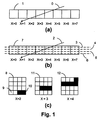•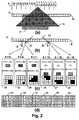•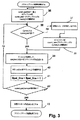•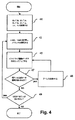•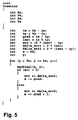•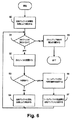•••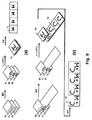•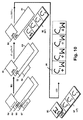••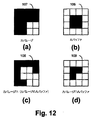•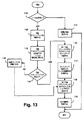•••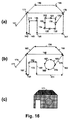•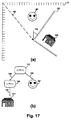•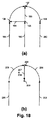•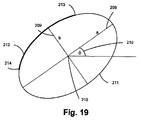•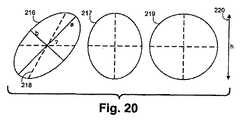•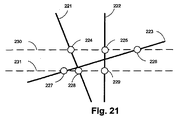•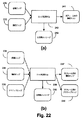•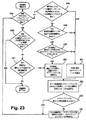•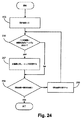•••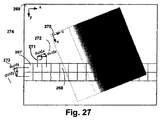•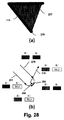•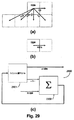•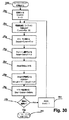•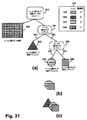•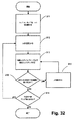•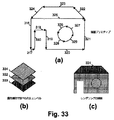•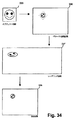•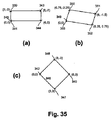•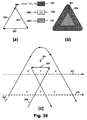•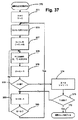•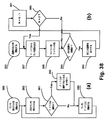•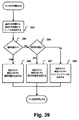•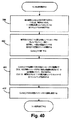•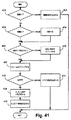•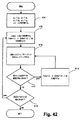•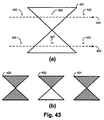•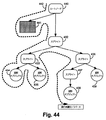••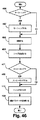•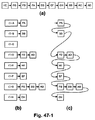•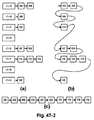•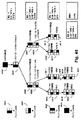•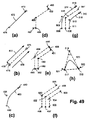••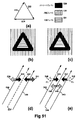•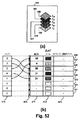•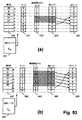•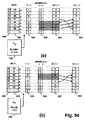•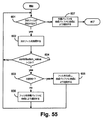•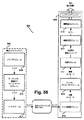••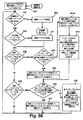•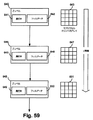••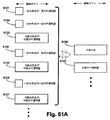•••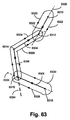•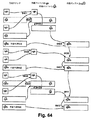•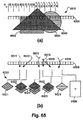•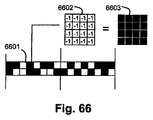•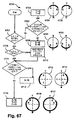•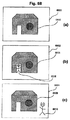•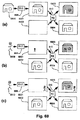•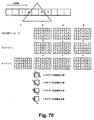•## Classifications

• GPHYSICS
• G06COMPUTING; CALCULATING; COUNTING
• G06TIMAGE DATA PROCESSING OR GENERATION, IN GENERAL
• G06T15/003D [Three Dimensional] image rendering
• G06T15/10Geometric effects
• G06T15/40Hidden part removal
• GPHYSICS
• G06COMPUTING; CALCULATING; COUNTING
• G06TIMAGE DATA PROCESSING OR GENERATION, IN GENERAL
• G06T11/002D [Two Dimensional] image generation
• G06T11/40Filling a planar surface by adding surface attributes, e.g. colour or texture

## Abstract

In general, an imaging engine system (699) for replaying a graphical object image using a device with limited computing resources, such as a so-called “thin client”, is disclosed. Many developments related to conventional image processing and rendering enable high quality image generation. One such development uses temporal coherence between one frame and subsequent frames in the animation sequence. In particular, some edges (233, 235) of graphic objects often remain “static” over several consecutive frames. An example of this is an edge used to draw details of an image background. In another development, anti-aliasing is used so that sub-pixel resolution coverage bit masks (A buffers 29-34) are generated for a limited number of scan lines at a time during the scan line rendering of a graphic object image. Done. Preferably, the A buffer is generated for only one pixel at a time. Yet another development relates to rendering a scan line of a graphic object image with a scan line renderer for a span of pixels lying between two consecutive edges with respect to x that intersect the scan line. For this span of pixels, this evolution maintains a subset of the depth that exists in the rendering result, this subset exists on the span and is maintained in depth order (590), and the corresponding depth is no longer active This is the depth to be removed in some cases. In yet another development, the composite stack (6101-6107) of image layers to be rendered in raster scan is simplified. Rendering can operate on a run of two or more pixels where the relationship between the graphical objects contributing to the layer is not changed. The layers are first divided into groups (6110, 6112, 6114), and each group is separated by layers (6111, 6113) with variable transparency. Of these groups, in the top group, the layer that is constant in color within the run is reduced to a single equivalent color (6115, 6116, 6117) with an associated cumulative contribution. Many other developments are also disclosed.

## Description

This application is filed on June 26, 2003 under 35 USC 35 USC and is hereby fully incorporated by reference as if set forth in its entirety herein. Claims priority based on Australian patent application 2003903448.

This specification includes contents that are subject to copyright protection. The copyright owner has no objection to the reproduction of the relevant material from this specification or the relevant patent office file for review purposes, but shall retain all other copyrights.

The present invention relates generally to rendering graphical objects, and more particularly to solving z-orders of graphical objects to accelerate rendering.

Raster image processors are fundamental in the field of computer graphics. A raster image processor (RIP) takes graphic primitives as input and produces an array of pixel values on a raster grid as output. This specification considers a 2D rendering system.

Two important classes of RIP are object-based RIP and scanline-based RIP. The difference between these two classes of RIP is in each inner loop, ie the entity on which each algorithm iterates. An object-based RIP iterates over every graphic primitive that must be rendered, whereas a scanline-based RIP iterates over each scanline that is to be rendered in raster order.

Consider the case of rendering a set of polygons that are distinguished from each other in terms of their contour, fill and rendering depth (ie z-order). Object-based RIP typically iterates over this single polygon set in z-order and renders each polygon in turn. This is the “painter's algorithm” approach. In contrast, scan line RIP examines each scan line in turn to determine where each polygon edge is on the current scan line. The pixel span between the edges that intersect the current scan line is then filled.

One improvement of scanline based rendering systems is in the use of an active edge list. In such an approach, instead of considering every polygon edge on every scan line, the scan line RIP maintains a list of only the edges that intersect the current scan line, and those edges are Chase. This approach typically uses a forward difference technique, where the edges are tracked by a form of Bresenham's scan-to-conversion algorithm.

New edges may be added to the list of active edges at the start of rendering of each scan line. In doing so, sorting is important to significantly reduce the number of edges to consider when adding to the active edge list. Specifically, the entire set of edges is generally arranged in raster order of the starting point. Here, the start point means the end point first encountered in the entire scan. As a result, if there is an edge being activated at the beginning of the scanning line, it is possible to quickly determine which edge it is. If it is determined at the end of a scan line that an edge does not intersect the next scan line, that edge is removed from the active edge list.

Consider a typical scan line under the RIP described above. Assuming that the x-coordinates of all edges that intersect this scan line are known and it is desired to output raster image data for this scan line, the next problem to be solved is the pixel span between the intersections. It is to determine the contents of the raster data. That is, spans between intersections that are consecutive in the x order are mapped from the raster image space to a single polygon set in the graphic model space. This approach allows for the inability of RIP to perform anti-aliasing. In addition, this method means that overlapping polygons are distinguished from each other by their depths. That is, in one polygon set exposed within the span between intersections, the top polygon is the only polygon rendered across that span if it is completely opaque. If the top polygon is not completely opaque (i.e. contains some transparency), the polygon below it also contributes to the span.

Scanline rendering eliminates the need for a screen (frame) buffer to store rendering results, which is a common feature and defect in object-based (painter's algorithm) rendering systems. However, the scanline rendering system can now be further characterized by considering how to solve the above-mentioned problems. Specifically, some scanline rendering systems alleviate the need for a complete screen buffer to scanline buffers, while other scanline rendering systems do so by eliminating the need for scanline buffers. It is possible to render directly to a lower output raster data buffer.

It is appropriate to consider various line buffer RIP models. First, there is a RIP that can maintain a line buffer of raster image data. Edges are sorted by y coordinate (ie, scan line) rather than x coordinate (pixel position in the scan line), and spans are stored in the buffer in a random pattern. That is, the order in which spans are rendered is not related to their respective x-orders. In such a system, there is no z-order concept such that when a pixel is written, a high grayscale output value takes precedence over a low value. After all spans have been accumulated for the current scan line, they are written from the line buffer to the output buffer in x order.

Variations of the above RIP model can be seen in other scanline based RIPs that maintain a line buffer at the intersection instead of raster data. Again, the edges are sorted by y instead of x. For each scan line, all intersections are determined and then sorted by x. In other words, the line buffer at the intersection is maintained instead of the raster data line buffer. Note again, however, that the line buffer of crossing messages is stored in a random order.

The third class of scan-line based RIP has neither a raster data line buffer nor a crossing message line buffer. This can be avoided by sorting all edges in full raster order prior to rendering. That is, all edges are sorted by starting x-coordinate and y-coordinate. As each pixel in the scan line is considered, the list of edges is checked for any edges that are becoming active.

Next, consider the problem of determining the contents of raster data for a given scan line, which is a problem common to all scan line renderers that support overlapping polygons separated by depth. As introduced above, one constant polygon fill set ordered by its depth potentially contributes to the span. Assuming that each polygon has a single fill that inherits the depth of the polygon, this problem can be considered in terms of the fill and the depth at which they occur.

In a system that considers spans in x-order, solving this problem requires maintaining a table of currently active depths as rendering progresses from span to span. Span to span evolution occurs on any pixel that is crossed by an edge. That is, a span is a section on the current scan line over which one currently activated depth set is constant. Active means that a conceptual cross section through all polygons that intersect the span includes all active depths (ie, all graphic objects that contribute to or affect the final pixel value).

An active depth set allows determination of the raster output of a span if it is maintained in descending depth order. If the fill cannot contain transparency, the top fill in the active depth table is the top and is the only fill that contributes to the fill raster data. If the transparency can be included, then the depth related fill in the table can also contribute to the raster output.

Solutions to the problem of maintaining this currently active depth table exist in the prior art. For example, a complete status table of all the depths present in the page image to be rendered can be created. This method is characterized in that the depth exists in the linear address memory, and when a fill exists at a certain depth, the position in the memory where the fill data can be stored is implied from the depth. In other words, there is one complete slot set for pointers pointing to fill information, one for each depth, and the slots are arranged in depth order. Inserting a fill into such a table is easy because there is always a slot for a given depth. However, the slot occupancy pattern is generally sparse. In practice, the depth of the fill is used to index the complete table of this depth slot when considering the fill and depth referenced by edge traversal. This is essentially an order one operation and allows the RIP to operate at real-time speed.

A cache, which can be referred to as a summary table, can be used to improve the speed of lookup to such a complete table. This is necessary because in general the total number of depths present on a page can be very large. For example, some of the real PostScript print rendering operations have a very large number of depths. Another result of a very large size full depth slot table is that the hardware implementation of this RIP model often cannot maintain a complete list internally. Therefore, the table needs to be held in the external memory, but in this case, the reference is delayed. If there are more depths than the depth slot table can accommodate, additional tables can be swapped in at the cost of a significant increase in complexity.

Furthermore, a RIP with this model is not easily adapted to animation, an application in which the set of depths filled with fill changes from frame to frame. Also, if the input to the animation renderer is not deterministic, the occupied depth set is not deterministic. Many depth slots must be set aside for fill found on future frames. Alternatively, the fill may be given different depths from frame to frame, but in the prior art RIP class currently under consideration, the fill is tightly coupled in combination with the corresponding depth. That is, the fill has a suggested depth by being at a particular memory location. As a result, fill reassignment may involve significant movement of data, which is undesirable.

Further defects are found in maintaining an active depth table in the context of composition. Inserting a new fill into the summary table is not expensive because the fill has the same depth as the table of all depth slots found on the page, and each fill has a corresponding waiting slot. However, when spans are combined, the summary table is very large and usually very sparse. On the other hand, one fillet to be considered by the compositor is generally relatively small and compact and therefore expensive to operate. In practice, a depth encoder that is as deep as the status table consumes a lot of complexity and silicon. The encoder then creates a single compact fill set that is synthesized over the span in question by the process of considering one active fill set ordered in depth as detailed above. Used for.

It is an object of the present invention to significantly overcome or at least improve one or more disadvantages of existing configurations.

According to one aspect of the present invention, there is provided a method for rendering a scan line of a graphic object image by a scan line renderer for a pixel span between two consecutive edges in x-order that intersect the scan line. Is characterized by maintaining a subset of the depths present in the rendering result for the span of pixels, where the subset is the depth present in the span and is maintained in depth order, and the corresponding depth is not active The depth is removed.

Preferably, this subset of depth is maintained by a content addressable memory.

According to another aspect of the invention, there is provided an apparatus for implementing any one of the foregoing methods.

According to another aspect of the invention, there is provided a computer program product comprising a computer readable medium having recorded thereon a computer program for implementing any one of the aforementioned methods.

Other aspects of the invention are also disclosed.

At least one embodiment of the present invention will be described with reference to the drawings.

Table of contents Introduction 1.1 Coordinate space 1.2 Graphic object 1.3 Glyph 1.4 Z level 1.5 Stroking 1.6 Morphing Driver module 2.1 Sprite 2.1.1 Sprite: transformation matrix 2.1.2 Graphic object and its depth 2.2 Display list 2.2.1 Frame rendering 2.2.2 Graphic object and z level 2.2 .3 Local depth and absolute depth Transformation, Morphing and Stroking 3.1 Morphing 3.2 Transformation 3.3 Edge Generation 3.4 Stroke Edges and Decomposition into z Levels 3.4.1 Straight Edge Stroking 3.4.2 Curve Edges Stroking 3.4.3 Seam stroking 3.4.4 Stroking of equal or opposite edge seams 3.4.5 End-cap generation of path end points 3.4.6 Stroke edges and unstroked End cap between edges 3.4.7 Assign z level to opaque stroke 3.4.8 Assign z level to transparent stroke 3.4.9 Transform stroking primitives 3.5 Filtering 3.6 Generation of edge tracking parameters 3.6.1 Generation of straight edge tracking parameters 3.6.2 2 Generation of Next Bezier Curve Tracking Parameters 3.6.3 Sign Determination 3.6.4 Generation of Elliptic Arc Tracking Parameters 3.6.5 Generation of Glyph Edge Tracking Parameters 4. 4. Sorting Edge processing 5.1 Input and output 5.2 Top-level operation 5.3 Active edge tracking 5.4 Examples of edge processing 5.5 Edge conversion to active edge and edge persistence 5.5.1 Static edge persistence Stance 5.6 Active Edge Processing 5.7 Edge Tracking 5.7.1 Straight Line Tracking 5.7.2 Quadratic Bezier Curve Tracking 5.7.3 Quadratic Polynomial Fragment Tracking 5.7.4 Elliptic Arc Tracking 5.7.5 Glyph tracking 5.8 Anti-aliasing and cross message generation 5.8.1 Cross message generation for glyphs 5.9 Cross message example 5.9.1 Other examples 5.10 Reorder active edges and crossing messages Z-level activation module 6.1 One ordered Z-level set of interest 6.2 Control flow in Z-level activation module 6.3 Z-level activation and deactivation: flexion count 6.4 One ordered Z level set of interest: continued 6.5 Adding a new Z level to one ordered Z level set of interest 6.5.1 One ordered Z level set of interest in hardware 6.6 Run processing 6.7 Conversion of S buffer to A buffer: Bending rule 6.8 Run processing: continued 7. Synthesis module 7.1 Intermediate 7.2 z-level fill 7.3 Basic flow 7.4 Graphical overview 7.5 Contribution calculation 7.6 Bottom-up synthesis 7.7 Top-down synthesis 7.8 Other synthesis methods 7. 9 Advantages of top-down 8. Pixel Generation 8.1 Linear Gradient Pixel Generation 8.2 Radial Gradient Pixel Generation 8.3 Bitmap Image Pixel Generation 9. Pixel Extraction 9.1 Input Data 9.2 Output to Frame Buffer 9.3 Direct Output to Display 9.4 Half Toning 10. Implementation 1. Introduction This document describes a thin client imaging engine (TCIE), a system that renders 2D graphical objects using minimal computer resources. Examples of where such resource levels may apply include portable devices or those with small displays, such handheld computing devices include mobile phones and portable game machines, printers and Includes office equipment such as copiers. A top level diagram of the TCIE system 699 is shown in FIG. 56, where the TCIE system 699 is configured as a pipeline of processing modules. Each module will be described in the order of data flow in the system. These modules are conceptually divided into a display list compiler 608 and a rendering engine 610. The display list compiler 608 prepares information representing the desired output, and the rendering engine 610 uses this information to generate the output image (ie, render to a display device or frame buffer). The TCIE system 699 can be used to generate a series of output images dispersed in time, and these output images are hereinafter referred to as “frames”. This use of the TCIE system 699 creates the effect that an animation (or “movie”) is played on the output display.

1.1 Coordinate Space Referring to FIG. 56, the first module of system 699 is a driver module 615 that maintains a collection of graphical objects and information about them. FIG. 34 shows the space used by the system 699. In FIG. 34, first, a graphic object shown in the object space 335 is shown. The same graphic object is then shown being transformed into the global logical space 336. Then, the same graphic object is shown being converted into the rendering space 337, and finally the same graphic object is shown being converted into the display space 338.

The conversion from the object space 335 to the global logical space 336 is realized by arrangement conversion of graphic objects. This arrangement conversion may be a product of a hierarchical structure of a conversion matrix described later. The transformation from the global logical space 336 to the rendering space 337 is achieved by a field transformation that transforms global logical coordinates into subpixels (for anti-aliasing purposes). The conversion from the rendering space 337 to the display space 338 is realized by an anti-aliasing process that creates a display pixel from sub-pixels that are constituent elements. In the case of degeneration in the one-to-one antialiasing process, the rendering space 337 and the display space 338 are the same, that is, the logical space 336 can be directly converted into the display space 338.

1.2 Graphic Object The input to the system 699 consists of a set of graphic objects and associated metadata. FIG. 16 (c) shows that the graphic object 171 is rendered on the display, and the corresponding components are shown in FIGS. 16 (a) and 16 (b). A graphic object is a two-dimensional display primitive described as an ordered set of drawing primitives consisting of one or more drawing primitives of new drawing positions, lines and curves. The drawing primitive indicates a part of the outline of a certain graphic object. Each primitive is associated with one or more coordinates. The new drawing position can be specified as an absolute offset from the base point of the object or a relative offset with respect to the end point of the previous primitive. The new drawing position is shown as a single coordinate, a straight line as a pair of coordinates, and a curve as a series of three coordinates. A straight line uses a pair of coordinates to define the start and end points of the line. The curve is implemented as a quadratic Bezier curve in which the first coordinate defines the start point of the Bezier curve, the second coordinate defines the control point, and the third coordinate defines the end point of the Bezier curve. Bezier curves are well known to those skilled in the art.

Edge coordinates are stored as an ordered set of relative coordinates, which reduces memory storage requirements and also determines the edge direction. It is suggested that the first coordinate of the line or curve is the last coordinate of the previous drawing primitive. Table 1 is an example of one ordered primitive set that can form the display object shown in FIG. In this example, the Y coordinate increases downward and the X coordinate increases to the right. The terms corresponding to the new drawing position, straight line, and curved line are MOVETO_ABS, MOVETO_REL, LINETO, and CURVETO, respectively. The starting point in this example is (0,0) 141.

1.3 Glyphs
A glyph is a special type of graphic object that has the additional constraint that it is always written directly into the display space. Glyphs are designed for situations where the shape to be rendered is (i) small and (ii) designed to be placed exactly on the pixel boundary.

Examples of shapes that are properly represented in glyphs include characters in hinted fonts.

Glyphs are represented as 1 bit per pixel bitmaps with two associated fills instead of passes. This pit map acts like a mask, displaying an “on” fill when a bit is set, and an “off” fill when no bit is set.

1.4 z level The z level is a display primitive used to describe how the portion of the display surrounded by a subset of the edges of the graphic object is colored. For example, the z level can be described as the enclosed area being filled with a single color. The z level is also assigned an absolute depth, which is an integer value used to specify which z level is displayed above which z level. A z level with a higher absolute depth is rendered on top of a z level with a lower absolute depth.

A maximum of two z levels are associated with line and curve drawing primitives. That is, if there are two z levels, the first z level is rendered to the left of the primitive drawing direction, and the second z level is rendered to the right of the primitive drawing direction. FIGS. 33 (a)-(c) illustrate this concept by showing the left and right fills used to create the graphic object 331 previously illustrated as the object 171 in FIG. 16 (c). ing.

FIG. 33A shows drawing primitives 316 to 329. These primitives 316 to 329 refer to the z levels 333, 332 and 334 shown in FIG. The z level is shown in absolute depth order. That is, it can have an absolute depth of 2, 3 and 4, respectively. Table 2 is a table showing which z level the drawing primitive refers to. For example, LINETO 316 is downward and has a z level 332 on the left side of the drawing direction. The rendering result is shown in FIG.

The z-level style can be, but is not limited to, a single color, a linear blend described with one or more colors, a radial blend described with one or more colors, or a bitmap image. All these z-level styles also support a transparency (alpha) channel. The z levels 333, 332, and 334 in FIG. 33 represent the single color style z level. These z levels are used unchanged throughout most of the pipeline.

1.5 Stroking Drawing primitives can be associated with pen width. A drawing primitive having a pen width is converted into a plurality of edges (the edge has no width). These edges form a closed and filled shape that represents the stroke of the pen. See the section entitled “Transformation, Morphing and Stroking” for a detailed discussion.

1.6 Morphing Morphing is also well known in the art. Morphing can be defined as supplying two drawing primitive sets for a graphic object and a ratio that specifies that the graphic object is drawn by interpolation between the two sets. This is also discussed in detail in the section entitled “Morphing, Stroking and Conversion Module”.

2. Driver Module The driver module 615 is discussed in terms of the information it handles and what information it passes to the rest of the rendering pipeline. The role of the driver module 615 is to collect a collection of drawing primitives. Drawing primitives can first be collected into graphic objects as described above.

Graphic objects can be grouped into sprites as described below. These sprites can be specified as having properties that apply to the entire aggregate. The main role of the driver is to enable efficient high-level operations on the sprite without complicating the rest of the graphics pipeline. When the driver module 615 outputs drawing information for subsequent modules in the graphics pipeline, certain sprite properties (eg, its transformation matrix) are applied to each drawing primitive of that graphics object. This allows subsequent modules to only handle directed edges and z levels.

2.1 Sprite Driver module 615 accepts a sprite as part of the input. Sprites are well known in the art, and driver module 615 refers to primitives with transformation matrices, depths, and a list of graphical objects that exist within the context of the sprite. Sprites can include zero or one or more graphic objects and zero or one or more sprites. Here, “include” means that the transformation matrix of the sprite is applied to all the graphic objects and sprites owned by the sprite in question. The concept that a sprite contains other primitives also means that the depth of all graphic objects and sprites that the sprite contains is “local” to the sprite. The graphic object does not include other graphic objects or sprites.

2.1.1 Sprite: Transformation Matrix Transformation matrices are well known in the art. A sprite's transformation matrix is applied to all graphic objects owned by the sprite and defines the sprite's local space. In FIG. 17 (b), two sprites and two graphic objects are provided, and the method to be used in their rendering is described by a tree. The sprite 185 includes both the sprite 189 and the graphic object 180. That is, links 188 and 186 represent ownership relationships. The sprite 189 includes a second graphic object 182.

FIG. 17 (a) shows the geometry of the transformation matrix present in this example. Space 179 includes the objects in sprite 185. The object 180 can be seen to be located in the space 179, and the coordinates of drawing primitives that are constituent elements of the object 180 refer to the space 179. The sprite 189 of FIG. 17B is also located in this space 179. A space 181 represents a space where the graphic object of the sprite 189 is located.

In this example, sprite 189 has a transformation with rotation, translation and scaling. Translation is represented by the dotted line 183, rotation by the angle 184, and scaling by the relative size of the axis scales 179 and 181 used to represent the space of the sprites 185 and 189, respectively. In this example, the scaling is the same for any axis.

Still referring to FIG. 17A, a transformation matrix that represents the arrangement of graphic objects owned by a sprite is connected to a transformation matrix that describes the arrangement of the sprite. In the example of FIG. 17A, the transformation matrix applied to the graphic object 182 is a concatenation of the transformation matrices of the sprites 189 and 185. This composite transformation matrix for object 182 applies to all graphic primitives that object 182 contains.

2.1.2 Graphic Object and Its Depth The depth of a graphic object is local to the sprite that contains the object. This is illustrated in FIGS. 31 (a) -31 (c). In the rendering tree shown in FIG. 31A, a node 294 represents a sprite including another sprite 296 and a graphic object 302. The ownership relationships are indicated by directed lines 295 and 301, respectively. Similarly, the sprite 296 includes graphic objects 298 and 299 indicated by ownership relationships 297 and 300, respectively. Table 3 shows the local depth of all these primitives.

The concept of local depth can be clarified by looking at the appearance of the subtree in FIG. FIG. 31 (b) shows the visual appearance of sprite 296 (rendered separately as objects 298 and 299). Since object 298 has a smaller depth value than object 299, object 298 appears below object 299. FIG. 31C shows the appearance of the graphic object 302 and the sprite 296. Since the depth value of the graphic object 302 is smaller, it can be seen below 296. Note that the local depth of the children of the sprite 296 is preserved according to the local depth legend 304.

Node 303 is the root of the ownership tree and represents a sprite that includes all top-level sprites. The root 303 owns a background z level 309, which is always at its lowest local depth (which is also the lowest global depth). Therefore, this background z level is lower than all graphic objects.

2.2 Display List The list of all sprites and graphic objects in a single frame, including their ownership, local depth and transformation, is called the display list. Display lists are well known in the art. The driver module 615 maintains the display list in the form of a tree. This tree is a collection of sprites and graphic objects according to ownership relationships, which are ordered according to local depth. FIG. 31A also shows this configuration. For example, the children of sprite 294 are ordered according to local depth. The first child 302 is at the lowest local depth 1 and the next child 296 is at the higher local depth 2.

2.2.1 Frame Rendering In each frame of the animation, the display list can be modified before it is rendered on the display. The display list is held between frames.

2.2.2 Graphic objects and z levels Just as sprites can reference multiple graphic objects, graphic objects can reference multiple z levels. Each of these z levels has a depth in the graphic object. For example, the graphic object FIG. 33 (c) requires three z levels with depths as shown in FIG. 33 (b).

2.2.3 Local Depth and Absolute Depth Local depth is used in the driver module 615, but subsequent modules require absolute depth. Driver module 615 assigns an absolute depth to each z level.

The z-level absolute depth of the graphical object is passed from the driver module 615 to the subsequent module, where it is simply called the depth. Of course, all subsequent modules handle z levels that are assigned an absolute depth. These assignments are made for each frame immediately before rendering. This is only done when a new display object or sprite is inserted into the display list or when the old one is discarded.

If FIG. 31 (a) shows all primitives in a single frame, Table 3 above shows the absolute depth of all primitives in FIG. 31 (a). The absolute depth of all z frames in a single frame starts with 1, which is assigned to a special background z level. The z level is numbered with an integer that continues upward from the background z level. A sprite does not have an absolute depth itself (although information about the depth of its descendants can be recorded). A sprite exists as a container for information (for example, arrangement conversion) regarding a graphic object included in the sprite, but is not a graphic object itself. Subsequent modules in the rendering pipeline do not use sprites. Further, the graphic object does not exist outside the driver module 615. The local depth of the graphic object is used to calculate the absolute z-level depth referenced by the drawing primitives that make up the graphic object.

The absolute depth is calculated once the contents of the display list are rendered once per frame. A method for calculating these absolute depths is shown in FIG. 44, which shows a display list tree. The root node 440 of the display list tree owns a background z level 441 that is subordinate to all other z levels. That is, the background 441 has 1 which is the lowest absolute depth. Nodes 432-439 constitute the remainder of the display list tree.

Absolute depth is assigned to each z level of each graphical object during the depth-first traversal of the tree, indicated by dotted line 442. The assignment of absolute depth to the z level of a graphic object starts at the lowest z level in the graphic object, which is one absolute depth higher than the highest z level of the "immediate" graphic object. Is assigned. Here, “immediately before” means that the graphic object is the graphic object visited immediately before by the depth-first traverse. FIG. 44 shows the depth-first traverse reaching the graphic object 438. When assigning an absolute depth to the lowest z level of the object 438, an absolute depth one higher than the highest z level in the graphics object 435 visited immediately before is assigned.

If the object 438 has further z levels, the absolute depth is assigned sequentially from the z level immediately above the lowest z level to the highest z level. The reader will recall that all sprites and graphic objects have independent local depth ranges and are therefore not interleaved. The absolute depth is stored in all nodes of the display list tree. The graphic object stores the absolute depth of its highest z level. The sprite stores the absolute z-level absolute depth of its descendants. Tree traversal always starts from the root.

If only a part of the display list is changed due to insertion or removal of nodes, a minimal update of the tree is performed to increase efficiency. During traversal of the display list tree, absolute depth updates are performed only from the first node where the change is made. Thus, the z level of the previous graphic object (in traverse order) retains its effective absolute depth. Until the first change node is reached, absolute depth tracking is done by looking at the highest absolute depth of each node. Upon encountering the first change node, absolute depth assignment continues from this noted value.

For example, in FIG. 44, if object 438 is a new node in the display list tree, and this was the only change from the previous frame, absolute depth assignment starts at object 438.

3. Transformation, Morphing and Stroking As input, the transformation, morphing and stroking module 616 of FIG. 56 receives from the driver module 615 an ordered one or more that defines one or more outlines of the drawing object to be rendered. Take multiple sets of coordinates. Each set of ordered coordinates can be accompanied by additional parameters, including:

-Morph ratio-Transformation matrix-Stroke width-Left z-level reference-Right z-level reference, and-Stroke color z-level reference Morph ratio, transformation matrix and stroke width are ordered to describe the contour of a graphic object Describes how the coordinate set should be placed on the display. The remaining parameters are multiple z-level references that indicate the color, blend, or texture of the display pixels within the contour defined by the ordered set of coordinates.

FIG. 46 is a flow diagram illustrating the processing that module 616 performs for each ordered stepwise coordinate set. The purpose of this process is to transform each ordered stepwise coordinate set into a corresponding ordered one edge set, each described by at least start and end coordinates in rendering space ( See FIG. 16 (a)). That is, the edge has directionality. The z-level left and right references owned by the edge relate to this directionality. Morph ratio, transformation matrix and stroke width parameters are used to control this process.

Further, module 616 optionally accepts one or more glyph descriptions. These descriptions include the following information:

-Glyph position-glyph height and width-glyph bitmap-"on" z-level reference (possibly null)
-An "off" z-level reference (possibly null), and-a glyph description enters the pipeline at stage 470.

3.1 When the morphing module 616 receives an ordered set of coordinates corresponding to a morph object (ie, “yes” at stage 464 in FIG. 46), it receives two versions of each coordinate along with the morph ratio parameter. . One version of each coordinate received is called the starting version. The second version of each coordinate received is called the end version. Module 616 uses stage 467 to obtain a single intermediate version of each coordinate received by interpolation using the morph ratio parameter. For example, FIGS. 35 (a) and 35 (c) show ordered coordinate sets representing the morph “start” and morph “end” shapes, respectively. Any ordered coordinate set is shown starting and ending at the origin 342 in the “logical” coordinate space. The purpose of the morph process is to interpolate between the “starting” version of FIG. 35 (a) and the “ending” version of FIG. 35 (c), to obtain this shape as shown in FIG. 35 (b). Create an intermediate version. The ("start" and "end") version of each coordinate is provided as an ordered set of coordinate sets, in this example 339 and 346 are the first pair, after which 343 and 340, 344 and 347, The last pair 341 and 342 follows. The morph ratio is used to interpolate an intermediate version from each coordinate pair. If a morph ratio of 0 is used to represent the “start” shape of FIG. 35 (a) and a morph ratio of 1 is used to represent the “end” shape of FIG. The morph ratio corresponds to the shape shown in FIG. Thus, the morph process creates an ordered set of multiple coordinates as indicated by 350, 351, 352 and 353 as outputs.

3.2 Transformation Next, in FIG. 46, a transformation matrix is applied to the coordinates at step 468. Using a transformation matrix, it is possible to specify rotation, scaling, and / or translation of coordinates (and therefore drawing objects). In this description, the transformation matrix is said to transform coordinates (and hence the drawing objects they describe) from “logical space” to “rendering space”.

3.3 Edge Generation After the ordered coordinate set has been morphed and transformed (if appropriate), in a subsequent stage 465 of the flowchart of FIG. 46, the ordered coordinate set is converted to an ordered edge set. . An example of how stage 465 is implemented is shown in the flowchart of FIG. In this example, assume that the coordinates can form part of one of the three drawing primitives consisting of a straight line, a quadratic Bezier curve, and a new drawing position. This example also assumes that additional information (eg, a tag byte) is provided that indicates what type of drawing primitive follows and when the end of the drawing primitive is reached.

First, as shown in FIG. 15, in step 140, the current drawing position is initialized to (0, 0), and then in steps 124, 125, 126, and 127, the type of “subsequent” drawing primitive that is encountered next. Is determined.

If the above type indicates that the subsequent coordinates represent a new drawing position (step 124), the coordinates are read in step 128 and used to set the new current drawing position in step 131.

If the type indicates that subsequent coordinates represent a straight edge (step 125), then a new straight edge is generated at step 129 given a starting coordinate equal to the current drawing position. The coordinates are then read in step 132 and used in step 134 for the new straight edge end coordinates and in step 136 for the new drawing position.

If the type indicates that the following two coordinates represent a curve (eg, a quadratic Bezier) (step 126), a new curve is generated at step 130 given a starting coordinate equal to the current drawing position. The first coordinate is then read at step 133 and used as the “control point” coordinate of the new curve at step 135. The second coordinate is then read at step 137 and used for the new curve end coordinate at step 138 and the new drawing position at step 139, respectively.

At step 127, processing continues until the end of the ordered coordinate set is reached.

3.4 Decomposition of Strokes into Edges and Z Levels Returning to FIG. 46, at step 471, the next step of the process is optionally available, which is the stroking step 466. Stroking is the process of generating one or more contours that simulate the effect of using a pen with a given thickness when tracing along curves and vector paths. is there. Examples of stroking are shown in FIGS. 50 (a)-(e). FIG. 50 (a) shows an ordered set of three edges 523 to be stroked. In FIG. 50 (b), the generated stroke contour 524 is shown, which traces the path of three edges to be stroked with a pen with a circular tip having a given pen width 525. Bring effect.

46 suggests that the stroking step 466 must be performed after the conversion step 468, but allows the stroking to occur before the conversion, thereby producing different results. It may also be desirable. For example, referring again to FIG. 50 (a), if the original shape represented by edge 523 is first transformed to create the shape 526 shown in FIG. 50 (c) and then stroked, the result is A shape 528 shown in 50 (d) is obtained. However, if the original shape represented by edge 523 is first stroked 524 and then the same transformation is applied, the result is the shape 527 shown in FIG. 50 (e). The TCIE system 699 can achieve either result by optionally performing the coordinate transformation of step 468 after stroking 466.

In the following discussion on stroking, stroking refers to a coordinate system in which the positive Y axis is offset 90 degrees clockwise relative to the positive X axis (eg, X increases to the right and Y is downward Note that it is assumed that the

Module 616 performs stroking of the ordered edge set by passing through the edges in the original ordered edge set one after the other. For each edge, a new left edge and a new right edge are created whose centered and traced tip along the path of the original edge corresponds to the left and right limits of the circular pen stroke. An elliptical arc is used to generate a seam between two successive original edges and between the start and end end-kips of the original ordered edge set that does not form a closed form. The ordered edge set is closed only if the start point coordinates of the first edge of the ordered edge set are equal to the end point coordinates of the last edge of the ordered edge set.

The arc is placed as an arc in the object space and then converted to an elliptical arc in the display space by the process described in a later section. Note that this primitive is currently only used internally, but arcs can be easily exposed as drawing primitives available to the user.

3.4.1 Straight Edge Stroking FIG. 49A shows an example of a straight edge 473 to be stroked represented by a start point coordinate 474 and an end point coordinate 472. FIG. 49 (b) shows the desired result of stroking the straight edge 473, which includes a left edge 477 and a right edge 478.

When stroking a straight edge, a left-hand normal vector 479 having a direction of 90 degrees counterclockwise with respect to the direction of the straight edge and having a length half the pen width of the stroke is generated.

The left and right stroke edges are then calculated using the coordinates 474 (Xs, Ys) and 472 (Xe, Ye) of the straight edge 473 and the left normal vector 479 (Xn, Yn).

At the left stroke edge 477, the start point coordinates (Xs_left, Ys_left) and the end point coordinates (Xe_left, Ye_left) are calculated as follows.

Xs_left = Xs + Xn Ys_left = Ys + Yn
Xe_left = Xe + Xn Ye_left = Ye + Yn
The right stroke edge 478 is generated by subtracting the normal vector from the coordinates of the current edge.

Xs_right = Xs-Xn Ys_right = Ys-Yn
Xe_right = Xe-Xn Ye_right = Ye-Yn
3.4.2 Curve Edge Stroking FIG. 49 (c) shows a curve edge 483 to be stroked. A curved edge (second-order Bezier curve or quadratic polynomial fragment (QPF)) is represented by a start point 480, a control point 481, and an end point 482.

FIG. 49 (d) shows the two left normal vectors 487 and 488 that must be generated to stroke this edge. Both of the two left-hand normal vectors have a length that is half the pen width of the stroke. The first left normal vector 487 is 90 degrees counterclockwise with respect to the vector from the start point 480 to the control point 481. Second left normal vector 488 is 90 degrees counterclockwise with respect to the vector from control point 481 to end point 482.

Referring to FIG. 49 (e), a left stroke edge of a curve having a start point 493, a control point 494, and an end point 495 is generated. The left stroke edge start point 493 (Xs_left, Ys_left) is calculated by translating 480 the curve edge start point (Xs, Ys) by the first left normal vector 487 (Xn1, Yn1).

Xs_left = Xs + Xn1 Ys_left = Ys + Yn1
The end point (Xe_left, Ye_left) of the left stroke edge is calculated by translating 482 the end point (Xe, Ye) of the curved edge by a second left normal vector 488 (Xn2, Yn2).

Xe_left = Xe + Xn2 Ye_left = Ye + Yn2
The left stroke edge control point 494 is calculated using the intersection of two straight lines. The first straight line passes through the left stroke edge start point 493 and is parallel to the straight line from the curve edge start point 480 to the curve edge control point 481. The second straight line passes through the left stroke edge end point 495 and is parallel to the straight line from the curve edge end point 482 to the curve edge control point 481.

As shown in the example of FIG. 49 (f) showing the start point 503, the control point 504, and the end point 505, the right stroke edge of the curve is similarly generated. The start point coordinates (Xs_right, Ys_right) and end point coordinates (Xe_right, Ye_right) of the right stroke edge are calculated as follows.

Xs_right = Xs-Xn1 Ys_right = Ys-Yn1
Xe_right = Xe-Xn2 Ye_right = Ye-Yn2
The control point 504 for the right stroke edge is calculated using the intersection of the two straight lines. The first straight line passes through the right stroke edge start point 503 and is parallel to the straight line from the curve edge start point 480 to the curve edge control point 481. The second straight line passes through the right stroke edge end point 505 and is parallel to the straight line from the curve edge end point 482 to the curve edge control point 481.

FIG. 49 (g) shows the resulting left stroke edge 514 and right stroke edge 515 depicted for various control points.

The above technique is an approximation of curve stroking and is not suitable for curves with narrow angles such as curve 520 shown in FIG. 49 (h). In the triangle formed by the start point 517, the control point 518, and the end point 519 of the curve, the control angle is defined as the diagonal of the straight line connecting the start point 517 and the end point 519 (ie, facing each other). If the control angle is 120 degrees or less, the curve can be considered to have a narrow angle. A convenient test is to use the absolute value of the inner product of the two left normal vectors 521 (Xn1, Yn1) and 522 (Xn2, Yn2).
[Equation 1]
If (Xn1Xn2 + Yn1Yn2) 2 <1/2 (Xn1 2 + Yn1 2 ) * (Xn2 2 + Yn2 2 )
If so, the curve has a narrow angle.

A plurality of methods for dividing a quadratic Bezier curve represented by a start point, a control point and an end point into two equal parts are well known in the art. A curved edge that is determined to have a narrow angle is first divided into two equal curved edges and then divided again into two, resulting in a total of four curved edges. The outer two new edges (those not adjacent to the original narrow angle) are then stroked using the process described above. The inner two new edges are vectorized into a path of approximate linear edges using techniques well known in the art. This path is then stroked using the process described in Section 3.4.1.

3.4.3 Seam Stroking To stroke the region where the edges of the path join, additional curves need to be generated. A seam is defined as having an incoming edge and an outgoing edge. FIG. 45 (a) shows two straight edges forming a seam 444, where edge 443 is an incoming edge because the seam forms its end point, and edge 445 is a seam. Is the leading edge because it forms its starting point. The left stroke edges 446 and 447 and the right stroke edges 448 and 449 are generated using the process described above. The left normal vector for the end point of the incoming edge 462 and the start point of the outgoing edge 463 should have been determined. First, considering the left side, a test is performed to determine whether it is necessary to generate a curved contour for the joint connecting the end point 453 of the incoming edge and the start point 454 of the outgoing edge. The left-hand normal vector 462 having the component (Xn1, Yn1) for the end point of the incoming edge to be stroked and the left-hand normal vector 463 having the component (Xn2, Yn2) for the start point of the outgoing edge to be stroked are known. Assuming the following test can be used to determine if a curve outline needs to be generated.

If Yn1Xn2 <Yn2Xn1, a curved edge is used to connect the left edges of the stroke and a straight edge is used to connect the right edges of the stroke.

Similarly, if Yn1Xn2> Yn2Xn1, a curved edge is used to connect the right edge of the stroke and a straight edge is used to connect the left edge of the stroke.

If Yn1Xn2 = Yn2Xn1, the edges forming the seam have the same or opposite direction at the seam. The process in such a case is shown in section 3.4.4 below.

In the example shown in FIG. 45 (a), the above test should indicate that a curved edge needs to be generated on the left side of the seam. This curved edge is a true arc having the following properties. That is, the start and end points of the arc are points 453 and 454, respectively.
The radius of the arc is the length of the left vector 462;
-The center of the arc is the original pass point 444,
-The arc progresses clockwise from the start point to the end point (if the right side of the seam is processed, the arc progresses counterclockwise).

These values are used to generate elliptical arc edges, and further processing will be described later.

Continuing with the example shown in FIG. 45 (a), the above test generates a straight edge on the right side of the seam that connects the end point of the first right edge 451 with the start point of the second right edge 450. It should indicate that there is a need.

FIG. 45 (b) shows a situation where edges 455 and 456 on the same side of different stroke paths do not intersect. New edges can be generated that extend from the corresponding vertices 460 and 461 and are bent around the control points or centers associated with the edges 455 and 456. FIG. 45 (b) also shows corresponding opposing edges 457b and 457a that do not intersect. A new edge is inserted between vertices 458 and 459 to close the path. This is a straight edge because the effect on stroke fill is zero.

3.4.4 Stroking of equal or opposite edge seams In the description of the seam stroking technique described above, a test to show when two original edges forming a seam have equal or opposite directions Explained. That is, when (Xn1, Yn1) is the left normal vector at the end point of the incoming edge to be stroked and (Xn2, Yn2) is the left normal vector at the start point of the outgoing edge to be stroked,
Yn1Xn2 = Yn2Xn1
It becomes.

Furthermore, if Xn2 = Xn1, the left-hand normal vectors are equal, so the incoming and outgoing edges have the same direction at the seam. No additional edges are required for stroking the seam.

If Yn1Xn2 = Yn2Xn1, but Xn2 ≠ Xn1, the incoming and outgoing edges have opposite directions at the seam, so an end cap for the seam must be generated as described below.

3.4.5 Generating an end cap for a path end An end cap is generated for an end point of an unclosed path to be stroked. End caps must also be created for seams between edges when the incoming and outgoing edges are in opposite directions (as described above).

The process uses an elliptical arc to generate end caps for the endpoints. An example of a stroke end cap is shown in FIG. 18 (a), which shows that the original edge 192 of the path to be stroked ends at a point 193. Left stroke edge 195 and right stroke edge 200 are shown ending at end points 196 and 198, respectively. A left-hand normal vector 194 having components (Xn, Yn) at the end point of the finite edge is shown.

To generate the end cap, an elliptical arc with the following characteristics is placed.

-The arc begins at the left end 196 and ends at the right end 198,
-The center of the arc is the original pass point 193,
The radius of the arc is the length of the left normal vector 194;
-The arc advances clockwise around the circle.

When stroking a path that has a valid left z-level reference and / or a valid right z-level reference (in addition to a valid stroke z-level reference), it also includes an enclosed area in which the stroked path is to be filled. It shows that it forms. In such a pass, it is necessary to add two straight edges to any end cap.

In this case, a new left-hand normal vector 199 having components (Xn_knee, Yn_knee) equal to the left-hand normal vector at the end point of the finite edge rotated 90 degrees clockwise is generated. The inflection point coordinates 197 (Xknee, Yknee) are then generated using the new left-hand normal vector 199 with the coordinates and components (Xn_knee, Yn_knee) of the end points 193 (Xj, Yj). That is,
Xknee = Xj + Xn_knee Yknee = Yj + Yn_knee
FIG. 18 (b) shows two additional edges 207a and 207b, both starting at the inflection point 197 and ending at the end points of the original edge to be stroked. The first additional edge 207a is an additional left stroke edge, and the second additional edge 207b is an additional right stroke edge. A description of how z-value references are assigned to the left and right stroke edges will be given below.

3.4.6 Endcap between end of stroke and non-stroke edge In the endcap path, an edge that refers to the stroke fill is followed by an edge that does not refer to the stroke fill. . FIG. 28 (a) shows a partially stroked triangular shape. Vertex 275 is where stroked edge 276 intersects non-stroked edge 277. At vertex 275, an end cap is generated.

This end cap is created in the same way as shown in the previous section. That is, the same method used for the end cap generated at the end of the pass and the additional steps described below are used. The result of applying this method is shown in detail in FIG.

Consider the shape's internal fill, which is the left fill referenced by edge 278. From this, it can be seen that by adding a small end cap edge 280, the inner fill is surrounded by one edge set that is completely closed. The edges 279, 280, 282, and 278 are one edge set that surrounds the graphic fill in the joint area. Additional edges not shown enclose fills in non-seam areas. It is well known in the art that the method of filling a shape depends on the shape to be closed, especially if bending rules and bending counts are used to fill the area enclosed by the edges.

The edge 278 is the only edge that is not generated by the stroking method among the edges shown in FIG. This edge is taken from the incoming edge set and added to the outgoing edge set.

3.4.7 Assigning z-levels to opaque strokes If an ordered set of coordinates is provided as input and that ordered set of coordinates will form a stroke path, the left z-level reference and In addition to the right z-level reference, a valid stroke z-level reference and pen width is required as input. There is no difference between the z-level format used for the stroke and the z-level format used to fill the path. Once an ordered set of coordinates has been processed into an ordered set of edges, they can be stroked using the techniques described above. Module 616 creates one left stroke edge set and one right stroke edge set. If module 616 receives as input the left z level, right z level, and stroke z level, and the stroke z level is determined to be opaque, the created left stroke edge is assigned the following:

A left z level reference that references the left z level received as an input, and a right z level reference that refers to a stroke z level received as an input.

-A left z level reference that refers to the stroke z level received as input; and a right z level reference that refers to the right z level received as input. In this section, reference to the left or right stroke edge refers to the edge direction. This is a reference to the edge on the outer boundary of the stroke edge. In this regard, when this edge is pointing from the upper left to the lower right of the display, a left stroke edge appears on the right side of the edge.

When this z-level assignment is used, the original edge of the stroke path is discarded and thus completely replaced with the left and right stroke edges created.

An example is shown in FIGS. 51 (a) to (e). FIG. 51 (a) shows one input edge set that defines a closed path in the stroking method described above. The edges 533, 532, and 534 are all associated with the left z level 530, the right z level 531, and the stroke z level 529. For an opaque stroke, the edge output of the stroking method for the input edge 533 is shown in FIG. 51 (d), along with its own z-level assignment. The visual result of stroking the path shown in FIG. 51 (a) is shown in FIG. 51 (b).

Note that the created left stroke edge 535 has a left z level reference 538 that references the left z level 530 provided as input, and a right z level reference 539 that references the stroke z level 529 provided as input. I want to be. Also, the created right stroke edge 536 has a left z level reference 540 that references the stroke z level 529 provided as input, and a right z level reference 541 that references the right z level 531 provided as input. The original stroke edge 533 is discarded.

3.4.8 Assigning z-levels to transparent strokes The following describes an alternative method of z-level assignment as described above. This assignment is particularly suitable for the stroking of an ordered single edge set with a stroke z level with some transparency. In this z-level assignment, the left stroke edge created is assigned-a null left z-level reference, and-a right z-level reference that references the stroke z level received as input, and the created right stroke edge A left z level reference that refers to the stroke z level received as input, and a null right z level reference is assigned.

When this z-level assignment is used, the original edge is not discarded. That is, the output of the stroking process is the union of one original edge set, one left stroke edge set created and one right stroke edge set created. The original edge is assigned a left z level reference provided with the input left z level as a reference and a right z level reference provided with the input right z level as a reference.

FIG. 51A shows one input edge set in the above stroking method. The edges 533, 532, and 534 are all associated with the left z level 530, the right z level 531, and the stroke z level 529. For transparent strokes, the edge output of the stroking method for the input edge 533 is shown in FIG. 51 (e), along with its own z-level assignment. A visual result of stroking the path shown in FIG. 51 (a) is shown in FIG. 51 (c).

Note that the left stroke edge 542 created has a null left z level reference 545 and a right z level reference 546 that references the stroke z level 529 provided as input. The original edge 533 is not discarded and has a left z level reference 550 that references the left z level 530 provided as input and a right z level reference 549 that references the right z level 531 provided as input. Also, the created right stroke edge 536 has a left z level reference 547 that references the stroke z level 529 provided as input, and a null right z level reference 548.

FIG. 63 shows a path 6300 that is preferably stroked and formed by some directed edges 6302, 6304, 6306 and 6308. As shown, the oriented edges extend from and join at vertices 6310, 6312, 6314, 6316 and 6318.

In this example, path 6300 is desirably stroked according to the stroke width associated with each directed edge 6302-6306. In this regard, the directed edges 6304 and 6306 have the same stroke width and color so that these two edges together define a discrete portion of the original path 6300 to be stroked.

As previously shown in connection with FIGS. 18 (a) and 18 (b), artifacts can occur when stroking the end of an edge. Specifically, as shown in FIG. 63, when edges having different stroke widths intersect each other (for example, at vertices 6312 and 6316), all the edges can be synthesized from the edge information so that accurate synthesis can be performed. It is essential that accurate stroking is performed on the edges. In this regard, different stroke widths can cause one stroke to be composited over the other, which can lead to very serious errors if transparent strokes are used. is there. Furthermore, when using transparent strokes, less important but still serious errors can occur. To ensure that the path 6300 is stroked accurately, it is necessary to ensure that the individual stroke paths formed by the discrete edge portions of the path 6300 are accurately reproduced.

This is done by first identifying the vertices of the original stroke path 6300 where the strobe width changes. From FIG. 63, it can be seen that the strobe width varies at vertices 6312 and 6316. At all other vertices, the stroke width is constant and corresponds to the stroke width of the corresponding stroke path to be created. Furthermore, by identifying vertices whose stroke width changes, other vertices that do not change (eg, vertex 6314) can be accurately stroked. Once the vertices are properly identified, a separate stroke path can then be created for each discrete portion of the original path 6300. In the first discrete portion defined by edge 6302, stroke paths 6320 and 6322 each originate from vertex 6312, are on an extended portion 6338 of directed edge 6302, and are directed upwards on the page extending from vertex 6310. . The stroking of the paths 6320 and 6322 around the vertex 6310 may be performed by the method shown in FIG. The stroking of paths 6320 and 6322 around vertex 6312 is performed in the manner previously described in connection with FIG. Note that in FIG. 63, paths 6320 and 6322 extending from vertex 6312 (to be compared with edges 207a and 207b shown in FIG. 18B) are exaggerated. In particular, stroke paths 6320 and 6322 together enclose the corresponding discrete portion of the original path 6300 (formed by edge 6302).

A similar approach is performed for stroke paths associated with edges 6304 and 6306. In this case, a configuration corresponding to the configuration shown in FIG. 18B is incorporated at the end of each path defined by the vertices 6312 and 6316. Stroke paths 6328 and 6330 associated with edge 6308 are created in the same manner as the stroke path for edge 6302 was created. Significantly, in any example, the stroke path needs to pass through vertices identified as being located at points on the path where the stroke width varies.

An extended example of the configuration of FIG. 63 will be understood with reference to FIG. 51 (b) or 51 (c) showing a closed path. In the configuration of FIG. 63, a closed path incorporating discrete portions so that the inside of the path can be accurately filled using the negative bending and fill rules previously described with respect to FIGS. 51 (a)-(e). Can be stroked. Thus, replacing the original path edge with the edge defining the stroke path with the left and right fills, as described above, will replace the interior of the closed path, the resulting fill level and interior of the stroke part. Can be used to define.

3.4.9 Conversion of stroking primitives When stroking is performed in rendering space, there is no need to convert line segments, Bezier curves and arcs created by the stroking module. However, if stroking is performed in object space, these primitives must undergo the same transformation as the original edge of the shape.

Stroke lines and Bezier lines are transformed in the same way as for shape lines and Bezier curves, as discussed in Section 3.2. On the other hand, stroke end caps and seams have to go through a different procedure because applying an affine transformation to an arc results in a generalized elliptical arc.

FIG. 19 shows a generalized elliptical arc. The lower ellipse 211 can be represented by a major axis 208, a minor axis 209, a center point 215, and a rotation angle 210. The elliptical arc fragment 212 lying on this ellipse can be fully represented by further providing a start Y coordinate 213, an end Y coordinate 214, and a fragment direction (clockwise or counterclockwise). In the case of the elliptical fragment 212, the direction is counterclockwise.

There are several ways to extract the elliptical coordinates from the lower circles and transformations. One approach is to find two solutions modπ of the following equation:

tan2θ = (2ab + 2cd) / (a 2 −b 2 + c 2 −d 2 )
here,
| A b |
Γ = |
| C d |
Is the non-transformed part of the transformation matrix.

The normalized long and short axis vectors (for the ellipse center and for the unit circle) are then
| Cosθ |
A = Γ |
| Sinθ |
Given in. One of these normalization axes (or the opposite axis) A ′ should be in the first quadrant. Multiplying the length of this axis vector by the radius of the circle before conversion gives the length of the long axis of the converted ellipse. The rotation of the ellipse is given by the angle from the horizontal of this axis vector. Multiplying the length of the other axis vector by the radius of the circle before conversion gives the length of the short axis of the converted ellipse.

The center of the ellipse is determined by applying this user-supplied transformation to the center of the circle. The starting and ending coordinates of the elliptical arc are determined by applying this same transformation to the starting and ending coordinates of the original circle.

User-supplied conversions may be flipping in one dimension. If so, the direction of the arc fragment must be changed. To determine if the transformation is inverted, the vector | 1 | | 0 |
｜ ｜ and and ｜
| 0 | | 1 |
It is sufficient to apply Γ to find the angle of rotation of the determined vector from the horizontal. The two vectors make an angle smaller than 180 degrees.

｜ 1 ｜
Γ ｜ ｜
| 0 |
If is on the counterclockwise side of this angle, this transformation is an inverting transformation. Otherwise, this conversion is not reversed.

3.5 Filtering After creating coordinates that represent the start point coordinates (Xs, Ys) and end point coordinates (Xe, Ye), and additional control coordinates (Xc, Yc) only for curves, the next process is clearly Discarding edges that do not affect the output frame, this is the filtering process 470 shown in FIG. At this stage of the process, all the coordinates of the edges are in the “rendering space” (because they have already been transformed) and can therefore be directly compared to the bounding coordinates of the display. For example, if a frame has a width w and a height h, the upper left of the frame is represented by (0, 0), and the lower right of the frame is represented by (w-1, h-1).
(Xs ≧ w) and (Xe ≧ w) // shifted to the right or (Ys <0) and (Ye <0) // shifted upward or (Ys ≧ h) and (Ye ≧ h) // If straight down, straight edges can be safely filtered.

When filtering, the glyph is treated as if it were a straight edge extending from the upper left corner of the glyph to the lower right corner.

Similar tests for curves (with additional control coordinates) are (Xs ≧ w) and (Xc ≧ w) and (Xe ≧ w) // shifted to the right or (Ys <0) and (Yc <0) and (Ye <0) // shifted upward or (Ys ≧ h) and (Yc ≧ h) and (Ye ≧ h) // shifted downward.

Straight edges that are horizontal (ie, Ys = Ye) are also filtered.

Note that edges that are completely offset to the left of the display will not be discarded because they affect the display output.

3.6 Generation of Edge Tracking Parameters In FIG. 46, the purpose of the next process 469 is to convert the edges into a modified representation, which may include the calculation of tracking parameters. This modified representation is more suitable for processing by subsequent modules, at least-starting point coordinates (X, Y),
-Reference to left z level and / or right z level,
-End point Y coordinate, and-Tracking parameters.

As used herein, the term “tracking parameter” is one or more that allows the X coordinate of an edge to be calculated incrementally relative to a unit increment of Y, unless otherwise indicated. Used to represent a parameter. For example, a straight line can be represented by a start point coordinate (Xs, Ys), an end point Y coordinate Ye, and a delta X term that indicates a change in X coordinate corresponding to a unit step change (ie, gradient) in the Y coordinate. The delta X term should be a single tracking parameter for the edge.

Since the TCIE system 699 always renders from the upper left to the lower right, the edge (straight line or curve) needs to be specified to have an end point Y coordinate greater than the start point Y coordinate. This can be ensured by comparing the start and end Y coordinates and, if appropriate, exchanging the start and end coordinates. If the start and end coordinates are exchanged, then the left z-level reference and right z-level reference must be exchanged.

3.6.1 Generation of Straight Edge Tracking Parameters It has already been shown that a straight line can be described by using the delta X term as a tracking parameter. However, delta X often needs to contain decimal point data. A more accurate representation method used instead of representing Delta X using a floating point or fixed point format is to store the integer and fractional parts of the gradient.

In software implementation, this process can be implemented in C language to calculate two parts of the gradient.

Ient = (Xe−Xs)% (Ye−Ys)
Here, (Xs, Ys) is the edge start point coordinates, and (Xe, Ye) is the edge end point coordinates.

In order for the edges to be accurately represented in the proper format, the above mentioned Grad and Ient terms are provided with start point coordinates (Xs, Ys), end point Y coordinates Ye, left and right z level references, plus a left flag (Boolean) is provided. However,
When Leftflag = TRUE (Xe <Xs)
= FALSE (Xe ≧ Xs).

3.6.2 Generation of quadratic Bézier curve tracking parameters As mentioned above, step 465 of FIG.
-Generate control coordinates and-End point coordinates.

Since the coordinates of the curve have already been transformed (during processing of the corresponding ordered single coordinate set in step 468), the coordinates of the curve correspond to positions in the “rendering space”. FIG. 7 is represented by a start point coordinate 58, a control coordinate 59, and an end point coordinate 60 in the rendering space indicated by the origin (representing the upper left corner 61 of the animation frame), the X and Y axes (63 and 62, respectively). An example of a Bezier curve is shown. In the following, with reference to FIG. 41, a process for generating tracking parameters of a quadratic Bezier curve from start point coordinates 58 (Xs, Ys), control coordinates 59 (Xc, Yc), and end point coordinates 60 (Xe, Ye) will be described. To do.

In FIG. 41, the first step 403 determines whether the start point, control, and end point coordinates are on the same straight line. If so, the control coordinates are ignored and at step 404 edge tracking parameters are calculated as described for straight edges.

In the next step 405, it is checked whether the secondary Bezier curve is monotonic with respect to Y (ie, for each Y coordinate there is only one corresponding X coordinate that the curve intersects). For example, the Bezier curve in FIG. 7 is clearly non-monotonic with respect to Y. Such non-monotonic quadratic Bézier curves need to be represented using two equal curve edges that are monotonic with respect to Y. To determine whether the quadratic Bezier curve defined by the start point (Xs, Ys), control point (Xc, Yc), and end point (Xe, Ye) requires two curve edges, the following logic is used: Can be used.

Let sign () be a function that returns Boolean TRUE when the operand is positive (returns Boolean FALSE when the operand is negative),
(Sign (Ys−Yc) = sign (Ys−2Yc + Ye))
And (| Ys−Yc | <| Ys−2Yc + Ye |)
If the following holds, Bezier needs two curved edges.

If it is determined that two curved edges are required, a point (Xsplit, Ysplit) on the original non-monotonic Bezier is calculated using the following formula:

Xsplit = {Xs (Ye-Yc) 2 + 2Xc (Ys-Yc) (Ye-Yc) + Xe (Ys-Yc) 2 } / (Ys-2Yc + Ye) 2
Ysplit = (YsYe-Yc 2) / (Ys-2Yc + Ye)
Then, at step 409, two monotone curve edges representing the original non-monotone quadratic Bezier are created using the following coordinates:

First curve edge:
Start point coordinates = original non-monotonic secondary Bezier start point coordinates (Xs, Ys), and end point coordinates = (Xsplit, Ysplit)
Second curved edge:
Start point coordinates = (Xsplit, Ysplit), End point coordinates = Original non-monotone secondary Bezier end point coordinates (Xe, Ye)
As described above, an edge (straight line or curved line) needs to be designated to have an end point Y coordinate larger than the start point Y coordinate. This can be ensured by comparing the starting and ending Y coordinates of each curve at step 406 and exchanging the starting and ending coordinates at step 410 if applicable. If the start and end coordinates are exchanged, the left and right z-level references must also be exchanged.

The next step 407 is that if a portion of the curve is above the frame to be rendered (ie, it has a starting Y coordinate Ys less than 0, where Y = 0 represents the top edge top of the frame). Operates to “clip”. The highest coordinate Ytop is obtained by the following equation.

Ytop = max (0, Ys)
However, max (a, b) is a function that returns the larger of the two operands a or b.

The next test determines for each curve whether it needs to be represented as a quadratic polynomial fragment (QPF). This is necessary to avoid division by zero when solving the following quadratic equation. A brief test based on the start point, end point and control point to determine if this is the case is performed at step 413 as follows.

Ys + Ye = 2Yc
Indicates that the curve is QPF.

If the curve is determined to be QPF, three tracking parameters A, C and D are generated at step 411. These are derived from the start point, end point and control point of the curve, and the top coordinate Ytop as follows.

When an intermediate value is given Ai = {Ye (XsYe−XcYs) + Ys (XeYs−XcYe)} / (Ye−Ys) 2
Bi = (4XcYc-2XsYe-2XeYs)} / (Ye-Ys) 2
Ci = (Xs + Xe-2Xe) / (Ye-Ys) 2
Here, A, C, and D are derived as follows.

A = Ai + CiYtop + DiYtop 2
C = Ci + Di (2Ytop + 1)
D = 2Di
If at step 413 it is determined that the curve is not QPF, then at step 408 tracking parameters A, B, C and D are generated. A, B, C, and D are derived from the start point, end point, and control point of the curve, and the highest coordinate Ytop by the following calculation.

Xg = Xs-Xe
Yg = Ys-Ye
Xh = Xc-Xe
Yh = Yc-Ye
Yterm = Yg-2Yh
mix = XgYh-XhYg
And when
B = (Xg-2Xh) / Yterm 2
A = (2mix × Yh) / Yterm 2 + B (Ytop−Ye) + Xe
D = (Yterm × mix 2 ) / Yterm 4 )
C = (mix × Yh 2 ) / Yterm 4 + D (Ytop−Ye)
It becomes.

3.6.3 Sign Determination Parameters A, B, C, and D are used in subsequent processing to track the X coordinate of a quadratic Bezier curve using an expression of the form:

x = A ± 2√C
The final step for preparing the tracking parameters, step 412 of FIG. 41, is a step of determining whether the square root term should be added to or subtracted from A. Based on A, Xs, Ys, Xe, Ye and D, the following pseudo code can be used. Here, the result “sign = + 1” indicates addition of the square root term, and “sign = −1” indicates subtraction of the square root term.

if (A> 0) sign = -1
else if (A <0) sign = + 1;
else {
if (Ys <Ye) sign = -1
else sign = + 1;
if (D <0) sign = -sign
if (Xs <Xe) sign = -sign
}
3.6.4 Generate Elliptic Arc Tracking Parameters Step 466 (described above in connection with FIG. 46) includes: for each arc-the major and minor radii “a” and “b” of the ellipse,
The elliptical angle “θ” from the horizontal (with radius a),
-Ellipse center (x, y),
-Generate the starting point coordinates (xs, ys) and end point coordinates (xe, ye) of the arc, and-the left and right z-level references.

An ellipse can be described as a circle skewed only with respect to Y and scaled only with respect to X. Thus, to simplify ellipse tracking, the TCIE system 699 treats all ellipses as transformed circles.

When transforming an elliptical edge, the first step that tracking parameter calculation 469 must perform is to determine the circle on which the ellipse is based. FIG. 20 shows this process. The ellipse 216 is associated with the rectangular linear ellipse 217 by a skew factor. The magnitude of this skew is the slope of the line 218, but this line is not the axis of 216 but a line connecting the lowest point of the ellipse to the highest point.

The distance (from the lowest point to the center) of this line can be calculated by the following equation.

h = √ {b 2 cos 2 (θ) + a 2 sin 2 (θ)}
The width of this line (again, from the lowest point to the center) is given by:

w = cos (θ) sin (θ) (b 2 −a 2 ) / h
Therefore, the skew “e” is w / h
It becomes.

The ellipse 217 is then converted into a circle 219 having a height h. This is because the ellipse 217 has a scale factor f = h 2 / ab
It is easily obtained by applying.

In the case of an ellipse that is significantly wider than its length (ie, a >> b and θ is small), sufficient resolution cannot be obtained even if the generated circle is transformed, and the resulting ellipse is jagged. appear. This problem is solved by supersampling. That is, a plurality of circles with much larger radii are tracked, and the results from several lines are added together before conversion.

A cautious approach in determining the size of the circle for supersampling is to double the scale factor (initialized to 1) continuously, with a> 2 * scale * b. This approach assumes that a ≧ b and −90 <θ <90, and is only necessary if −45 <θ <45.

As described below, the circle is tracked using a modified version of Bresenham's circle algorithm. Because of the nature of real-time object rendering, only monotonically increasing fragments with respect to Y are handled. Accordingly, the second step performed by step 469 of FIG. 46 is to split the arc fragment that traverses the vertices above or below the ellipse into two or more fragments.

Here, one possible implementation of this division will be described. First, the start and end coordinates of the ellipse are converted to the start and end coordinates of a circle by applying a skew “e” and a scale “f”. The following algorithm is then executed: This algorithm accepts a start point (S), an end point (E), and a direction (d) (clockwise or counterclockwise). Further inputs are the top point (T) and bottom point (B) of the circle given by (xc, yc-h) and (xc, yc + h), respectively. The algorithm creates a list of fragments including the start y coordinate, end y coordinate, and the “side” (left or right) of the circle.

Referring to FIG. 67, in step 6701, the x-coordinates of S and E are compared with the x-coordinate of the center point (xc, yc) of the circle. If S and E are on opposite sides, a test is made at step 6702 to see if the top or bottom of the circle is traversed. That is, whether S is on the left and d is clockwise, or S is on the right and d is counterclockwise. If true, the top of the circle is traversed and the output is as shown at 6703 (T-> S, T-> E). Since the circle side continues from the lowest point side of the segment, in the case indicated by 6704, the output is T-> S (L), T-> E (R)). In FIG. 67, 6704 and 6705 are possible configurations that are related by symmetry. From now on, for the purposes of this discussion, all output shall take over the lowest point side of the segment. If step 6702 indicates that this test has traversed the bottom of the circle, the output is shown at 6706 and 6707 and 6708 show possible configurations. If it is determined in step 6701 that S and E are on the same side of the circle and it is determined in step 6709 that E is above S, the short point and direction of the segment are indicated in step 6710. So that further considerations are simplified.

Continuing with the case where S and E are on the same side of the circle, it is determined in step 6711 whether S and E cross either T and B or neither. The conditions are: S and E are on the left and d is clockwise, or S and E are on the right and d is counterclockwise. If true, the output is (S-> E), as shown at 6712, and possible configurations are shown at 6713 and 6714. If false, the output will be (T-> B, T-> S, E-> B) as shown in step 6715. In this case, segment T-> B will be on the opposite side of S and E. Possible configurations are shown at 6716 and 6717.

3.6.5 Generating Glyph Edge Tracking Parameters Glyphs are treated as special edges by the lower part of the TCIE system pipeline 699. Therefore, the stroking, morphing and conversion module 616 must convert the glyph representation to an edge representation.

The TCIE system 699 tracks the glyph by its right edge, not its left edge. Therefore, if the upper left coordinate of the glyph provided to the stroking, morphing and transformation module 616 is (Sx, Sy), the width of the glyph is W, and the height of the glyph is H,
The starting point coordinates of the glyph edge are (Sx + W-1, Sy)
-The left z-level reference is set to be the “in” z-level,
-The right z-level reference is set to be the “out” z-level,
-The Y coordinate of the end point is Sy + H.

The glyph-specific tracking parameter consists of a glyph bitmap and the glyph width. 4). One edge set created by the sorting stroking, morphing and transformation module 616 is provided to the sorting module 617 of FIG. The sorting module 617 first sorts these edges in ascending order with respect to the Y axis and secondly ascending order with respect to the X axis (ie, the edges are strictly sorted with respect to Y and within each subset of edges having the same Y value). The order of these edges is changed as follows: This ordering of edges is necessary to ensure that later stages of the rendering process are performed in “pixel order”. Pixel order means that rendering is performed pixel by pixel. In other words, rendering starts from the upper left pixel of the screen, proceeds from each pixel line from left to right and then descends to the next pixel line, and the last processed pixel becomes the lower right pixel of the screen. Pixel-ordered rendering eliminates the need for random access frame stores used in conventional rendering systems, reduces memory usage, and generally increases rendering speed. It will be apparent to those skilled in the art that the conventions for X-axis and Y-axis directions and priorities herein can be replaced by any alternative (but orthogonal) convention without detracting from the operation of the described system 699. Will.

The sorting module 617 implements its function using a well-known radix sort algorithm. The radix sort algorithm sorts a set of elements by performing a series of partial sorts on them. Each partial sort is performed by sorting associated with only a part of the sort index of each element (the part of the sort index used in each stage has a bit length equal to the base 2 logarithm). The This part of the sort index is used to select which sorting bin the element is in. The number of sorting bins required is equal to the number of radixes (ie, the number of sorting bins required is equal to the total number of possible values that can be represented by each part of the sort index). As elements are placed into each sorting bin, the existing order of those elements is maintained within each sorting bin and not between multiple bins. This prevents subsequent partial sorts from undoing useful sort operations performed by previous partial sorts. When each partial sort is complete, the contents of all bins are concatenated together in sequence, thereby restoring the sorted element set to a (new) simple sequence. The first partial sort uses the least significant bit of the sort index, and the subsequent partial sort uses the sequentially increasing portion of the sort index.

Examples of the radix sort algorithm are shown in FIGS. 47-1 and 47-2.

FIG. 47-1 (a) shows a series of ten elements that require sorting. Each element has a 2-digit octal sort index. To avoid confusion with figure numbers, the octal digits “0, 1, 2, 3, 4, 5, 6, 7” are replaced with the symbols “A, B, C, D, E, F, G, H”. Please note that

FIG. 47-1 (b) shows the application of the first stage of the radix-8 sort to the series of elements shown in FIG. 47-1 (a). Eight sorting bins are used and each element is placed in these bins based on the first part of its own sort index. The part of the sort index used for this first partial sort is the least significant 3 bits (the radix R sort has a bit length equal to the logarithm of R with 2 bases (3 bits in this case). Recall that this is done using the sort index part of Since the least significant 3 bits of the sort index correspond to the least significant octal digit, the first partial sort sorts each element into the sorting digit corresponding to the rightmost octal digit of that element's sort index. Can be described as a traversal of a series of elements that require sorting.

FIG. 47-1 (c) shows the second stage of this sorting. At this stage, the contents of all eight sorting bins are concatenated, starting with the contents of the first bin and proceeding sequentially to the last bin. This results in a new sequence of 10 elements that need to be sorted. Note that the resulting sequence is correctly ordered with respect to the rightmost octal digit and not correctly ordered with respect to the most significant octal digit.

FIG. 47-2 (a) shows the third stage of this sorting. In this stage, the input sequence is the sequence shown in FIG. 47-1 (c), and the part of the sort index used is the second 3 bits, that is, the left octal digit (the most significant octal digit). Except for a certain point, the first stage is repeated. Sort index Since this part contains the most significant bits, this stage of partial sorting is the last required stage. This stage can be described as a traversal of a series of elements that require sorting, assigning each element to the sorting bin corresponding to the leftmost octal digit of the element's sort index.

FIG. 47-2 (b) shows the fourth stage of this sorting. This stage is a repetition of the second stage, but the contents of the sorting bin have changed and are now as shown in FIG. 47-2 (a). The contents of all eight sorting bins are sequentially reconnected from the first bin content to the last bin. This results in another new sequence of 10 elements that needs to be sorted. The resulting sequence is correctly ordered with respect to the leftmost (most significant) octal digit, but is not correctly ordered with respect to the rightmost (lowest) octal digit. Note, however, that the order of the rightmost octal digits is correct within the subsequence of this new sequence that shares the same leftmost octal digits. As a result, this new sequence is correctly ordered with respect to the sort index. This sequence is shown again in FIG. 47-2 (c) for easy understanding.

When applying the radix sort algorithm to the graphics rendering system described herein, the sort index used is created by concatenating the X and Y coordinates of the start point of the edge. When the X coordinate is concatenated after the Y coordinate, the sorting operation first sorts with respect to Y, and X with respect to secondary sorting. After the edges are sorted in this manner, the desired pixel sequential rendering can be easily performed.

To accommodate anti-aliasing, the rendering system 610 can describe edge coordinates with sub-pixel accuracy. This anti-aliasing is described in detail elsewhere in this specification, but it is mentioned here because the representation of edge coordinates affects the sort operation. Since sorting is performed to facilitate pixel sequential rendering, the sub-pixel details of edge coordinates are discarded when creating the sort index. If the coordinates are displayed in a fixed-point representation with sub-pixel precision within the decimal digits, this can be easily achieved by right shifting the coordinates by the number of digits equal to the number of decimal digits. Can do.

The operation of the radix sort algorithm can be accelerated somewhat by simple optimization. In this optimization, the operation of the sorting module 617 partially overlaps the operation of the previous module (transformation, morphing and stroking module 616). In a non-optimized system that strictly divides the operation of these modules, the interaction between the modules can be discussed as follows. The transformation, morphing and stroking module 616 generates one edge set. These edges are created in any order that is convenient for the transform, morph and stroking module 616 and concatenated with the sequence in this order. When this series of edges is complete, it is fed together to the sorting module 617 for application of radix sort. In systems with the aforementioned optimization, the interaction between modules is modified as discussed below. The transformation, morphing and stroking module 616 generates edges as described above, but does not concatenate. The edges are sent directly to the sorting module 617 instead of being concatenated, and the sorting module 617 processes these edges by applying a first partial sort (ie, the edges are sorted bins based on their least significant bits). Distributed). Subsequent partial sort operations are performed after the transformation, morphing and stroking module 616 has finished supplying edges to the sorting module 617. This optimization is possible because the first partial sort of the radix sort can be performed “on the fly” while one object set that requires sorting is being created, and the subsequent partial sort can be performed on one object This is because it can only be executed after the set is complete.

5. Edge Processing As seen in FIG. 56, the output of the display list compiler 608 is an ordered set of edges that describe the desired output (on a single frame) on the screen. The rendering engine 610 receives this ordered one edge set as input. Note that while the display list compiler 608 prepares an edge for the next frame, the rendering engine 610 can use the double buffer 609 to allow the edge to be processed for one frame.

5.1 Input and Output The flow of display data through the rendering engine 610 begins with the operation of the edge processing module 614, whose data flow parameters are summarized in FIGS. 22 (a) and (b). The current frame in the animation movie is described by one ordered new edge set 232 and one ordered static edge set 233. One ordered new edge set 232 includes the edges created for the current frame by the display list compiler 608 (described above). These new edges 232 describe a graphic object that was not in the previous frame or moved to a new position since the previous frame. One ordered static edge set 233 describes objects that are still (and do not move) after being introduced as new edges in the previous frame.

After edge processing 242 has processed all edges of the frame (including both new edge 232 and static edge 233), edge processing 242 is ordered as provided to Z level activation module 613 as input. One crossing message set and one ordered static edge set 241 used by edge processing 239 in the next frame will be generated as output (ie, set 241 becomes set 233 at the start of the next frame). .

Edge processing 242 is also responsible for discarding edges that no longer contribute to output display after the current frame. Such edges must be marked for removal. This removal mark is attached by an external entity (eg, driver) and is outside the scope of this document. After each edge is retrieved from one ordered new edge set or one ordered static edge set, edge processing 242 checks whether the edge is marked for removal. If so, the edge is not moved to one ordered static edge set for the next frame 241.

5.2 Top Level Operation FIG. 24 shows a summary of edge processing 242 in a single frame. To render each frame, edge processing 242 is repeated for each scan line (row) down the display, and any one of an ordered new set of edges or an ordered static edge set. Operate to calculate the position where the edge intersects the current scan line. Those skilled in the art can apply this basic algorithm to other scanning directions.

Referring to FIG. 24, at the start of frame processing, in step 255, the counter of the current scanning line (eg, the top scanning line) is initialized to zero. When step 256 determines that any edges intersect the current scan line, step 257 processes these edges. If the current scan line counter indicates that the last scan line has been processed (step 258 = yes), processing for that frame is complete; otherwise, the current scan line counter is incremented at step 259 and the subsequent The scanning line edge processing is performed.

5.3 Active Edge Tracking FIG. 22B shows the appearance of the data flow that passes through the edge processing 239 when performing edge processing on the current scanning line 257. Edges that intersect with the current scan line (and therefore need to be processed) are called active edges and are stored in scan order within one ordered set of active edges 236. The edge processing 239 generates an active edge from the new edge 234 or the static edge 235 when the start point coordinate of the new edge 234 or the static edge 235 intersects the current scanning line. The active edge has a format that is slightly different from the format of the new or static edge. For example, instead of having start point coordinates (Xs, Ys), the active edge has a current_X field that is updated for each scan line. The current_X field corresponds to where the edge intersects (crosses) the current scan line. After each active edge is processed, if it is determined that the active edge also intersects the next scan line, the active edge is placed in the ordered one active edge set for the next scan line 238 in scan order. It is burned.

FIG. 23 shows in more detail the operation of edge processing 239 on a single scan line (corresponding to step 257 in FIG. 24). Prior to rendering the first scan line of the display, one ordered active edge set 236 is empty. This ordered set is a scan line where edge processing 239 intersects a new edge in one ordered new edge set 232 or a static edge in one ordered static edge set 233. It remains empty until processed. Until the scanning line is reached, the edge processing 239 does nothing. That is, one ordered active edge set is empty (decision at step 244 = yes) and there are no remaining static or new edges (step 246 = yes), or all static or new It does not start unless the edge becomes a later scanning line (step 247 = no).

If one ordered active edge set is empty (step 244 = yes) and it is determined that some new or static edge starts somewhere on the current scan line (step 246 = no and step 247 = yes) The new or static edge is removed from the corresponding ordered set of static or new edges and converted to an active edge to be processed in step 250.

The edge processing 239 must always process the edges in the scanning order (ascending order with respect to the X coordinate) along the scanning line. As a result of the processing of the previous scan line, there may already be active edges in one ordered active edge set (ie, step 244 = no). If there is already an active edge and there is any new or static edge (step 245 = no) and it starts on the current scan line (step 248 = yes), determine which one should be processed next Therefore, in step 249, the X coordinate of the next new / static edge must be compared with the X coordinate of the next active edge. Since the three sources of edges (new, static and active) are stored in scan order, the next edge to be processed is always available next to each source 14 (see FIG. 2 (a)). Can be determined by considering the edge.

5.4 Edge Processing Example The example of FIG. 2 (a) shows the desired output of the first frame of the movie, showing a triangular shape 15 overlying and concealing the square shape 14. In this example, the current scanning lines 16 of the display pixels are superimposed. The leftmost display pixel of the current scanning line is 13. This display pixel corresponds to the X coordinate 0, the pixel immediately to the right corresponds to the X coordinate 1, and so on.

Prior to generating any output for the frame shown in FIG. 2 (a) with edge processing 239, the display list compiler 608 includes an ordered one containing four new edges describing the desired output of this frame. It should have created a new edge set (recall that horizontal edges are removed). FIG. 2B shows the four edges intersecting the current scanning line 16. Edges 19 and 22 activate and deactivate z levels corresponding to squares, respectively. Edges 20 and 21 activate and deactivate z levels corresponding to triangles, respectively.

By the time edge processing 239 processes the current scanline shown in FIG. 2 (b), edges 19 and 22 are placed in one ordered new edge set and edges 20 and 21 are ordered one active. Placed in the edge set. Edges 20 and 21 have already been converted from a new edge to an active edge by the previous (higher) scan line.

These edges are processed for the current scan line as outlined in FIG. In summary, in this example, edge 19 will be processed first (since it has the least X). Edge 19 is first converted to an active edge before any crossing message is generated therefrom. Edges 20 and 21 are then processed, and finally edge 22 is converted to an active edge and then processed. In this example, processing these four edges for the current scan line produces the single crossing message set shown in FIG. These generations and formats are described in more detail in section 5.8.

5.5 Edge to Active Edge Conversion and Edge Persistence Referring again to FIG. 23, if the next edge to be processed is from an ordered new edge set or an ordered static edge set, step 250 That edge must first be converted to an active edge for processing, otherwise the next active edge is taken at step 251. If the next edge to be processed is a new or static edge and the new or static edge is not marked for removal, the new or static edge is ordered one static for the next frame. It is put in the edge set 237. This means that an edge can persist over multiple frames. There are various ways to implement a mechanism that allows the removal of edges on a particular frame. One technique is to assign an identification field to every edge so that each frame can be provided with a set of identification fields corresponding to the edges to be removed in that frame. Another technique is to indicate that all reference edges should be removed by providing a flag within the z level referenced by the edge.

5.5.1 Static edge persistence Examples of the operation of the static edge buffer are shown in FIGS. 68 (a) to 68 (c) and FIGS. 69 (a) to 69 (c). FIG. 68 shows three frames 6800, 6802 and 6804 of the animation sequence. FIG. 68 (a) shows the first frame 6800 consisting of the house 6806. FIG. 68 (b) shows a second frame 6802 that adds a person 6800 standing at the front of the house 6806 while holding the house 6806 from the previous frame 6800. FIG. 68 (c) shows a third frame 6804 that moves the person from the house entrance to the right position 6810 of the house 6806 while holding the house 6806 again.

FIGS. 69 (a)-(c) show the time sequences of the processing operations necessary to create the frame seen in FIG. As can be seen in FIG. 68A, first, an object representing the house 6806 is input to the display list compiler 608 in order to create a new edge buffer 6901 including a new edge to be added to the current frame 6800. FIG. 16 (a) described elsewhere shows the coordinates that outline the house shape and how these coordinates are interpreted to generate the edges held in the new edge buffer 6901. FIG. The incoming static edge buffer 6902 contains static edges to be retained from the previous frame. Buffers 6901 and 6902 are then rendered by rendering engine 610 to output a rendered display 6903 of current frame 6800. FIG. 69 (a) also shows an outgoing static edge buffer 6904 that contains static edges to be retained for the next frame.

In FIG. 69 (a), the display list compiler 608 performs conversion, stroking, morphing and sorting to process the coordinates of FIG. 16 (a). In this particular example, morphing and stroking is not required, but the display list compiler 608 still consumes processing time to convert the coordinates to the correct screen position and sort the edges in screen order. The processed edges are placed in a new edge buffer 6901 in preparation for a rendering operation. The input static edge buffer 6902 is empty because it is the first frame 6800 of the animation (thus it cannot hold an edge from the previous frame). When the edge buffers 6901 and 6902 are ready, the display list compiler 608 ends the operation for this frame 6800 and the rendering engine 610 starts the operation for this frame 6800. The rendering engine 610 merges the edges from the edge buffers 6901 and 6902, renders them on the display 6903, and outputs the edges to be retained for the next frame in the output static edge buffer 6904. This completes the processing of the first frame 6800 of the animation.

In FIGS. 68 (b) and (c), reference numbers 6808 and 6810 refer to the coordinates that form the outline of the human shape to be rendered in the second and third animation frames 6802 and 6804, respectively.

In FIG. 69 (b), the processing of the second frame 6802 of the animation is performed. Note that the input static edge buffer 6902 of FIG. 69 (b) is the same as the output static edge buffer 6904 of FIG. 69 (a). This is possible because the input and output static edge buffers are swapped “ping-pong” between frames. The output static edge buffer 6904 of FIG. 69 (b) is cleared immediately after the “ping-pong” swap.

The display list compiler 608 processes the coordinates 6808 of the new edge to be added to the frame 6802 and puts the processed edge in the new edge buffer 6901. Since the edge of house 6806 is retained from the previous frame in an already processed form, display list compiler 608 need not process it. This is why the required processing time is reduced by this static edge technique.

Similar to the processing of the previous frame, the display list compiler 608 is stopped and the rendering engine 610 starts processing of this frame 6802. The contents of edge buffers 6901 and 6902 are merged and rendered on display 6903 and the edges to be retained for the next frame 6804 are stored in output static edge buffer 6904.

In FIG. 69 (c), processing of the third frame 6804 of the animation is performed. The display list compiler 608 processes the coordinates 6810 of the new edge to be added to the frame 6804 and puts the processed edge in the new edge buffer 6901. As with the previous frame 6802, the edge of the house 6806 is retained from the previous frame 6802 in the already processed form, so the display list compiler 608 need not process it. However, since the human shape 6810 has been moved and enlarged compared to the previous frame, processing is required. Accordingly, the display list compiler 608 processes the coordinates 6810 and places the processed edges of the human shape in the new edge buffer 6901.

As before, the display list compiler 608 is stopped and the rendering engine 610 starts processing this frame 6804. The contents of edge buffers 6901 and 6902 are merged and rendered on display 6903 and the edges to be retained for the next frame are stored in output static edge buffer 6904. Note that it is assumed here that the house object 6806 is retained without modification for inclusion in the fourth frame of the animation. Otherwise, the house shape is not included in the output static edge buffer 6904.

5.6 Active Edge Processing Processing of the next active edge in step 252 generates one or more crossing messages that are passed to the subsequent module, the Z level activation module 613. Each message contains both the current X coordinate of the active edge and the left and right z-level references of the edge.

If it is determined that the active edge extends down the display screen to at least the next scan line (step 253 = no in FIG. 23), at step 254, this active edge is ordered for processing on the next scan line. Added to a single active edge set.

5.7 Edge Tracking This process of tracking the X coordinate of the edge for each scan line, performed in step 252 of FIG. 23, is often referred to as “edge tracking” in the prior art. In the system 699 herein, the edges may be straight, quadratic Bezier curves, elliptical arcs or quadratic polynomial fragments. The edge tracking techniques for each of the four edge types are described below.

5.7.1 Line Tracking Bresenham's line algorithm is well known in the art as a method for drawing a line. Edge processing 239 uses an improved Bresenham algorithm at step 252 to calculate the X coordinate of the active straight edge for each scan line. The C language source code of FIG. 5 shows an example of an improved Bresenham algorithm used to track (and draw) a straight line from a certain start point coordinate (Xs, Ys) to a certain end point coordinate (Xe, Ye). This example shows an incremental calculation for each Y coordinate in the range Ys-Ye of the X axis of the line based on three precalculated values, err, delta_err1, and delta_err2.

When edge data that is a straight line is generated by the display list compiler 608 (see Section 3.6.1—Generating Straight Edge Tracking Parameters), the data is at least—starting point coordinates (Xs, Ys),
-End point Y coordinate (Ye),
-Generated to include a left flag, and-a left and / or right z-level reference.

When the straight edge is converted to an active straight edge in step 250, the ient term is replaced with err, delta_err1, and delta_err2 calculated from the straight edge data as follows.

ty = Ys−Ye
Then, the following equation is established.

err = (2 × ient) −ty
delta_err1 = 2 × ient
delta_err2 = 2 × (ient-ty)
Then, on each scan line, the new current X coordinate of the active edge can be calculated from the existing current X coordinate and the parameters err, delta_err1 and delta_err2 using the improved Bresenham algorithm according to step 252 as follows: .

If err is less than 0,
err = err + delta_err1
If not,
err = err + delta_err2
If the left flag is true,
current_X = current_X-1
It becomes.

If not,
current_X = current_X + 1
5.7.2 Tracing the quadratic Bézier curve A quadratic Bézier is represented by a start point, an end point, and a control point, as shown in the example of FIG. When edge data that is a quadratic Bezier curve is processed by the display list compiler 608 (see Section 3.6.2-2 Generating Quadratic Bezier Curve Tracking Parameters), the quadratic Bezier edge description must be at least-starting point Coordinates (Xs, Ys),
-End point Y coordinate (Ye),
-Tracking parameters A, B, C and D
-Reformatted to include a sign flag (+1 or -1), and-a left and / or right z-level reference and presented to edge processing 239.

For each scan line, the current_X position of the Bezier curve is recalculated as follows based on A, B, C and D in step 252 of FIG.

A = A + B
C = C + D
If the sign flag is +1,
Current_X = A + 2√C
If not,
Current_X = A-2√C
5.7.3 Tracking Quadratic Polynomial Fragments Display List Compiler 608 (see Section 3.6.2-2 Generating Quadratic Bézier Curve Tracking Parameters) generates edge representations for quadratic polynomial fragments. At least-starting point coordinates (Xs, Ys),
-End point Y coordinate (Ye),
-Generate tracking parameters A, C and D and-reference to left and / or right z level.

For each scan line, the current_X position of the second order polynomial fragment is recalculated at step 252 based on A, C, and D as follows:

A = A + C
C = C + D
current_X = A
5.7.4 Tracking Elliptical Arcs The display list compiler 608 determines that the elliptical arc edge representations are at least-the center of the equivalent circle (Cx, Cy),
-Start point coordinates (Sx, Sy) of the equivalent circle,
-Start point error value (E),
-Conversion from circle to ellipse (skew "e" and scale "f"),
-Generated to include the end point Y coordinate (Ey), and-the left or right orientation.

When step 250 of FIG. 23 converts this data to an active elliptical arc, the current coordinates, current error value, and current mode (described below) are initialized from this data.

The tracking algorithm tracks the coordinates with respect to the center of the circle. Therefore, the current coordinates are set to the start point coordinates minus the center of the equivalent circle. The current error value is set to the error value provided from the display list compiler 608. The mode is used to keep track of the currently scanned octant and is initialized based on the starting point coordinates supplied to step 250.

At any time, absolute elliptical coordinates can be derived from the associated current coordinates (cx, cy) using the following formula:

(SEx, SEy) = (fcx + ecy + Cx, cy + Cy)
The circle is tracked using Bresenham's circle algorithm to iteratively update the current coordinates and error values. This algorithm, well known in the art, only tracks the upper right octant of the circle (the october 120 in FIG. 14) and uses a series of inversions to generate the other seven octants. Further details can be found in “Computer Graphics: Principles and Practical”: Fole and Van Dam, Second Edition; Section 3.3 Scan COnverting Circles.

5.7.5 Glyph tracking The active glyph object maintains a current pointer into the glyph bitmap. This pointer is incremented for each row while the glyph edge is being tracked. Since the left edge of the glyph is vertical, there is no need to update the current X position while the edge is being tracked.

5.8 Generation of anti-aliasing and crossing messages Anti-aliasing is achieved by tracking edges using additional (sub-pixel) resolution. As is well known in the art, this additional resolution can be used to determine which fraction of each pixel a certain graphic primitive occupies. This fraction (referred to in some prior art as pixel coverage) can be used to attenuate the contribution of graphics primitives to the color of each output pixel, thereby producing a greatly improved rendering result. For example, the coordinates of the edge can be provided at a resolution with 2 bits added for both X and Y, thereby providing 4 times the resolution for both X and Y.

How the edge processing 239 uses the additional resolution will be described below with reference to FIGS. FIG. 1 (a) shows the active edge 0 entering the scan line 1 of the display pixel currently being rendered. In FIG. 1 (b), this scan line is composed of four subpixel lines (spel lines) 3, 4, 5 representing the additional subpixel resolution provided by the addition of 2 bits into the Y coordinate of the edge data. Divided into 6. The X position of the edge is then iteratively determined with subpixel accuracy for each of the four spel lines using one of the appropriate edge tracking techniques described above. For each display pixel whose tracked edge intersects at least one spel line, a 4 × 4 bit mask representation is created that represents how the edge affects that pixel. Such bit masks are often referred to in the art as A-buffers, “The A-buffer, an Anti-aliased Hidden Surface Method”, Carpenter, L., Computer Graphics, Vol. 18, No. 3, Jul. It was first described in 1984 (hereinafter referred to as “Carpenter”). The A buffer is used to indicate which spels in the display pixel are affected by the edge, and is included in the cross message passed from the edge processing module 614 to the Z level activation module 613. In this example, three A buffers are generated as seen in FIG. That is, the A buffers 8, 11, and 12 corresponding to the current scanning line pixels X = 2, X = 3, and X = 4. As can be seen in FIG. 1C, the spel 9 is not affected by the edge, but the spel 10 is affected by the edge.

In step 252 of FIG. 23, the generation of an intersection message and associated A buffer is performed for an active edge. This step is described in detail below with reference to FIG.

In the first step 35 of FIG. 3, the current_spel_line value is initialized to zero. This indicates that the top spel line of the current scan line should be processed first. The A buffer is initialized to empty and the current_pixel value is initialized with the X value corresponding to the display pixel where the active edge enters the current scan line. This X value is the integer part of the current_X coordinate of the active edge.

Next, in step 36, the current_X coordinate of the active edge being processed is compared with the current_pixel value to see if current_X is within the display pixel represented by current_pixel. In the first iteration, this is true as a result of step 35.

The next step 39 operates to modify a single line of the A buffer corresponding to current_spel_line. However, the top line of the A buffer corresponds to current_spel_line = 0. The non-integer data in the current_X coordinate is used to determine how many spels should be “placed” on the A buffer line corresponding to current_spel_line. For example, if current_X provides 2-bit sub-pixel accuracy, an A buffer representing 4 spels per row can be generated, as shown by A buffers 8, 11 and 12. Table 4 shows how to use 2-bit non-integer data to determine how to set the A buffer line corresponding to current_spel_line.

Referring again to the example of FIG. 1B, during the processing of the topmost spel line 3 (current_spel_line = 0), the current_X value of the active edge 0 is an integer component 4 (100b) and a non-integer component 0.75 (non-integer component). The integer bit has 11b). In this case, only the rightmost spell in the top row of the A buffer 12 is set according to Table 4.

After the A buffer has been modified, a new current_X corresponding to where the active edge intersects the next spel line 4 is calculated at step 40, as seen in FIG. This is achieved by applying one of the previously described tracking techniques to the active edge data. In step 41, if current_spel_line is incremented and there are more spel lines to process (step 42 = no), the process returns to step 36 where the current_X of the active edge is compared with current_pixel.

If current_X indicates that the active edge intersects in a display pixel that is different from the display pixel indicated by current_spel_line and current_pixel, step 37 is executed, which generates a crossing message that includes at least:

-X coordinate = current_pixel
-A buffer-Activated z level reference (replicated from the left z level reference of the active edge)-Deactivated z level reference (replicated from the right z level reference of the active edge) These z levels Note that the reference may be a reference to a null fill.

The generated crossing message is passed as output to the Z level activation module 613 via a sorting means (eg, a sort buffer). This sorting means is necessary for the Z-level activation module 613 to receive the crossing messages in scan order (ie, sorted in ascending X coordinate order).

The A buffer is then reinitialized to be empty and current_pixel is set to the integer part of the current_X value of the active edge (0). The A buffer is then modified to become A buffer 11 to show the effect of the active edge on current_spel_line 5 for current_pixel, as described above.

Processing continues for the remaining spel lines 6, thereby outputting a crossing message that includes the A buffer 11.

When all the spel lines have been processed (step 42 = yes), as described above, the final crossing message including the A buffer 8 is generated and output in step 43.

5.8.1 Generating Crossing Messages for Glyphs Each row of rendered glyphs is divided into 1 byte wide (or 8 pixel wide) blocks that are processed sequentially. Each byte is used as an index into a 256-entry look-up table (LUT), and each entry occupies 32 bits. A single entry in the LUT indicates at which pixel in the 8-pixel row the crossing message must be generated.

Each entry is processed for each nibble. The first nibble records the number of intersections generated by the byte, and each subsequent nibble records the relative position of the intersection relative to the start of the region. In the unique case of a byte with crossings on all pixels, a special entry recording 8 crossings and 0 positions is used.

Referring to FIG. 26, an example relating to a bitmap 265 having a width of 16 and a height of 2 is shown. The binary representation 265 will be {0xD6, 0x54, 0x99, 0xcc} (note that bit “7” in each byte points to the leftmost pixel in the corresponding pixel block). Taking the first byte of this bitmap and looking up its absolute value in LUT 266, it can be seen that there are six intersections at positions 0, 2, 3, 4, 5, and 7. Assuming that the LUT 265 is placed at the screen coordinates (100,100), it is necessary to generate a crossing message at (100,100), (102,100), (103,100), (104,100), (105,100), (107,100). It means not to be. The bytes that generate the eight crossing messages can also be seen in this figure.

After the LUT 266 is indexed and one intersection set is obtained for the byte, additional intersections can be inserted or incorrect intersections can be deleted. This is defined by a flag that is initialized to 0 at the beginning of each row of the bitmap. If this bit is cleared, the intersection is not altered. When this bit is set, if there is no intersection (“zero intersection”) corresponding to the leftmost position of the pixel block, one intersection is inserted. Conversely, if a zero crossing exists, it is deleted.

The status of the flag is updated when processing of each byte in the row is completed. If there are an odd number of crossing messages, the flag is inverted, while if there are an even number of crossing messages, the current value of the flag is retained. If the flag is set at the end of a line, a final crossing message is output to ensure that the line ends correctly. The position of this message is the head of the pixel block immediately to the right of the current pixel block.

Referring again to FIG. 26, it is possible to track the status of this flag across 4 bytes in the bitmap. At the beginning of the first line, the flag is initialized to zero. Since the first byte is broken down into an even number of intersections, the flag is not toggled at the end of this byte. However, the second byte is broken down into an odd number of intersections, so that the flag is switched to 1. Since the second byte is the last byte of the line, a final crossing message is generated to end the line.

Next, when moving to the second line, the flag is initialized to zero. The third byte is broken down into an odd number of intersections, so that the flag is switched to 1 at the end of this byte. The fourth byte is broken down into 8 crossing messages at 0, 1, 2, 3, 4, 5, 6 and 7. However, the flag is set. Since there is a zero crossing message, this message is deleted, resulting in 7 crossing messages being output. Since there are an odd number of intersections, the flag is switched to 0 again. At this time, since the processing has come to the end of the line and the flag is not set, the final crossing message is not output.

In any case, the crossing message comprises an S buffer with all subpixels set to -1. As a result, glyphs should always be rendered using even-odd bending rules.

5.8.1.1 Glyph Crossing Message Generation and Antialiasing Processing FIG. 66 shows the same scan lines as shown in FIG. Considering a single pixel 6601 on that scan line, an intersection message 6602 is generated. In the intersection message 6602, all subpixels are set to -1. As a result of this crossing message, an entire pixel 6603 is output. Thus, the glyph is not anti-aliased and the subpixel is not considered. This is true for all crossing messages generated for a glyph, including crossing messages added in view of the “zero crossing” flag.

5.9 Cross Message Example Continuing with reference to the example shown in FIG. 2 (c), the cross message generated by the edge processing module 614 for the active edge shown in FIG. 2 (b) is shown. In this example, 4 × 4 antialiasing is used in which the active edge coordinates are described with an additional resolution of 2 bits corresponding to the sub-pixel (spel) in the display pixel. First, processing of edge 19 is performed, so that the corresponding intersection message 23 is generated first. Since the active edge now has a left Z level reference describing the square fill, the activated Z level reference of the generated crossing message 23 is the activated z level reference describing the square fill. Will have.

Next, processing of the active edge 20 is performed. Intersection message 25 is generated while module 614 (outlined in FIG. 3) iterates through the first three of the four spel lines of active edge 20. Since the active edge intersects the fourth (bottom) spel line on another pixel, another cross message must be generated for this spel line, so that the cross message 24 is then generated. Is done. Both of these intersection messages have an activated z-level reference that describes a triangular shaped fill.

Similarly, crossing messages 26 and 27 are generated for the active edge 21. These have a Z level reference (replicated from the right z level reference of the original active edge 21) to a triangular shaped fill. Finally, an intersection message 28 is generated from edge 22 with a deactivated z-level reference to a square shape fill.

5.9.1 Other Examples FIG. 70 shows a further example illustrating the updating of the S buffer upon receipt of a zero crossing message. For pixel # 1 in a scan line, there is no intersecting edge at the beginning, so there is no intersection message or S-buffer n for that pixel. Therefore, the S buffer n is set to be filled with 0 for the pixel # 1. Next, the rightmost column of the S buffer n is duplicated to the first (ie, leftmost) column of the S buffer n-1 for the next pixel # 2. This column is then replicated across each column of S buffer n-1, so that in this example this buffer is also filled with zeros. As shown in the figure, an edge intersects pixel # 2, and a zero-crossing message for pixel # 2 according to the illustrated rule for intersection with a subline (intersection with 4, 2 or 1 subline) Is determined. As can be seen in the figure, the lower right quarter of this message is filled with +1, thereby complementing the edges that intersect the scan line. In pixel # 2, the zero crossing message and S buffer n-1 are summed, thereby giving S buffer n for this pixel. In this case, the buffer was null, so that is the only crossing message. At the next pixel # 3, the rightmost row of this S buffer n is duplicated again, and in this case S buffer n-1 is filled with 1. The intersection message at pixel # 3 is determined according to the rules for intersection, and it can be seen that the edge intersected only two sublines in the upper right quarter of the pixel. The intersection message and S-buffer n-1 are summed again to give S-buffer n for pixel # 3. At pixel # 4, the rightmost column is duplicated again and filled across S buffer n-1. The rule is again used to determine the crossing message, thereby giving the S buffer n for pixel # 4. The technique described here for anti-aliasing using intersection messages and S-buffers provides the ability to accurately determine the active level of a pixel location on a scan line without the need for sub-pixel based level synthesis. To do. All sub-pixel processes are done using S-buffers and intersection identifiers, so compositing responsibility is left to the pixel level.

5.10 Reordering Active Edges and Crossing Messages The active edges of scan lines are processed in scan order, but the corresponding crossing messages are not necessarily generated and output in scan order (X ascending order). For example, when edge 20 is processed in FIG. 2 (b), a crossing message 25 is first generated and output, followed by message 24. Since the intersection message 25 has a larger X coordinate than the message 24, the intersection messages are not output in the scanning order. Further, the calculation result of the new current_X during the operation of the edge processing module 614 may cause the first active edge to have a lower scan position than the second active edge already processed on this scan line. There is.

FIG. 21 shows an example of this situation. In the figure, two horizontal broken lines 230 and 231 represent output display scanning lines. The upper broken line 230 represents the scan line that the edge processing module 614 is currently processing, and the lower broken line 231 represents the next scan line to be processed. FIG. 21 shows three active edges 221, 222, and 223 that intersect the current scan line 230 at intersections 224, 225, and 226, respectively. The current_X field of the active edge indicates the position of the intersection. The edge processing module 614 calculates a new current_X value for each active edge corresponding to the intersections 227, 228 and 229 on the next scan line 231. In this example, because the active edge 223 intersects the active edge 221 and the active edge 222, these modified active edges are no longer in scan order. Thus, the modified active edge needs to be re-sorted before being put into the ordered one active edge set for the next scan line. In this example, the desired order of active edges in an ordered set of active edges for the next scan line is first 223, then 221 and finally 222.

The edge processing module 614 sorts the modified active edges into some sort means (e.g., arranged so that the modified active edges are arranged in scan order before being moved into an ordered set of active edges for the next scan line). , Sort buffer). Also, in order for the Z level activation module 613 to receive cross messages in scan order, the output cross message (as generated in step 252 of FIG. 23) must also be passed through the sort means (eg, sort buffer). There is.

6). Z-level activation module Z-level activation module 613 provides edge intersection data passed from edge processing module 614 to keep track of which z-level contributes to the output color while the frame is being rendered. Is used. A stream of output pixels will be generated by the rendering engine 610 in scan order. Each crossing message from the edge processing module 614 represents display coordinates at which the output color required when created in the scan order changes. In the following description, one or more pixels that are consecutive in the scan order starting at a particular raster space position are referred to as a pixel run. In this description, it is assumed that when rendering system 610 performs anti-aliasing, an A buffer is generated at the edge intersection.

6.1 One Ordered Z-Level Set of Interest The Z-level activation module 613 maintains a list of “interesting” z-levels, whose outline is shown in FIG. Here, of interest means that the z level in question must be considered by the Z level activation module 613. The Z level activation module 613 uses an ordered set of z levels of interest to contribute the input of the crossing message, which z level contributes to the output color for the run of pixels along the current scan line. Convert to area message output indicating whether to do. That is, the run is between the points represented by two adjacent crossing messages.

The operation of the Z-level activation module 613 transforms the stream of crossing messages into an output region message that indicates what depths exist on the span and what their relative depth order is across the span. You can also see from the side. The span in this case means the inside of the scan line bounded by the crossing message. Since z levels bound depth to fill, one such ordered depth set is used to determine which z level contributes to the output color for a run of pixels along the current scan line. Can be used. This is the role of the compositor to be discussed later. Conversely, determining one z-level set present on the span is equivalent to determining the depth present on the span.

6.2 Control Flow in the Z Level Activation Module As with the edge processing module 614, the operation of the Z level activation module 613 scans the input (crossing message) taking into account the rendering work for each scan line. Expect to receive in order. The function of the Z level activation module 613 for a single scan line is summarized in FIG. Processing at a scan line begins at step 628 by initializing the list of active z levels as empty and setting the Current_X value to zero. Current_X is used to indicate the pixel currently being processed, and a value of 0 represents the first (leftmost) pixel of the scan line.

Intersection messages for scan lines are processed in order from left to right (ascending X).

If there is no edge crossing message for this scan line, i.e., it is determined no in step 627, then in step 629, the Z level activation module 613 immediately requests rendering of the entire scan line. At this stage, the list of z levels of interest is empty, so this request results in the scan line being rendered with only the default background color.

If it is determined that there is an intersection message for this scan line (step 627 = yes), then at step 630, the X coordinate of the next available intersection message is compared to the Current_X value. If the Current_X value and the X coordinate of the crossing message are not equal, the Z level activation module 613 requests rendering of a pixel run between the Current_X value and the X coordinate of the crossing message before processing the crossing message. Must. With this request, in step 631, the compositing module 612 in the rendering engine 610 uses the contents of one ordered set of interest z levels to generate an output color for this pixel region.

If the Current_X value and the X coordinate of the intersection message are equal (yes in step 630), the intersection message corresponds to the color generation for the current pixel, and processing of the intersection message proceeds accordingly.

6.3 Z Level Activation and Deactivation: Bend Count To facilitate the rendering of self-intersecting objects, the Z level activation module 613 utilizes bend rules. Simply, this module does not store a Boolean indicator as to whether the z level is activated, but stores a small bend count integer value for each active z level. Bending counts are well known to those skilled in the art. Two of the bend counts that can be used are generally described in the prior art. That is, non-zero and even / odd (parity). A third rule that can be used (which is less general than the previous two but described in the prior art) is given in “Section 6.7-Conversion of S Buffer to A Buffer: Bending Rule” below. The negative bend described.

The cross message may contain a z-level reference to be “activated”. That is, the bend count must be decremented at that z level. The crossing message may also include a z-level reference to be “deactivated”. That is, the bend count must be incremented at that z level. This z-level increment and / or decrement is applied only to the portion of the pixel crossing message shown in the A buffer, as shown in FIG. 2 (d). A buffers 29, 30 and 31 are examples of subpixels activated by crossing messages 23, 24 and 25. 32, 33 and 34 are examples of subpixels deactivated by the crossing messages 26, 27 and 28.

The effect of these activations and deactivations on the contribution of the z level to the output pixel depends on which bending rule is used with that z level.

6.4 One ordered Z-level set of interest: continued As seen in FIG. 59, the Z-level activation module 613 causes a two-dimensional array of flex counts to be obtained for each z in the list of z-levels of interest. Stored for each level. A two-dimensional array of flex counts is called an S buffer. Each bend count represents a z-level state for one sub-pixel of the currently rendered pixel (643, 647 and 651). This allows the Z level activation module 613 to know on which part of the current pixel each z level is active. The order of one ordered z-level set of interest is the depth order. In the example shown in FIG. 59, the z levels 640, 644 and 648 are ordered by respective depths 641, 645 and 649. Fill data 642, 646 and 650 define the colors of z levels 640, 644 and 648.

6.5 Adding a New z Level to an Ordered Single Set of Interest Z Level Referring again to FIG. 58, if the new crossing message has no active z level (step 638 = no), this active z level The processing steps associated with are not executed. Similarly, if the crossing message does not have an inactive z level (step 639 = no), the processing steps associated with this inactive z level are not performed.

If a new intersection message activates a z level, if that z level is not already in the list of z levels of interest (step 632 = no), the list of z levels of interest is checked in step 640a. . If the z level of interest is full, the Z level filled with only zero flex count is removed from the list. Thereafter, a new entry corresponding to the z level detected at step 638 is inserted into the list such that the z level depth order of the list is maintained, as shown at step 634. The A buffer of the cross message is used to initialize the array of subpixel counts (ie, this z level S buffer) that will be associated with the newly inserted z level. The subpixel indicated by the A buffer corresponds to the subpixel count in the S buffer to be initialized with a value of -1. The remaining subpixel counts are initialized with a value of zero. An example of this conversion from an A buffer to an array of flex counts is shown in FIG.

If a new crossing message activates a z-level that is in one ordered z-level set of interest (632 = yes), the A-buffer for that crossing message is in the S-buffer associated with that z-level Is used to determine which subpixel count of the to be decremented.

Still referring to FIG. 58, if a new crossing message deactivates a z-level that is not yet in the list of z-levels of interest, at step 636 the corresponding new entry maintains the z-level depth order of this list. Will be inserted into this list. The A buffer of the cross message is used to initialize the array of subpixel counts (ie, this z level S buffer) that will be associated with the newly inserted z level. The subpixel indicated by the A buffer corresponds to the subpixel count in the S buffer to be initialized with a value of +1. The remaining subpixel counts are initialized with a value of zero. An example of this conversion from an A buffer to an array of flex counts, or S buffer, is shown in FIG. Here, the cross messages having X = 14, X = 15, and X = 15, respectively, are cross messages that deactivate the z level.

If a new crossing message deactivates a z-level already in the list of z-levels of interest (635 = yes), the A-buffer of that crossing message will count which sub-pixel count in that z-level S-buffer Is used to determine if should be incremented. For each subpixel shown in the A buffer, in step 637, the bending count of the corresponding subpixel in the S buffer is incremented.

FIG. 71 shows a method different from the method for processing the crossing message shown in FIG. In FIG. 71, all reference numbers that match the reference numbers shown in FIG. 58 have the same function. FIG. 71 differs from FIG. 58 in that step 640 a of FIG. 58 is removed, so that step 634 comes immediately after the “no” determination at step 632. FIG. 71 also includes an additional step 641a that, after step 637, operates to check if an entry in the list of Z levels of interest has a bend count that is all zero. If it has such a flex count, the entry is no longer valid and is therefore removed from the list. Thus, the approach of FIG. 71 operates to remove entries from the list that can no longer contribute to the rendered image, as opposed to FIG. 58, which removes entries only when a new entry list is needed. Is.

6.5.1 Maintaining an Ordered Single Z-Level Set of Interest in Hardware Now the discussion can turn to hardware implementation such as ASIC. The Z level activation module 613 manages the Z level for each scanning line in the Z level activation table (ZLAT) as shown in FIG. While the scan line is processed from left to right, the Z level referenced by the crossing message is gradually incorporated into the ZLAT. Each incoming Z level is assigned a ZLAT entry for storing the S buffer 573 and fill information 572, and can be addressed by the Z level depth 571.

Z level entries in the ZLAT are not ordered by depth. Rather, it is likely that they are placed in the order received in the crossing message. FIG. 52 (a) shows an example of five Z levels active at a particular X coordinate and their relative depths, while FIG. 52 (b) shows five Z level entries in the ZLAT as depth. Indicates that it is not ordered. Z level entries are maintained in descending order by depth within the Z level activation module 613 by the map 570. Map 570 is a table that contains the same number of entries as ZLAT, and is an ordered list of local depths, each containing a mapping reference for each entry in ZLAT. For example, Z level 555 has a local depth of 1 (which is the second highest Z level since the local depth of 0 is the highest) and is mapped to ZLAT entry 3, ie, Z level 555. An updated map 574 is also shown.

The Z level can be accessed in two ways using this mechanism. The ZLAT of the present disclosure is implemented as a content address memory in which a corresponding Z level entry is returned by supplying a Z level depth. For this reason, the size of the ZLAT can be changed according to the number of active objects in the span, while allowing easy incorporation into and out of the Z-level ZLAT. The ZLAT is thus accessed in updating the state of the S buffer when it receives a crossing message. Conversely, ZLAT entries can also be addressed with local depth via the map table. That is, the Z level at a specific local depth can be determined by applying the map to the local depth. The ZLAT is accessed in this way when determining the ordered depth subset active on the particular span to be synthesized.

6.5.1.1 Adding a new Z level entry in hardware The Z level is added to the ZLAT when it is first referenced by a crossover message on the scan line. For subsequent references, each entry only needs to be updated and stays in the same position in the ZLAT. An input counter is maintained to keep track of the position in the ZLAT where the next new Z level entry will be inserted. The counter is first reset to position 0 at the start of the scan line and incremented each time a new Z level is added to ZLAT. Thus, until the ZLAT is full, the Z level entries are maintained in the order received in the crossing message.

At the start of the scan line, ZLAT is initially empty. Map 570 also has an initial state where local depth 0 includes a mapping to ZLAT entry 0, local depth 1 includes a mapping to ZLAT entry 1, and so on. Then, when a new Z level is added as an entry to ZLAT, this Z level is inserted at the location pointed to by the input counter, and the map 570 is updated to reflect any Z level reordering, and the input counter Is incremented. In order to update the map, the incoming Z level local depth must be determined relative to the Z level local depth currently maintained in the ZLAT. That is, it is determined which Z level has a local depth higher than the input Z level and which Z level has a local depth lower than the input Z level.

This process is illustrated in FIGS. 53 (a) and 53 (b). As the first step in this process, shown in FIG. 53 (a), the Z level entry currently maintained in ZLAT 578 is compared to the added Z level. A ZLAT entry is marked as being locally higher or lower than the input Z level by comparing the respective Z level depths. In FIG. 53 (a), the ZLAT entry is marked as being higher (mark = 0) or lower (mark = 1) than the Z level 575 added to the list 577.

The second step is to reorder the markings to the order of their associated local depth, as determined by map 579. This has the effect of determining the local depth in the map as being higher or lower than the local depth of the input Z level. Using the reordered markings 576, the map 579 is updated to reflect the new order of local depth due to the addition of new Z levels.

To update the map 579, the local depth marked lower than the Z level to be added, shown as 575, is lowered in order while the new local depth is raised in order, so that the next map 580 is created. Note that although the local depth of each ZLAT entry is changed, the mapping does not change because the ZLAT entry is statically maintained in the ZLAT. For example, in ZLAT 578, the Z level at depth 9 has a local depth of 4 in the current map 579 before the new Z level 575 is added. The mapping is a mapping of ZLAT 578 to position 1, which is not changed even after the new Z level 575 has been added and its local depth has been lowered to 5 in the next map 580.

FIG. 53 (b) shows another example of adding a further Z level 586 to the ZLAT 581 resulting from FIG. 53 (a) and the steps of updating the maps 584 and 585 appropriately.

6.5.1.2 Re-sorting Z-level entries in hardware As an extended example, Z-level entries can be maintained in the ZLAM first in order of interest and then in descending order of Z-level depth. The Z level of interest is such a Z level that application of its own fill rule to its own S buffer results in a non-zero A buffer. That is, the Z level of interest is a potentially contributing Z level. There is no possibility that the Z level depth will change, but there are situations where the state of the Z level changes from interest to non-interest, and vice versa. This happens in two possible scenarios. When the Z level activation module 613 receives a cross message that references a Z level already in the ZLAT, and an A buffer that is also associated with the cross message, causing the Z level to change the state for that interest. Can happen. It can also occur at the completion of the processing of a span where the rightmost spel of the S buffer of the ZLAT entry must be replicated throughout the S buffer. This step can have the effect of removing the Z level of interest from the interest.

The event that the Z level changes state with respect to the object of interest has no effect on the entries in the ZLAT other than updating the respective Z level S-buffer. However, this event requires the map to be updated to reflect the new local depth order. Thus, the local depth maintained by the map needs to be re-sorted rather than inserted-sorted.

FIG. 54 (a) shows a scenario where the Z level 587 in the ZLAT 590 is no longer of interest. The current state 599 of ZLAT 590 indicates that some depths are of interest and some depths are not of interest, and the current map 591 reflects the current local depth order. This can be used to form a ZLAT590 partition. In order to update the map 591, the entries in ZLAT 590 are marked as in the process of adding a new Z level. Entries are marked as to whether they are above or below the state where the Z level is changing with respect to the local depth order. In the mark column 589, an entry is marked with 1 if its local depth order is higher than the Z level after the changing Z level is updated. The markings are similarly reordered to reflect the local depth status 588, and the map 591 raises the local depth mapping higher than the changing Z level and lowers the changing Z level mapping to a lower local depth. The map 592 is updated.

FIG. 54 (b) shows this process 600 when entry 593 is of interest. In this example, as shown in mark column 595, an entry in ZLAT 596 is marked with 1 if its local depth is lower than the local depth of the changing Z level 593. The marks are similarly reordered 594 and the map 597 is updated to 598 so that the marked local depth mapping is lowered to a lower local depth and the changing Z-level local depth is increased.

6.5.1.3 Z-level entry replacement No mechanism is needed to remove inactive ZLAT entries on the current scan line. A new Z level needs to be added and a delay policy can be implemented in which inactive entries are ignored unless there is no room in the ZLAT due to the finite size of the ZLAT. In such a situation, the Z level entry mapped by the lowest local depth is removed and the added Z level occupies that entry in the ZLAT. The map table is updated as if the entry required to be passed in ZLAT was empty before.

6.5.1.4 Simplified ZLAM Management on a Single Scan Line FIGS. 65A and 65B show how one ordered z-level set of interest on a scan line is updated in the manner described in FIG. Provide an appearance about how it changes. For clarity, the optional delay policy for inactive z level reuse described in Section 6.5.1.3 is not shown, and the inactive z level has been removed from the table.

FIG. 65A shows an image 6500 consisting of a rectangle 6502, a circle 6504, and a triangle 6506 rendered on a particular scan line 6508 with an X coordinate in the range [0, 18]. FIG. 65B shows an ordered set of levels of interest 6530 that form an z-level activation table as each edge is encountered in relation to the edges that are active on scan line 6508.

At 6511, the left edge associated with rectangle 6502 intersects with pixel # 4 on scan line 6508 and an intersection message is generated. This crossing message adds z level 6521 associated with rectangle 6502 to ordered set 6530.

At 6512, the left edge associated with triangle 6506 intersects pixel # 6 and an intersection message is generated. This crossing message adds the z level 6522 associated with the triangle 6506 to the ordered set of interest z levels 6530. In this case, application of the method of FIG. 58 results in the fill of triangle 6504 being inserted in the highest order of ordered set 6530. The fill is inserted so that set 6530 is maintained in depth order and triangle 6506 is above rectangle 6502.

Similarly, at 6513, the z level or fill 6523 of circle 6504 is inserted between z levels 6522 and 6521 of triangle 6506 and square 6502, respectively. At 6514, when the square 6502 becomes inactive, the ordering of the ordered set 6530 is not affected by the removal of the fill 6521 of that square 6502. At 6515, circle 6504 becomes inactive, and its fill 6521 is removed from ordered set 6530, resulting in an empty.

6.6 Run Processing Now we return from the discussion of the more hardware related issues and continue the description of the more general method in Section 6.5.

The processing of the crossing message continues until there is no crossing message corresponding to Current_X (630). At this time, as shown in FIG. 40, the Z-level activation module 613 sends the output color 631 for the pixel run to the synthesis module 612 before proceeding with any other crossing messages. Must be generated.

FIG. 40 shows the output of run data to the compositing module 612 and the associated tracking of the run of pixels in which one set of interest z level is not changed.

First, in step 399, color generation for the display pixel Current_X must be requested. At this time, the contents of one ordered z-level set of interest describe all z-levels that contribute to the color of this pixel, and for each z-level, subpixel coverage information is provided by the respective S-buffer. Provided. The remainder of FIG. 40 is described in section 6.8.

6.7 Conversion of S-buffer to A-buffer: Bending rule The synthesis module 612 takes as input an A-buffer associated with each z-level of interest. Accordingly, the Z level activation module 613 converts the S buffer associated with each z level of interest into an A buffer before passing the run data to the synthesis module 612. In that case, the Z level activation module 613 uses a bending rule. The three bending rules described above are shown in FIGS. 57 (a) and 57 (b). In the figure, S buffers 624 and 620 are converted to A buffers 621, 622, 623 and 625, 625, 619, respectively, according to the respective bending rules.

-Even-odd: fill contributes to sub-pixels when the bend count is odd, as shown by A buffers 623 and 619-Non-zero: When the bend count is non-zero, as shown by A buffers 621 and 626 , Fill contributes to sub-pixels-negative bend: as shown in A-buffers 622 and 625, if the bend count is negative, fill contributes to the sub-pixel. The result of these bend rules for an entire shape 421 is As shown in FIG. In FIG. 43 (a), several bend counts 420, 426 and 422 on the scan line 424 are shown. Similarly, bend counts 429, 427, and 423 are on scan line 425. In FIG. 43 (b), the results of applying the rules of non-zero bend 428, negative bend 430, and even / odd 431 define the fill with hatched edges of the shape, and in the representation of FIG. 43 (b). Seen within the boundaries of the shape.

Negative bending rules are particularly useful in creating and rendering stroked edges using minimal computation. The process of path stroking has been described herein as part of the transform, morph and stroking module 616 (see section 3.4). Examples of a part of the stroked path are shown in FIGS. FIG. 36 (a) shows an ordered set of three edges 362, 361 and 360 to be stroked that form a triangular path clockwise from the origin 359. FIG. One ordered set of edges to be stroked is provided with a “null” left z level 366, a right z level 367 and a stroke z level (ie, pen color) 365. A desirable output example of the shape represented by this ordered set of three edges and the associated z level is shown in FIG. 36 (b). To achieve the edge representing the desired output shown in FIG. 36 (b), the transform, morph and stroking module 616 is shown in FIG. 36 (c) which shows a magnified view of the top seam at the origin 354. Left stroke edge 355 and right stroke edge 356 should be created. If the stroke z level 365 has no transparency, the stroke process
The left z level reference of all left stroke edges 355 to the null left z level 366,
-Stroke z level 365 to the right z level reference of all left stroke edges 355,
The stroke z level 365 to the left z level reference of all right stroke edges 356,
Right z level 367 with reference to the right z level of all right stroke edges 356
Should be assigned to each.

FIG. 36C shows two scanning lines 357 and 358. Above each of the two scan lines, numbers representing the bending counts of the right z-level reference 367 of the original one edge set are written at various points along the scan line. If either even-odd or non-zero bend rule is used, the bend count for this region is “1”, as shown in FIG. 36 (c), so a self-intersecting edge surrounded by the right stroke edge The undesired triangular area 368 formed by is rendered by the z level 367. Using a negative bend rule, a bend count of “1” is considered inactive, so the seam is rendered correctly.

6.8 Run Processing: Continuing Returning to the run processing discussion of FIG. 40, in step 399 the compositing module 612 uses the A buffer and one ordered filter of interest filter to anti-alias a single pixel. Calculate the processed color.

The list of z levels of interest is also used to generate the color of subsequent pixels along the scan line up to the X coordinate of the next crossing message. However, each z-level bend count array (S-buffer) must first be modified to show the correct coverage of each z-level for subsequent pixels. This is because the coverage may be different from the coverage for the pixel Current_X. For these subsequent pixels, each z-level coverage does not change until the X coordinate of the next crossing message.

Referring again to FIG. 2, the left edge of the square 14 caused the edge processing module 614 to generate the crossing message 23 (X = 4). The A buffer indicates that for pixel X = 4, the z level used to fill the square is active in the four subpixels in the lower right corner of the output pixel. However, in order to render this rectangle correctly, this z level is further reduced to the lower two on all subsequent pixels along the scan line up to the right edge of X = 15 where the z level is deactivated. Should remain active for the other sub-scan lines. A correct S-buffer that represents the z-level contribution to a display pixel where X is greater than 5 and less than 15 replicates the rightmost subpixel value to all other subpixel values in the same row, as shown in FIG. Can be generated. FIG. 8 shows the S buffer 65 corresponding to the crossing message 64 at pixel X = 4 in FIG. The S buffer 66 represents the result of copying the rightmost bend count to all other bend counts for each row. This result correctly represents the z-level bend count where X is between 5 and 14, which was used to render the rectangle.

Referring again to FIG. 40, step 400 operates to duplicate the rightmost flex count for all z levels in one ordered set of interest z levels, and Current_X is incremented. . According to step 401, the compositing module 612 can now be invoked for the remaining pixels of the run. If there are no crossing messages remaining in the current scan line, the compositing module 612 is requested to generate pixels from Current_X to the end of the current scan line.

Note that the color generated for these pixels may still not be constant throughout the run, for example if one or more active z levels are mixed colors or bitmaps. Only the coverage information and the z level involved are constant throughout the run. Details regarding the generation of pixel colors based on the contents of the z-level list of interest are in the description of the compositing module 612 (see section 7). The last step 402 of FIG. 40 is to modify Current_X to be the X coordinate of the next available intersection if there is one.

The procedure for generating an A-buffer from each z-level S-buffer in one ordered set of z-levels of interest is the same as the procedure for compositing a single pixel. It is described in the section entitled “Transformation: Bending Rules”.

Finally, returning to FIG. 58, for the current scan line, the edge crossing message is processed until it is gone, which is determined in step 627. The operation of FIG. 58 is repeated for every scan line to be rendered.

7). Compositing module The compositing module 612 takes as input: one ordered z-level set and each A-buffer created by the Z-level activation module 613, and-the run length of the output pixel to be created. Take the coordinates in the specified output display space, and as output-create a pixel run suitable for display on the output display device.

One ordered input z-level set is sorted in depth order. The concept of z-level depth has already been described. In the following discussion, the terms top and bottom are used. If two z levels A and B are given and the final output shows z level A as obscuring z level B, z level A is considered to be above or above z level B. Conversely, z level B is considered to be below or below z level A. Furthermore, the top z level refers to a z level that is conceptually considered to be “above” all other z levels, ie, can conceal all other z levels, and vice versa. It is not obscured by all other z levels. Conversely, the lowest z level is a z level that cannot be obscured by any other z level and can be obscured by all other z levels. In addition, there is always a background z level that is considered below the lowest z level in the input set. Background z levels are not included in an ordered set of input z levels, and any discussion of z levels that are part of the input set does not include background z levels. If background z level is required, this is clearly indicated.

7.1 Intermediate During the synthesis process, access to some intermediate synthesis buffer is required. This buffer can be large enough to hold any intermediate composite results for the maximum size, ie, the output device width run. Those skilled in the art will appreciate that this synthesis buffer can be of any size and therefore may require repeated application of each described process.

Compositing module 612 also utilizes a work buffer that is large enough to store any composite result for a single pixel. These buffers can be formed in the ASIC for hardware implementation, or can be formed in general purpose memory for software implementation, and typically have red, blue, green and alpha (transparency) components. It has.

7.2 z-level fill Each z-level contains a reference to the fill, which can be any one of the following:

A solid fill is a fill whose color and alpha values are constant throughout the length of the run. Examples of solid fills include an opaque red fill or a blue fill with an alpha of 50%.

An x-dependent fill is a fill whose color values are not necessarily constant throughout the entire length of a pixel run. In this definition, the x-dependent fill has a constant alpha value throughout the length of the run. Examples of x-dependent fills include linear or radial color mixing with the same alpha at all blending control points, or bitmap texture fills (with constant alpha).

A variable alpha fill is a fill where alpha is variable throughout the length of the run. Examples of variable alpha fills include linear or radial color mixing where alpha is not constant at all blending control points, or bitmap texture fills with alpha that is not constant.

7.3 Basic flow Fig. 13 shows the basic flow of the synthesis algorithm. This is a high-level overview, and a more detailed description of some steps will be provided later.

The first step 110 checks whether the input set is empty. If the set is empty, it means that there is no fill and the fill to be drawn is a background fill, which is generated at step 111. This completes the synthesis process.

If the input set is not empty, it is determined in the next step 112 whether there is a fill classified as variable alpha in one ordered input z level set. In the synthesis algorithm, the fill that is of interest is the topmost variable alpha fill. This check is done by iterating one by one for an ordered set of input z levels and testing for variable alpha fill. The preferred order is to iterate over the ordered set so that the first checked element is the top fill. The check ends when the first variable alpha fill is found, or the variable alpha fill is not found as a result of checking the entire set.

In the next step 113, a contribution calculation is performed for all fills above the top variable alpha fill found in step 112. If there is no variable alpha fill, this contribution calculation is performed for all fills in the ordered input set.

The next step is decision 114. If a variable alpha fill is found at step 112, process execution proceeds to step 115. Otherwise, process execution proceeds to step 116.

If there is no variable alpha fill, step 116 initializes the synthesis buffer to empty.

In step 115, a bottom-up compositing of all the fills below it is performed, including the top variable alpha fill. Then, at step 117, the total contribution of all fills bottom-up synthesized at step 115 is calculated.

At step 118, all fills above the top variable alpha fill are combined using top-down combining.

In the final step 119, output pixels are created in a form suitable for display on the output display device.

7.4 Graphical Overview Each stage of processing is illustrated in FIGS. 9 (a), (b) and 10. FIG. These figures show different synthesis scenarios.

FIG. 9A shows a simple example. One ordered input fill set 70, 71, 72 is shown. These are all solid fills. Contribution calculations are performed and exemplary respective contributions are shown at 67, 68 and 69. Since there is no variable alpha fill, the next process is top-down compositing. The result is stored in the working buffer 73 and then repeated as many times as necessary to produce the required output pixel run 74. In this example, a run of four pixels is created.

In the second scenario FIG. 9B, an x-dependent fill is used. Fills 75 and 77 are both solid, while fill 76 is x-dependent. A contribution calculation is performed and the results are shown at 80, 81 and 82. Since there is no variable alpha fill, the next process performed is top-down compositing. Pixels relating to the x-dependent fill 76 are generated and added to the synthesis buffer 79 by synthesis. On the other hand, colors for the solid fills 75 and 77 are generated and added to the work buffer 78 by synthesis. The respective contributions of the synthesis buffer and the working buffer are shown. The final step is to create an output pixel by adding a single pixel in the working buffer to each pixel in the synthesis buffer by synthesis, and an output pixel 83 is generated.

The last example shown in FIG. 10 is more complex and includes variable alpha fill. The fill 84 is a solid fill, the fill 85 is an x-dependent fill, the fill 86 is a variable alpha fill, and the fill 87 is a solid fill. After the contribution calculation, fills 84 and 85 have their respective contribution values (indicated by 88 and 89 respectively), while all lower fills, including variable alpha fill 86, have contribution values. Does not have The next process to be performed is bottom-up synthesis. The fills 86 and 87 are bottom-up synthesized, and the result is stored in the synthesis buffer 92 (c1). After bottom-up synthesis, the contribution of the result stored in the synthesis buffer can be determined by simply subtracting the contributions of fills 88 and 89 from 100%. The next step is a top-down synthesis. The top fill 84 is a solid and is therefore added to the work buffer 95 by synthesis. Since the fill 85 is x-dependent, it is added to the synthesis buffer 97 (c2) by synthesis. After the top-down synthesis step, it can be seen that working buffer 95 contains the synthesis results for a single fill 84 and synthesis buffer 97 (c 2) contains the synthesis results for fills 85, 86 and 87. The last stage is to generate output pixels. A single pixel in the working buffer is combined into each pixel in the combining buffer, thereby generating an output pixel 96.

7.5 Contribution Calculation Step 113 of FIG. 13 will be examined in more detail with reference to FIG. Recall that this process is performed for all fills in one ordered input z-level set above the top variable alpha fill found in step 112. If there are no variable alpha fills in the input set, this process is performed for all fills in the input set. If the top variable alpha fill is the top fill in the input set, there is no need to perform a contribution calculation.

In the contribution calculation, a new variable is introduced for each fill that is the subject of this process. That is, the contribution_value of the fill. This is always initialized to represent a zero contribution. For example, an 8-bit integer value can be used to store the contribution, where 0 represents a zero contribution and 255 represents a 100% contribution. The purpose of the contribution calculation is to determine how much each fill contributes to the output pixel.

Here we introduce two variables, accumulated_opacity and coverage. coverage is a description of which spel element of the A buffer associated with the file being processed is currently being considered. It is convenient to express coverage as an A buffer. accumulated_opacity is a measure of how much of the currently considered spell is obscured by the fill above the current fill. In the following discussion, 0% accumulated_opacity refers to complete transparency, and 100% estimated_opacity refers to complete opacity. These two variables together with the current_fill indication being processed form a parameter set that describes the state of the contribution calculation algorithm. A stack concept as is well known in the art is used to store multiple sets of these three parameters that a contribution calculation algorithm can read and operate on. In the discussion below, the term push means adding one parameter set to the top of the stack, and pop means that the one parameter set at the top of the stack is retrieved. This stack is called a parameter stack.

FIG. 48 shows the effect of accumulated_opacity in the A buffer during rendering in order from top to bottom. Each of the plurality of objects A4800, B4801, C4802 and D4803 has opacity applicable to a particular spel in the corresponding A buffer. Note that 100% opacity means that the object is completely opaque. These objects are rendered on a background object 4804 with 0% opacity (ie, 100% transparency). In FIG. 48, the black shading indicates coverage by that object over the associated subpixel of the A buffer. First, when the object A 4800 is applied to the background 4804, a result 4805 is obtained. Since only two spels of A have an opacity of 35%, the contribution of object A4800 to the resulting pixel value remains at 17.5%. Note that 0% opacity results in a 0% contribution. Cumulative opacity is broken down into trees as shown. The number of tree branches is limited to the number of subpixels in the mask, in this case four. Two results 4806 and 4807 of application of object A 4800 to background 4804 are shown. Then, when object B4801 is applied, it applies only to the two spels under the A buffer. Since the lower left spel 4808 intersects object A, the two contributions are cumulative, and in the lower right spel 4809, object A 4800 was not contributing, so the result of that spel at this stage is limited to the contribution of object B4801 . From the calculations shown, it is determined that the net contribution of object B is 16.5%. Thus, after application of object B, the spel has an opacity of 61% at 4810, 35% at 4811, 40% at 4812, and 0% at 4813, respectively. Object C4802 has 100% opacity and affects the two right spels, resulting in results 4814, 4815, 4816 and 4817, which represent a 40% contribution to the pixel. Since object C is completely opaque, there is no need to consider objects below it (object D) that may intersect object C. Thus, for the right spell, the tree branch stops at object C. Object D4803 has 100% opacity and contributes 26% to the pixel as seen at 4818, 4819, 4820 and 4821. From FIG. 48, it can be seen that the cumulative contribution obtained from the opacity of the active graphic object is 100% in total.

For the A buffer of FIG. 48, the resulting contribution can be expressed as:

From FIG. 48 it is clear that the tree is traversed such that the number of levels is determined by the size of the sub-pixel mask (A buffer). Further, as seen with respect to object C4802, the traversal of any branch of the tree can be stopped when the opacity reaches 100% and can no longer contribute to its subpixels.

Here, returning to the actual processing, the parameters are initialized in the first step 98 of the process of FIG. current_fill is initialized to be the top fill of the input set. accumulated_opacity is initialized to 0% and coverage is initialized to represent the overall coverage of the A buffer. This first set of parameters is pushed onto the parameter stack. In addition, all contribution_values of all fills to be processed are initialized to zero.

In the first step 99 of the main process loop, the next parameter set is popped from the parameter stack. In this regard, in step 100 it is checked whether it is an empty result. If there are no more parameter sets on the stack, the contribution calculation ends. If one parameter set is successfully popped from the stack, the next step 101 calculates the current_fill contribution from the current accumulated_opacity and coverage and adds it to the total contribution_value of current_fill. This calculation is performed according to the following equation:

current_contrib = current_alpha * (1-accumulated_opacity) * coverage_ratio
However,
current_alpha = alpha value of current_fill
accumulated_opacity = accumulated_opacity given in the current parameter set
coverage_ratio = active_ratio (coverage∩A buffer)
A-buffer = A buffer associated with current_fill
Active_ratio (A-buffer) = the ratio of the number of active spells in a given A buffer to the total number of spells in a given A buffer.

Note that all elements of the main formula mean values expressed as real numbers with a range of [0-1]. This is to simplify the notation of this equation and it will be clear to those skilled in the art how this equation should be manipulated to accommodate other representations of these elements. I will. For current_coverage_ratio, the range [0-1] represents the ratio of the number of “active” spels at the intersection of the coverage A buffer and the current_fill A buffer to the total number of spels in the A buffer.

This calculated current_contrib value is then added to the current_fill contribution_value.

The next step 102 of the process of FIG. 11 checks whether there is a fill to be processed next. If current_fill is the last fill of the input set, or the next fill is a variable alpha fill, the result of this check is negative. Execution of the process then returns to step 99. If the check result is positive, this current_fill is updated to identify the next fill.

In the next step 103, one parameter set is pushed onto the parameter stack. These parameters are as follows:

current_fill ← current_fill
accumulated_opacity ← accumulated_opacity
coverage ← coverage∩ {(coverage∩A-buffer) /}
Here, “/” indicates a complement.

The next step 104 updates the current parameter set as follows.

current_fill ← current_fill
accumulated_opacity ← (1-current_alpha) * (1-accumulated_opacity)
coverage ← coverage∩A-buffer
Note that accumulated_opacity and current_alpha are intended values expressed as real numbers with a range of [0-1].

Graphical displays of operations applied to coverage are shown in FIGS. 12 (a) to 12 (d). The A buffer 107 shows an example representing coverage. The second example A buffer 106 represents a fill A buffer. When the operation for generating a new coverage A buffer shown in step 103 is applied, an A buffer 108 is obtained as a result. When the operation for generating a new coverage A buffer shown in step 104 is applied, an A buffer 109 is obtained as a result.

After this update, the accumulated_opacity is tested to see if a sufficient degree of opacity has been obtained. An example of a threshold value is 255/256, which is completely opaque. If sufficient opacity is reached, execution of the process returns to step 99. Otherwise, execution of the process proceeds to step 101.

7.6 Bottom Up Compositing Returning again to FIG. 13, if a variable alpha edge is detected in the input set at step 114, step 115 of bottom up compositing is performed.

The bottom-up compositing process synthesizes the fill in the order from the bottom fill to the top variable alpha fill. In the following discussion, when referring to the “next” fill, this refers to the fill immediately above the current_fill.

The bottom up synthesis process requires access to the fill generation buffer. This buffer must be able to store at least as many pixels as the synthesis buffer. For example, this buffer can be realized using half of the synthesis buffer. This halves the size of the synthesis buffer and requires a second application of the synthesis buffer 612 for output runs longer than half the original length of the synthesis buffer.

With reference to FIG. 6, a bottom-up synthesis process is illustrated. In its first step 50, the background fill is synthesized in the synthesis buffer. Since this is guaranteed to be the first fill added to the synthesis buffer by synthesis, no special computation is required. The fill color is simply duplicated into the composition buffer. For the first execution of the next step, current_fill is considered a background fill.

The next step 51 is a test to see if there are other fills that require bottom-up synthesis. If current_fill is the top variable alpha fill, there are no more fills to be bottom-up synthesized and the bottom-up synthesis process proceeds to the penultimate step 55.

The determination of the contribution of the bottom-up synthesis result in step 55 is performed according to the following algorithm:
total_contrib = 0
For all fills above the top variable alpha fill
total_contrib = total_contrib + fill [n] .contrib
bottom_up_contrib = 1-total_contrib
However,
fill [n]. contrib = contribution value of the nth fill above the top variable alpha fill, calculated in step 113 of the flowchart of FIG.

When the contribution value of the bottom-up synthesis result is calculated, the contribution value is multiplied according to the following algorithm and placed in the synthesis buffer.

For all pixels in the composition buffer
cb [n] = cb [n] * bottom_up_contrib
However, cb [n] = nth pixel of the synthesis buffer.

The above formula for multiplying a scalar value (bottom_up_contrib) by a color value (cb [n]) refers to multiplication by scalar of all components of the color.

If it is determined in step 51 that there is more fill to be bottom-up synthesized, then in step 52 current_fill is set to be the next fill. Step 53 then tests to see if current_fill is an x-dependent fill. If the fill is not x-dependent, according to step 54, a fill color is generated in the working buffer. The contents of the working buffer are then added by iterative synthesis into the synthesis buffer using the following algorithm.

For all pixels in the composition buffer,
cb [n] = cb [n] + work_alpha * (cb [n] -work)
However,
cb [n] = nth pixel in the synthesis buffer
work_alpha = alpha of pixel in work buffer
work = pixel in work buffer.

Execution of bottom-up synthesis then returns to step 51.

If the fill is x-dependent, at step 56, all pixels of the run are generated in the fill generation buffer. Then, in step 57, the fill generation buffer is synthetically added to the synthesis buffer using the following algorithm.

For all pixels in the composition buffer and fill buffer
cb [n] = cb [n] + fb [n]. alpha * (cb [n] −fb [n]) Equation (1)
Where cb [n] = nth pixel of the synthesis buffer
fb [n] = nth pixel in the fill buffer
fb [n]. alpha = alpha of the nth pixel in the fill buffer.

7.7 Top-Down Synthesis The top-down synthesis process shown in step 118 of FIG. 13 is described in detail with respect to FIG. This applies to all fills above the top variable alpha fill determined in step 112. If there is no variable alpha fill in the input set, the top-down alpha process is applied to all fills in the input set.

In the top-down process, contribution_value is used for the fill calculated in step 113. Note that in the top-down synthesis process, the order in which the fills are processed is not important in terms of the final result. In the following example, fills are processed in the order in which they appear in the input set, starting with the top fill in the set.

As seen in FIG. 55, in the first step 601 of the top-down synthesis process, it is determined whether there are any other fills to be processed. If there is no next fill to process, or if the next fill is the top variable alpha fill determined at step 112, the process proceeds to step 607.

If there are more fills to process, at step 602 the current fill is stored in the variable current_fill. In the next step 604, it is checked whether the current_fill contribution_value is greater than zero. If this test returns false, execution proceeds to step 601.

If contribution_value is greater than 0, execution proceeds to step 603. In step 603, it is tested whether current_fill represents an x-dependent fill. If this fill is not x-dependent, execution proceeds to step 606. Otherwise, if step 603 returns true, execution proceeds to step 605.

Non-x dependent fills are processed at step 606 as follows. Fill colors are generated according to the following algorithm and added to the working buffer by synthesis:
wb = wb + current_fill. contrib * current_fill. color expression (2)
However, wb = work buffer
current_fill. contrib = current_fill contribution_value
current_fill. color = color value of current_fill.

The x dependent fill is processed at step 605 as follows. As each pixel of the x-dependent fill is generated, it is immediately added to the synthesis buffer by synthesis according to the following algorithm:
cb [n] = cb [n] + current_fill. contrib * gp
Where cb [n] = nth pixel of the synthesis buffer
current_fill. contrib = current_fill contribution_value
gp = generated fill pixel currently under consideration.

When either step 605 or step 606 is complete, execution returns to step 601.

If there is no next fill to process at step 601, execution proceeds to step 607. In this step, the working buffer is added to the synthesis buffer by synthesis to produce the final result. This step is performed according to the following algorithm:
About each pixel in the composition buffer
cb [n] = cb [n] + wb
Where cb [n] = nth pixel of the synthesis buffer
wb = work buffer.

The top-down compositing is now complete, and the composite_buffer contains the pixels that are completely composited for the output run.

7.8 Other Synthesis Techniques An alternative technique for the synthesis process is described below. This alternative eliminates the need for potentially large bottom-up synthesis when a variable alpha layer is encountered. Instead, use a top-down synthesis process whenever possible, thereby maximizing the benefits that top-down synthesis provides.

As mentioned earlier, the contribution calculation process stops when the top variable alpha layer is encountered. This alternative compositing technique checks whether there is a layer below this top variable alpha layer. If so, the compositor is called repeatedly and the layer below the top variable alpha layer becomes the top layer of this new call. With this new call, and all subsequent calls, when each layer is processed normally and becomes completely opaque, pixels are added by creation to the working and composition buffers as usual. After any recursive call returns to its parent, the results in the composition buffer are combined with the variable alpha layer found in the parent. The resulting contributions in the working and synthesis buffers are then calculated as in step 55 of FIG. 6, and the pixel values in both buffers are adjusted accordingly.

For pixel runs, one generalization of these synthesis techniques is as follows.

(I) dividing the layers into groups separated from each other by a variable alpha layer;
(Ii) Perform top-down compositing for each group to determine group pixel values, thereby decomposing the original layer into a smaller number of groups containing group pixel values separated from each other by a variable alpha layer. ,
(Iii) When the contribution of any one group is within a predetermined threshold of 100% (ie, completely opaque), all variable alpha layers and groups under that threshold group can be ignored as not contributing. ,
(Iv) For each pixel in the run, perform bottom-up synthesis of the contributing group and variable alpha layer to determine the corresponding pixel value.

A further implementation of the compositing process can be achieved by using two buffers as follows:

(I) using the first buffer, top-down compositing for all layers starting from the top layer, including the first variable alpha layer up to the first variable alpha layer,
(Ii) Use the second buffer to perform top-down synthesis for all layers up to and including this variable alpha layer,
(Iii) combining the first buffer on the second buffer and holding the result in the first buffer;
(Iv) Repeat steps (ii) and (iii) until all layers are synthesized. As before, when the contribution of any one layer group above a variable alpha layer is within a predetermined threshold of 100% (ie, almost or completely opaque), all variable alpha layers below that threshold group And the group can be ignored as non-contributing, and the synthesis can be finished there.

Note that the further implementation described above does not accurately represent the image because the accumulation of the variable alpha layer does not correctly represent the contribution of the layers in the lower group. For example, if the contribution of all layers above it up to the first variable alpha layer is 80%, except for the first variable alpha layer, the first variable alpha layer and all below it The layer contribution should be 20%. Combining the first variable alpha layer with the layers above it distorts the balance of contribution, resulting in a faster but inaccurate composition. Such a result may be undesirable for the final image rendering, but may be sufficient in the preview stage of the image construction, which does not require precise details for all layers.

The predetermined threshold generally depends on the desired accuracy of the synthesis result and the number of operations to be processed. The threshold may be, for example, within the least significant bits of a color channel, which contributes 255/256 (99.6%) for 8-bit color. Other thresholds can be chosen.

FIG. 61A shows a generalization that can be performed for top-down compositing of multiple layers within a run of a pixel. As shown, a number of layers 6101-6107, each having a different type, are shown. One type is a constant color level that can include an alpha component that is constant in nature. Levels 6101, 6102, 6104 and 6106 correspond to this. Another type of level is a variable color level but a level with constant alpha. As an example, there is a linear color mixture over the entire run of pixels. Again, since the alpha value is constant, it can be considered as a single variable color. Examples of this level are levels 6103 and 6105. The last type of level, exemplified by level 6107, is a level that includes both variable color and variable alpha. Because of its nature, this level can be generalized as a variable alpha level, and when rendering a span of pixels, it operates to change the contribution of all levels below that level.

The generalization of FIG. 61A is that top down synthesis can be used to top down all levels with a constant alpha above a variable alpha level to provide a single color level. This single color level may be a variable color level depending on the original source level. This generalization is illustrated by a new color level 6108 representing the top-down composition of levels 6101-6106. Level 6108 is placed above the previous variable alpha level 6107.

Thus, FIG. 61A can top-down any number of levels with a constant alpha component to provide a single level with a constant alpha (eg 6108), which is then It shows that it can be composited on a level with variable alpha.

FIG. 61B shows how this generalization can be applied to a composite stack that includes multiple layers, where variable color layer groups 6110, 6112, and 6114 are separated by variable alpha layers 6111 and 6113, respectively. Indicate. In such a configuration, each layer group with variable color and constant alpha can be top-down combined to create a corresponding single layer with constant alpha, and these new layers are 6115, 6116 and 6117. Is shown as Each of these new layers can then be combined with the variable alpha layers 6111 and 6113 between each in a bottom-up composition to provide an accurate composition of pixel runs. Most importantly, by dividing the compositing operation into as many top-down compositing as possible, the number of compositing operations over the entire run of pixels is minimized. In this regard, due to the operation of any variable alpha level, the compositing operation requires compositing at each pixel in the pixel run. Thus, combining top-down synthesis with subsequent bottom-down synthesis provides optimization that was not previously available.

An extension of this principle is shown in FIG. In the figure, a composite stack 6200 for a span of pixels is formed using various layers 6210-6212 including combinations of constant color, variable color and variable alpha layers. According to this variation, the compositing stack 6200 is composited using only a top-down compositing operation to provide pixel values of the pixel run. In effect, the stack 6200 is divided into sections each drawn with a variable alpha layer. In this configuration, the top-down composition 6213 is performed on the first layer group including the first variable alpha layer up to the variable alpha layer, that is, the group of layers 6201 to 6204. The result of this synthesis is held in the first buffer 6214. Next, a top-down composition 6215 is performed on the next layer group including the next variable alpha layer up to the variable alpha layer, that is, the group of layers 6205 to 6207. The result of this synthesis 6215 is held in the second buffer 6216. Next, by combining the first buffer 6214 on the second buffer 6216, the combined results included in the respective buffers are combined, and the combined result is obtained as shown by 6217 in FIG. Is written back into the buffer. Next, the next layer group 6208 to 6211 is top-down combined 6218, and the combined result is stored in the second buffer 6219 again. The first buffer 6217 is again synthesized on the second buffer 6219, and the synthesis result 6220 is stored in the first buffer. Finally, in the example of FIG. 62, the last layer group including only the constant color layer 6212 is top-down combined 6221. In this case, since the layer group contains only a single constant color, the compositing operation is effectively null, and the value of layer 6212 is replicated in the second buffer 6222. The buffers are synthesized again and the final result 6223 is held in the first buffer.

From the description of FIG. 62, it will be appreciated that relying solely on top-down compositing does not necessarily guarantee that the final compositing result is completely accurate for that particular pixel span. However, the present inventors have determined that there is a possibility that a desired accuracy level related to the synthesis operation can be secured so that the final synthesis result can be created with a determinable accuracy level. This is useful when image quality can be sacrificed but very fast compositing is required. An example of this may include a preview of the image production process, for example by a graphic artist. Other implementations may be able to perform the synthesis process on several different platforms that have different processing capabilities. In particular, if time constraints on synthesis are set (eg, in real-time operation), the complexity of the synthesis stack is used to meet system requirements, and the specific type of synthesis (eg, Some but slow synthesis, or the fast but possibly inaccurate synthesis of FIG. 62) can be triggered.

Yet another alternative approach is shown in FIG. This approach provides an improved version of the previously described configuration by eliminating the need for bottom-up synthesis. The exclusive use of top-down synthesis allows further utilization of the benefits of top-down synthesis, where the process ends early if the opacity is sufficiently resolved to near 100%. The cost of this alternative approach is that a work buffer (referred to herein as “work buffer 2”) is added compared to the approach shown in FIGS. This new work buffer has the same elements as work buffer 1 (eg, 78), but each element is not rgb but rgbo (r = red, g = green, b = blue and o = opacity).

In operation, the composite stack is partitioned into sections separated from each other by a variable opacity layer. As in the previous implementation, work buffer 0 (eg 73) and work buffer 1 are used for the top section. The working buffer 1 is then synthesized on the first variable opacity layer and the result is stored in the working buffer 2. Work buffer 0 and work buffer 1 are then applied to the next lower section in the same way as was applied to the top section. But at the end,
(A) The result of combining the work buffer 2 with the work buffer 1 is stored in the work buffer 2;
(B) The result of combining the work buffer 2 on the next variable opacity layer is stored in the work buffer 2.

In the synthesis method 6400 shown in FIG. 64, in step 6401, a fixed color is synthesized from the synthesis stack, thereby forming a fixed color in the work buffer 0. In step 6402, two variable colors are combined in the work buffer 1. In step 6403, work buffer 0 is synthesized on work buffer 1, and the result is stored in work buffer 2. These steps substantially correspond to the steps of the previous approach. In step 6404, work buffer 1 is composited on the first variable opacity layer and the result is stored in work buffer 2. At step 6405, the next two fixed colors are combined as before, but their contributions are kept locally in working buffer 0. Next, in step 6406, the work buffer 2 is synthesized on the work buffer 0 and the result is stored in the work buffer 2. Next, in step 6407, the work buffer 2 that is a variable opacity color is composited onto the next variable opacity layer and the result is stored in the work buffer 2. Steps 6408 to 6410 are substantially repetitions of steps 6401 to 6403. In step 6411, the work buffer 2 is synthesized on the work buffer 1 and the result is stored in the work buffer 2 again. In step 6412, the working buffer 2 is processed on the next variable opacity layer and the final result is held in the working buffer 2 to complete the compositing process.

Thus, it can be seen that this approach can accommodate variable opacity layers while maintaining top-down traversal of the composite stack. Early termination of the method 6400 can be achieved when the accumulated opacity reaches a certain threshold, as described above. When calculating the cumulative opacity, the opacity contribution of the variable opacity layer can be considered zero without problems. Alternatively, the opacity contribution of each variable opacity layer can be viewed as the minimum value obtained, for example, by looking for the pixel with the smallest opacity in that variable opacity layer. A further extension of this principle is to use the opacity contribution as a vector throughout the stack composition (e.g., pixel) so that the cumulative opacity of the pixel individually reaches a predetermined threshold, thereby achieving early termination. To track).

7.9 Top-Down Advantages The top-down synthesis technique described above is preferred over the bottom-up technique. The top-down technique is more efficient in terms of the amount of computation required to synthesize the two layers. This will be understood with reference to equations 1 and 2 provided in sections 7.6 and 7.7.

Top-down synthesis also has the important advantage that the synthesis process can be terminated as soon as subsequent synthesis operations are determined to have no visible effect on the output. This “early termination” actually occurs frequently and results in improved performance.

8). Pixel Generation The pixel generation module 611 of FIG. 56 is concerned with generating pixel value runs for a single Z level.

Compositing module 612 combines the z-level fill color values to produce an output pixel. The source color values that the compositing module 612 combines are either explicitly defined or must be generated from some form of fill description. The Z level of the solid color fill explicitly defines the source color value ready for synthesis. For Z levels of complex fill types such as gradient (linear or radial) and bitmap image fill, the source color value needs to be generated depending on the raster space position of the pixel to be generated. This process of determining the z-level source color value of the composite fill is called pixel generation and is performed by the pixel generation module 611.

An outline of the processing flow of the pixel generation module 611 is shown in FIG. At step 393, module 611 is provided with starting and ending positions in raster space as input, along with a composite Z level reference containing the required fill description. Depending on the Z level fill type determined in steps 394 and 395, the compositor generates a run of color values for any of the following:
The color gradient generated in step 397 or the bitmap fill generated in step 398 is stored in the composition buffer or fill generation buffer ready for composition by the composition module 612. Details of color generation for each fill type will be described next.

8.1 Linear Gradient Pixel Generation The z level can have a linear gradient fill. In the art, linear gradient fill is often referred to as linear color mixing. Linear gradient fill uses a color value determined by the vertical distance from a particular imaginary line to the point to be shaded to shade the surface.

A linear gradient can be described using a gradient lookup table. A gradient lookup table defines color values for a gradient over a range of index values. The gradient lookup table can be implemented as an ordered set of one or more gradient lookup table entries. Each gradient lookup table entry includes an index value and a corresponding color value. Gradient lookup table entries are ordered from lowest to highest index value.

Referring to FIG. 25, a gradient lookup table 260 is shown. It can be seen that the range of index values in the gradient lookup table 260 is from 0 to 255 including 255. Gradient lookup table 260 includes two gradient lookup table entries 261 and 262. The first gradient lookup table entry 261 defines a white color value with an index value 63. Second gradient lookup table entry 262 defines a black color value with index value 191.

For an index value between two entries in the gradient lookup table, the corresponding color value can be calculated using linear interpolation of the color values of the two gradient lookup table entries on either side of it. For index values that are lower than the minimum index value of all gradient lookup table entries, or greater than the maximum index value of all gradient lookup table entries, the corresponding color value has the index closest to it The color value of the gradient lookup table entry can be determined directly using.

For example, in FIG. 25, the color value corresponding to the index value between the two lookup table entries 261 and 262 is determined using linear interpolation of the white and black color values of these entries. Continuing with this example, for an index value of the first lookup table entry 261, eg, an index value lower than the index value 50, the associated color value is the color value of the closest lookup table entry (and thus the white color value). ). Conversely, for index values higher than the last lookup table entry 262 index value, eg, index value 255, the associated color value is the color value of the closest lookup table entry (and hence the black color value).

When generating a raster pixel color value from a gradient fill, it is necessary to calculate the gradient lookup table index corresponding to the pixel location. To do this, the pixel raster space coordinate position is converted into a gradient space. In order to map the gradient space within the lookup table index range, it is useful to define the gradient space surface. Each point in the gradient space surface is mapped to a specific gradient lookup table index. The color value of the raster pixel can be determined using an index value and a gradient lookup table.

In the case of a linear gradient, the gradient space surface can map points to index values in a manner that depends solely on the horizontal position of the points in the gradient space surface. This means that for a linear gradient, it is not necessary to calculate the vertical component of the gradient space position. To change the appearance of the linear gradient in raster space, it may be desirable to modify parameters such as gradient position, scale, and rotation. To obtain such an effect, a gradient to raster space conversion can be used to convert a linear gradient space surface into raster space.

FIG. 25 also shows an example of a square gradient space surface 263 having a width 32768 centered on the gradient space origin. The color value across this gradient space surface is shown using the linear gradient technique described above, so the color value is determined solely by the horizontal spatial position of the gradient. In FIG. 25, this gradient space surface 263 is mapped onto a raster space device 264 for output. It can be seen that this mapping involves some rotation and scaling with respect to the gradient space surface.

A linear gradient fill can be rendered by incrementally tracking the index in the gradient lookup table. In this technique, three parameters determined by the transformation from gradient to raster space are precalculated. Referring to FIG. 32, given a gradient lookup table and a linear gradient description including gradient to raster space conversion, before calculating the output pixel color value, the following can be calculated at step 311: .

Change in horizontal position of gradient space per pixel step in raster space horizontal direction ∂u / ∂x
Change in horizontal position of gradient space per pixel step in raster space vertical direction ∂u / ∂y
And the gradient space position uo corresponding to the raster space origin.

These precomputed values are determined by the transformation from gradient to raster space, and recalculation is only necessary if the transformation is modified.

A graphical representation of these gradient tracking parameters is shown in FIG. FIG. 27 shows the display of the raster output device in the raster space XY269. A gradient defined in the gradient space U-V 270 is rasterized in the raster device space 269. The raster scan line 267 of the raster device is intended to contain a run of gradient filled pixels starting from the start pixel 268 in the scan line. For one raster pixel step in the positive raster space horizontal direction, the corresponding gradient space horizontal position change (∂u / ∂x) 271 is shown. For one raster pixel step in the positive raster space vertical direction, the corresponding gradient space horizontal position change (∂u / 正 y) 273 is shown. The figure also shows similar parameters 272, 274 corresponding to the gradient space vertical position. Calculation of these values is not necessary for linear gradients.

This can proceed to generate a linear gradient pixel color value for one or more consecutive pixels that form a run to be output to the fill generation buffer. Given the raster space coordinate position (xstart, ystart) of the leftmost raster pixel of the run to be generated, it is first necessary to initialize the gradient space horizontal position u in step 312 of FIG. In FIG. 27, an example of the leftmost raster pixel of the run to be generated is indicated at 268. The initial u value can be calculated using the following equation:

u = uo + xstart × (∂u / ∂x) + ystart × (∂u / ∂y)
Therefore, it is possible to determine the actual index into the gradient lookup table by using the u value directly. At step 313, such index values can be used to determine the corresponding color values from the gradient lookup table as described above.

After generating the pixel color value, if it is determined in step 310 that there are more pixel color values to be generated from the linear gradient in the run, then in step 315 the gradient space horizontal position value u is Can be incremented by a recurrence formula.

u = un-1 + (∂u / ∂x)
This process is repeated until all necessary pixels in the run have been generated. Thereafter, if there are more runs that need to be rendered using the same linear gradient, as determined at step 314, the same process is repeated for the starting pixel of the new run to be rendered first at step 312. The initial gradient space horizontal position u can be recalculated and then the process of generating pixel color values for each pixel in the run can be repeated at step 313.

Rendering radial gradients in a conventional manner is more computationally intensive. In general, it is necessary to convert the coordinates of each pixel into gradient space, then calculate the distance from the center of the gradient space to the gradient space point, and finally look up the corresponding color value. However, when generating a contiguous sequence of raster pixels from a radial gradient, performance can be improved by using an incremental approach to calculating the gradient lookup table index.

Referring to FIG. 42, given a gradient lookup table and a radial gradient description including gradient to raster space conversion, before calculating the output pixel color value, the following can be calculated at step 414: it can.

(I) Change in gradient space horizontal position per pixel step in the raster space horizontal direction ∂u / ∂x
(Ii) Change in the vertical position of the gradient space per pixel step in the horizontal direction of the raster space ∂v / ∂y
(Iii) Change in horizontal position of gradient space per pixel step in the vertical direction of raster space ∂u / ∂y
(Iv) Change in gradient space vertical position per pixel step in the raster space vertical direction ∂v / ∂y
(V) Gradient space position (uo, vo) corresponding to the raster space origin
These precomputed values are determined by the transformation from gradient to raster space and only require recalculation if the transformation is modified. By using these precomputed values, an incremental tracking of the gradient lookup table index squared (index 2 ) is possible. In a particular implementation of raster scan rendering, index is equal to the distance from the center point of the radial gradient to the pixel location to be rendered.

This can proceed to generate pixel color values for one or more consecutive pixels that form a run to be output to the fill generation buffer. Given the raster space coordinate position (xstart, ystart) of the starting pixel, first the corresponding initial gradient space position (ustart, vstart) is calculated. In FIG. 27, an example of this starting raster pixel is indicated at 268. The corresponding initial gradient space position can be calculated using the following equation:

ustart = uo + xstart × (∂u / ∂x) + ystart × (∂u / ∂y)
vstart = vo + xstart × (∂v / ∂x) + ystart × (∂v / ∂y)
From these calculations, in step 415 of FIG. 42, an initial tracking value for maintaining an index into the gradient lookup table is determined. More specifically, the square (index 2 ) of the initial gradient lookup table index and the initial increment value {∂ (index 2 ) / ∂x} of the squared index value are calculated as follows.

index 2 = ustart 2 + vstart 2
∂ (index 2 ) / ∂x = 2 × {(ustart × (∂u / ∂x) + vstart × (∂v / ∂x)} + (∂u / ∂x) 2 + (∂v / ∂x) 2
Once the index 2 value is calculated, step 416 can take the square root of this value so that the corresponding color value can be looked up using the gradient lookup table as described above. .

After generating the pixel color value, if it is determined in step 417 that there are other pixel color values to be generated from the radial gradient in the pixel run, index 2 and {∂ (index 2 ) / ∂x } Can be incremented at step 418 by the following recurrence formula:

index 2 n = index 2 n-1 + ∂index 2 n-1
∂ (index 2 n) / ∂x = ∂ (index 2 n−1) / ∂x + 2 × {(∂u / ∂x) 2 + (∂v / ∂x) 2 }
Note that the value 2 × {(∂u / ∂x) 2 + (∂v / ∂x) 2 } is constant and can be pre-calculated.

This process is repeated until all necessary pixels in the current run of pixels have been generated. Thereafter, if it is determined in step 419 that there are more runs of pixels that need to be rendered using the same radial gradient, return to step 415 to recalculate the initial index tracking values for the starting pixels of the new run. This same process can be repeated. In summary, after the initial tracking values are calculated, this technique allows a radial gradient to be rendered along a run of pixels with only two additions and a square root calculation for each pixel.

Square root calculations can be performed quickly using a single 65K entry lookup table, or “Reciprocation, Square Root, inverse Square Root, and Some Elementary Functions Using Small Multipliers”, MD Ercegovac et al, IEEE Transactions on The process described in Computers, Vol. 49, No. 7, July 2000 can be performed more efficiently and with the same accuracy using two 256-entry look-up tables.

The described method renders pixels with radial gradients in raster scan order from upper left to lower right. That is, the scan line is rendered from the top to the bottom of the display, and the pixels in the scan line are rendered from left to right. By appropriately modifying the same technique, other operations can be similarly applied to the raster scan order. In this regard, the raster scan order can be defined as any one-dimensional scan in a two-dimensional space. Preferably, the raster scanning order is an order from the upper left to the lower right.

It should be appreciated that the described radial gradient generation method is suitable for use in other applications. In general, the described method is such that any sequence of input values is arranged in a straight line, and each result value is a function of the absolute value of a vector described by a function input. Suitable for generating result value sets. More specifically, the described method is suitable for calculating a radial function value for a discrete set of discrete positions when the discrete set is in raster scan order. A radial function can be defined as any function of two variables that maps to a result value (or set of result values) that is a function of the distance of an input from a particular position in a two-dimensional space.

8.3 Bitmap Image Pixel Generation The z level can have a bitmap image fill. This means that the z-level fill may be based on a digital image that includes pixel data rows that define point samples in a predetermined color space. The pixel data of the bitmap image can be updated before any frame. Such a technique facilitates an animated bitmap image fill effect resembling a moving video. For rendering bitmap image fills, the same approach as described for gradient fill rendering is used.

In order to map raster space pixel locations to bitmap image values, it is appropriate to define the bitmap space surface as the surface containing the bitmap image. The width and height of the bitmap space surface are the pixel values of the width and height of the bitmap image. The origin of the bitmap space surface corresponds to the upper left pixel of the bitmap image. In the same way that a gradient space surface can be converted to raster space, a bitmap space surface can be converted to raster space. For this reason, the bitmap can be translated, enlarged / reduced, rotated and skewed.

For a bitmap image fill, each pixel location in raster space is mapped to some color value. Raster space pixel locations that are mapped to points in the bitmap image can use corresponding pixel color values defined by the bitmap image data. Raster space pixel locations that map to points outside the bitmap image can be defined as being mapped to pixel color values using various means. For example, a raster space pixel location that is mapped to a point outside the bitmap image can be defined as being mapped to the bitmap image pixel closest to that pixel location. As another example, raster image pixel positions may be mapped to bitmap pixels so that a tiled bitmap image effect is obtained in which the bitmap image repeats indefinitely over the entire raster space.

Referring to FIG. 4, given a bitmap image description including raster image pixel data and a bitmap to be converted to raster space, calculate the following at step 44 before calculating the output pixel color value: Is appropriate.

(I) Change in the bitmap space horizontal position per pixel step in the raster space horizontal direction (∂u / ∂x)
(Ii) Changes in the vertical position of the bitmap space per pixel step in the horizontal direction of the raster space (∂v / ∂x)
(Iii) Changes in the horizontal position of the bitmap space per pixel step in the vertical direction of the raster space (∂u / ∂y)
(Iv) Changes in the vertical position of the bitmap space per pixel step in the vertical direction of the raster space (∂v / ∂y)
(V) Bitmap space position (uo, vo) corresponding to the raster space origin
These precomputed values are determined by the conversion from bitmap to raster space and only require recalculation if the conversion is modified. By using these precomputed values, the address of the current pixel in the bitmap image data can be tracked incrementally.

It can then proceed to generate pixel color values for one or more consecutive pixels that form a run to be output to the fill generation buffer. Given the raster space coordinate position (xstart, ystart) of the starting pixel, the corresponding initial bitmap space position (ustart, vstart) is first calculated. This is calculated using the following formula:

ustart = uo + xstart × (∂u / ∂x) + ystart × (∂u / ∂y)
vstart = vo + xstart × (∂v / ∂x) + ystart × (∂v / ∂y)
From these calculations, at step 45, an initial address into the bitmap image data is determined. For a bitmap image having scanning order data starting with an address (bitmapbase), when the bitmap image data is bitmap image data having (bpp) bytes per pixel and row width (rowstride) bytes, the bitmap image data The initial address into is calculated as follows:

address = bitmapbase + vstart × rowstride + ustart × bpp
Once the address value is calculated, at step 46, the pixel color value at that address can be used as the output pixel color value of the current output raster pixel. After the pixel color value is generated, if it is determined in step 47 that other pixel color values to be generated from the bitmap image are within the current pixel run, then in step 48 the address value is: Can be incremented by a recurrence formula.

address n = address n-1 + (∂v / ∂x) × rowstride + (∂u / ∂x) × bpp
Note that the increment value of address can be precomputed as a constant. This process is repeated until all the pixels in the current pixel run have been generated. Thereafter, if it is determined in step 49 that there are more pixel runs that require rendering using the same bitmap image, step 45 recalculates the initial index address for the start pixel of the next pixel run. This same process can be repeated. In summary, this module only renders two additions per pixel so that the bitmap image is rendered along the output pixel run.

9. Pixel Extraction The pixel extraction module 618 of FIG. 56 performs the last step of the rasterization process and outputs the completed pixel. These pixels can be output directly to the display device or can be stored in the frame buffer memory for later output to the display device. The pixel output destination is a choice in system design. Some systems use only one pixel output destination, while other systems can have a runtime configuration for multiple output destinations.

For systems where the output pixel accuracy is lower than the accuracy of the color values supplied to the pixel extraction module 618, the pixel extraction module 618 can also perform an additional step of halftoning. For example, in a system in which the pixel extraction module 618 receives 8-bit color information (RGB 8: 8: 8) per channel and outputs 5-bit pixels (RGB 5: 5: 5) per channel, halftoning is performed. May be executed.

9.1 Input Data The pixel extraction module 618 receives “span” as input data. A span is a series of consecutive pixels with the same color in the scanning order of the display device. The span length may be for one pixel when the span length is short, and may be for the entire display when it is long. Each span is input to the pixel extraction module 618 as two values: the span color and its length in pixels.

9.2 Output to Frame Buffer In this mode of operation, the pixel extraction module 618 outputs pixels to the frame buffer memory. The operation in this mode will be described with reference to the flowchart of FIG. In step 370, processing begins. In step 371, frame buffer position variables X and Y are both initialized to 0, thereby indicating the upper left output position of the frame buffer. In step 378, the next span to be displayed by the pixel extraction module 618 is received. At step 377, variables N and C are assigned span length and color, respectively. Subsequently, in step 376, the pixel extraction module 618 sets the color of the pixel at the position (X, Y) in the frame buffer to the color C. At step 375, the frame buffer position X variable is incremented. At step 379, the frame buffer variable X is compared with the width of the frame buffer. If X reaches or exceeds the width of the frame buffer, the process proceeds to step 374, otherwise the process proceeds to step 369. In step 369, the span length counter variable N is decremented. In step 380, the span length counter variable N is compared with zero. If N is 0, the process moves to step 378; otherwise, the process moves to step 376.

In step 374 of FIG. 37, the frame buffer variable X is set to 0, and the frame buffer variable Y is incremented. In step 373, the frame buffer variable Y is compared with the frame buffer height. If Y is greater than or equal to the height, processing ends at step 372, otherwise processing proceeds to step 369.

9.3 Direct Output to Display In this embodiment, the pixel extraction module 618 outputs the pixels directly to the display device. Since the arrival of the span information at the pixel extraction module 618 is likely to be asynchronous with respect to the display rate of the pixels on the display device, the pixel extraction module 618 may request the FIFO ( Queue spans using first in, first out buffers. The FIFO buffer provides some flexibility between the timing of the input span and the output pixel so that timing differences can be tolerated.

Hereinafter, this embodiment will be described with reference to the flowcharts of FIGS. The process of accepting spans into the FIFO buffer is described in steps 388 to 389 of FIG. 38 (a), and the process of outputting spans from the FIFO buffer is described in steps 382 to 383 of FIG. 38 (b). The timing of these two steps is kept largely asynchronous by the FIFO buffer.

As seen in FIG. 38 (a), at step 388, the span (pixel run) acceptance process begins. At step 392, the span is accepted by the pixel extraction module 618. In step 391, it is tested whether the FIFO buffer is full. If it is full, processing proceeds to step 390, otherwise processing proceeds to step 389. In step 390, wait for the FIFO buffer to become full. In step 389, the span accepted in step 392 is stored in the FIFO buffer.

As seen in FIG. 38 (b), at step 382, the pixel output process begins. In step 387, the span is read from the FIFO. At step 386, the span length and color are stored in variables N and C, respectively. In step 385, a test is performed to determine if it is time to output the next pixel. If not yet, the process repeats the current step. If it is time to output the next pixel, the process moves to step 384. At step 384, color C pixels are output to the display. In step 381, the variable N is decremented. In step 383, the variable N is compared with zero. If it is equal to 0, the process proceeds to step 387, otherwise the process proceeds to step 385.

9.4 Halftoning FIG. 29 illustrates the halftoning technique used by the pixel extraction module 618. This technique is a kind of error diffusion method. FIG. 29 (a) shows a prior art error diffusion technique that diffuses the quantization error of one pixel to five adjacent pixels including the four pixels on the next scan line. FIG. 29B shows an error diffusion method of the pixel extraction module 618 that diffuses the quantization error of one pixel to only one adjacent pixel on the same scanning line as the current pixel. FIG. 29 (c) shows a system block diagram of the error diffusion system 2900 of the pixel extraction module 618. System 2900 is a simple first order negative feedback control system. An error within 5 bits accuracy introduced by the quantization of the current pixel is added to the next pixel to provide a negative Fordback. The system 2900 can be configured with hardware using a quantizer 2901 and an adder 2902 coupled as shown in FIG. 29 (c). Alternatively, the error diffusion system can be configured in software using the method shown in FIG. For ease of explanation, the error diffusion algorithm will be described with respect to only one color channel. In an actual system, all three color channels are processed similarly.

FIG. 30 provides a flowchart representation of the halftoning algorithm. In step 292, the halftoning process is started. In step 283, the variable is set to its initial value, and in step 290, the 8-bit precision pixel intensity is read. In step 289, an Error variable is added to the pixel intensity read in step 290. The addition result is stored in the Desired variable. Subsequently, in step 288, the Desired variable is quantized to 5-bit precision and stored in the Actual variable. The quantization from 8 bits to 5 bits is realized by logically shifting 3 bits to the right. In step 287, Actual which is a quantized color value is output. Next, in step 286, the actual output color Actual is re-expressed as an 8-bit value. This is achieved by adding the actual left shifted 3 bits to the actual right shifted 2 bits. The resulting value is stored in the ActEcho variable. At step 285, ActEcho is subtracted from Desired, thereby calculating the quantization error. The result is stored in the Error variable. At step 284, a test is performed to see if the last pixel has been reached. If the last pixel has been reached, the process ends at step 293. In step 291 the variable X is incremented.

10. Implementation The TCIE system 699 described above can be implemented in hardware or software, or a combination of both. In a hardware implementation, the module of FIG. 56 can be incorporated into one or more ASIC devices and incorporated into a target device such as a mobile phone. In a software implementation, such software can be configured to run on a general-purpose but limited-capability processor often used in limited computing systems. The hybrid implementation helps the software implementation of the display list compiler 308 and the hardware implementation of all or part of the rendering engine 610, thereby generally providing more flexible data (image) input and faster rendering rates. give. In addition, the system 699 has been developed for use with thin clients, but this does not preclude the application of the same imaging and rendering techniques to devices with large computing resources, such as desktop computers and set-top boxes. Absent.

The thin client imaging method described above can be performed using a system 6000 as shown in FIG. There, the described processes are completely implemented as software, such as an application program executed within the system 6000, for example. In particular, each step of the method of FIG. 56 is implemented by instructions in software executed by computing device 6001. The instructions can each be formed as one or more code modules for performing one or more specific tasks. The software also splits into two separate parts, with the first part performing a specific imaging and rendering method and the second part managing the user interface between the first part and the user. You can also. The software can be stored on a computer readable medium, including a storage device described below as an example. The software is loaded from the computer readable medium into the computer and then executed by the computer. A computer readable medium having such software or computer program stored on it is a computer program product. The use of a computer program product in a computer preferably provides an advantageous device in thin client imaging.

System 6000 includes computing device 6001 and network 6020 interconnected by communication link 6021. The computing device 6001 may be a mobile phone, for example, in which case the network 6020 may be a subscriber telephone network such as a cellular network. If device 6001 is a copier or printer, for example, network 6020 may be the Internet or other network system such as a local area network (LAN) or a wide area network (WAN). The computing device 6001 includes a user input interface 6002 that allows a keyboard, touch panel, and pointing device such as a mouse or trackball to be equipped. The computing device 6001 incorporates functional hardware 6012, such as cell phone transceiver electronics or a copier engine, depending on the example described above. The computing device 6001 communicates with the network 6020 and generally uses the functional interface 6008 to perform the main functional role of the device 6001.

The computing module 6001 generally includes at least one processing unit 6005 and memory units 6006 and 6010 that serve as volatile and non-volatile memory, respectively. Volatile memory 6006 may be formed by a semiconductor random access memory (RAM), and non-volatile memory may be formed by a semiconductor read-only memory (ROM), a hard disk drive, or an optical memory platform. For example, the memory 6006 can operate as the memory 609 in FIG. Module 6001 also includes a number of I / O interfaces, including an image output buffer 6007 coupled to display 6014 and an input / output (I / O) interface 6013 for user interface 6002. The modules 6002-6014 of the computing device 6001 communicate via a generally interconnected bus 6004 to provide a conventional mode of operation of the computing device 6001 that is well known to those skilled in the relevant arts.

In general, an application program for thin client imaging is resident in the non-volatile memory 6010 and is read and controlled by the processor 6005 when executed. Intermediate storage of programs and data obtained from the network 6020 can be realized using the volatile memory 6006, possibly with a non-volatile memory with, for example, a hard disk drive. In some cases, the application program can be encoded into non-volatile memory 6010 and provided to the user, or it can be read from network 6020 via interface 6008 by the user. In addition, software can be loaded into computing device system 6001 from other computer-readable media. As used herein, the term “computer-readable medium” refers to any storage or communication medium that participates in providing instructions and / or data to the computer system 6000 for execution and / or processing. Examples of storage media include floppy disk, magnetic tape, CD-ROM, hard disk drive, ROM or integrated circuit, magneto-optical disk, or PCMCIA card, whether inside or outside the computing device 6001 Computer readable cards such as Examples of communication media include wireless or infrared communication channels, and network connections to other computers or network devices, and network connections to the Internet and intranets that contain information recorded in e-mail transmissions and websites. .

In the example of a mobile phone, the present invention can be used to play a graphic-based animation game, where the network 6020 sends a display list that is rendered for each frame of the game to the device 6001, which then displays this display. The list is manipulated by the display list compiler 608 according to user input, such as personal settings and real-time game play input, and is consequently delivered to the rendering engine 610, from which output pixel images are delivered to the buffer 6007 and display 6014 in turn. The compiler 608 and the renderer 610 can each be formed of software modules that are called and executed by the processor 6005.

The described arrangement is applicable to the computer and data processing industries and is particularly applicable when image playback is required, especially when computing resources for image generation and rendering are limited.

The foregoing is merely illustrative of some embodiments of the invention, which are exemplary and not restrictive, and may be modified without departing from the scope and spirit of the invention. And / or can be changed.

(Australia only) In the context of the present specification, the word “comprising” means “primarily including but not limited to” or “having” or “including”. , Does not mean "consisting only of". Variations of “comprising”, such as “comprise” and “comprises”, also have various appropriate meanings.

It is a figure which shows the active edge judgment in a subpixel level. FIG. 6 illustrates edge processing for scan lines at the sub-pixel level. FIG. 24 is a flowchart of the method of step 252 of FIG. 23 for generating a crossing message. It is a figure which shows the method of a bitmap image pixel production | generation. It is a figure which shows the C language source code of the improved Bresenham algorithm. It is a flowchart of a bottom-up composition process. FIG. 42 is a diagram illustrating an exemplary Bezier curve related to the tracking parameter generation process of FIG. 41. It is a figure which shows duplication of the bending count of a sub-Excel value in the example of FIG. , It is a figure which shows the various scenarios of a synthesis | combination. It is a flowchart of step 113 of FIG. It is a figure which shows the operation | movement of a coverage and A buffer. It is a high level flowchart of the synthetic | combination method used by this Embodiment. It is a figure which shows the circle | round | yen which illustrated operation | movement of the Bresenham algorithm. FIG. 47 is a flowchart of step 456 of FIG. 46 for converting an ordered set of coordinates into an ordered set of edges. It is a diagram showing how two-dimensional primitives are combined to form a graphic object. It is a figure which shows the relationship between a sprite and a conversion matrix. It is a figure which shows the end cap of a stroke. It is a figure which shows the generalized ellipse. It is a figure which shows determination of the circle | round | yen below the ellipse. It is a figure which shows the example of the reordering of the active edge in an intersection. FIG. 57 is a diagram showing a data flow in the edge processing module of FIG. 56. FIG. 25 is a flowchart of step 257 in FIG. 24 showing edge processing in a single scan line. It is a flowchart which shows the edge process in a single flame | frame. It is a figure which shows the operation | movement of gradient fill lookup. FIG. 6 illustrates generation of a crossing message for a glyph. FIG. 6 shows various gradient tracking parameters. FIG. 6 shows various fills for several end caps. It is a figure which shows the two error diffusion methods in a prior art. It is a flowchart of the error diffusion process (halftoning) of the module 618. It is a figure which shows the relationship of z level between a sprite and a local figure object. It is a flowchart at the time of determining the index to a gradient lookup table. FIG. 17 illustrates left and right fills used to create the object of FIG. FIG. 2 illustrates various image spaces used in current rendering systems. It is a figure which shows the several coordinate set in a morphing process. It is a figure which shows the example of the stroked path | pass. It is a flowchart which shows operation | movement of the pixel extraction module for outputting a pixel to a frame buffer memory. It is a flowchart which shows operation | movement of the pixel extraction module for outputting directly to a display. It is a figure which shows the processing flow of a pixel generation module. It is a figure which shows the process of the run of the pixel for producing | generating an output color. 6 is a flowchart illustrating generation of tracking parameters for a quadratic Bezier curve. It is a flowchart of the progressive method in the gradient judgment of a radial direction. FIG. 6 shows various fill results resulting from non-zero bends, negative bends, and even-even fill rules. It is a figure which shows calculation of the absolute depth in a display list. It is a figure which shows the edge management for stroking a seam. FIG. 4 is a flow diagram illustrating the processing performed for each set of coordinates ordered by the morph, transform and stroking module. , It is a figure which shows operation | movement of a radix sort algorithm. It is a figure which shows the contribution of the opacity in a sub pixel level. It is a figure which shows the example of a stroking edge. It is a figure which shows the example of a stroking edge and a conversion edge. FIG. 6 shows left and right fills for stroking edges. It is a figure which shows operation | movement of a z level activation table. It is a figure which shows the example of reset of a z level activation table. FIG. 6 is a diagram illustrating an example of updating a z-level activation table for a z-level whose state with respect to an object of interest changes. It is a flowchart of a top-down composition process. It is a schematic block diagram of the thin client imaging engine which concerns on this Embodiment. It is a figure which shows the conversion from the S buffer to the A buffer by three bending rules. 6 is a flowchart showing the operation of the z-level activation module. FIG. 6 shows a list of z levels of interest maintained by a z level activation module. FIG. 6 is a schematic block diagram of a computer device capable of implementing some of the devices described herein. , , FIG. 6 illustrates a synthesis approach that uses top-down and bottom-up partitioning of a composite stack. It is a figure which shows a mode that a mutually different stroke is applied to the discrete part of a path | pass. FIG. 6 shows an alternative form of top-down synthesis. FIG. 6 is a diagram illustrating updating of the z-level activation table across scan lines. FIG. 6 illustrates cross edge generation for glyph edges. It is a figure which shows the process which divides | segments an ellipse segment into several segments which are monotone about y coordinate. It is a figure which shows the animation sequence of an image frame. FIG. 69 is a diagram showing processing of a new static edge buffer for creating the animation sequence of FIG. 68. FIG. 5 is a diagram illustrating updating of an S buffer to zero cross a message. FIG. 59 is similar to FIG. 58 but illustrates the operation of discarding a level from the list of z level activation modules.

## Claims (15)

1. In a scan line renderer, a method of rendering a scan line of a graphic object image for a span of pixels lying between successive edges ordered with respect to x that intersect the scan line, comprising:
A set of depths present in the rendering is maintained in depth order, and for each span, the set includes at least a depth that is active within the span, and the set has the corresponding depth deactivated A method characterized by being subject to depth removal in some cases.
2.   The method of claim 1, wherein the set of depths is updated for each span.
3.   The method of claim 1, wherein the set of depths is maintained using a content addressable memory.
4.   4. The method of claim 3, wherein the content addressable memory is addressed by at least depth and refers to fill information associated with an active graphic object within the span.
5. The depth order of the set of depths is
Associating the depth in the set of depths with a corresponding second depth indicating a relative order of the depth on the span;
Mapping from each second depth to each associated depth of the set of depths using a map;
reusing the set of depths and the map for each span in ascending order for x;
Maintaining the set of depths and the map in an ordered state and adding the depth to the subset and removing the depth from the subset, using the map according to the claim. Item 5. The method according to Item 4.
6. When depth is added to the set of depths, the maintenance is
Marking each depth in the set of depths as being higher or lower than the added depth, thereby creating a mark set;
Ordering the mark set in the same order as the set of depths stored in the content addressable memory;
Reordering the mark set into the same order as the second depth associated with it determined by the map;
Reordering the map to reflect the new order of the depths stored in the content addressable memory according to a second depth associated therewith, including the added depth. The method of claim 5, further comprising using the ordered mark set in the reordering of the map.
7. Further comprising partitioning the set of depths into depths that may potentially contribute to raster data output for the current span and depths that do not currently contribute. In order, the ordering with respect to the depth is applied separately to the contributing and non-contributing sections, the method comprising:
For each of the depths in the span, form a composite index having a top portion indicating a corresponding one of the partitions and a minimum portion indicating the separately applied depth ordering;
Ordering the partitioning and the depth by ordering the composite index using a map;
Associating the composite index of each depth in the set of depths with a corresponding further depth indicating both the partitioning by contribution and the relative ordering of the depths within each partition on the span;
The map is used to map from each further depth to the composite index of each depth in the set of depths, and to replay the one depth set and the map for each span in ascending order for x. use,
5. The method of claim 4, wherein the depth set and the map maintain an ordered state upon changing a depth contribution status in the depth set by addition or removal.
8. Furthermore, when a depth is added to the set of depths,
Tag the additional depth as contributing or not contributing, thereby providing the composite index for the additional depth;
Each depth in the set of depths is marked higher or lower than the additional depth based on a corresponding composite index, thereby being ordered in the same order as the set of depths and stored in the content addressable memory Create a mark set
Reordering the set of marks in the same order as the corresponding composite index determined by the map, and according to the further depth associated with each, including the added depth Reordering to reflect the new order of the depth stored in the content addressable memory;
The method of claim 7, wherein the reordering of the map is determined by the ordered mark set.
9. When the depth in the set of depths changes from contributing to non-contributing,
For each depth in the set of depths in the contributing compartment, marking if lower than the modified depth, and similarly for each depth in the set of depths in the non-contributing compartment, Marking if higher than the depth to be changed, thereby creating a mark set in which the set of depths is ordered in the same order as stored in the content addressable memory;
Reordering the mark set into the order of the respective composite indexes determined by the map;
The map is reordered to reflect the new order of the depth stored in the content addressable memory according to the further depth associated with each, including changes in the modified depth contribution status. Including the steps of:
The method of claim 7, wherein the reordering of the map uses the reordered mark set.
10. The rendering determines the raster data output of the span, and each depth is associated with a corresponding fill;
Using the set of depths and the fill associated with each depth of the set of depths to create a subset of fills having a depth order provided by the depth order of the one depth set; The method of claim 1, further comprising:
11. Providing an input to a raster image processor, wherein the input is in the form of a tree of graphic objects, the tree is ordered by local depth, and each graphic object is associated with one or more fills; Each fill in is associated with a local depth such that the local depth of the fill is local to the respective graphic object, and each graphic object is associated with a certain local depth, and the local depth of each graphic object corresponds to Local to the parent graphic object, the method
Determining the depth associated with the corresponding graphical object by performing a depth-first traversal of the graphical object tree;
The method of claim 1, comprising traversing the fill associated with each graphical object in a local depth order and assigning successive depths to the fill in the order encountered during the traversal.
12.   The method of claim 3, wherein the content addressable memory is formed in hardware.
13.   The method of claim 3, wherein the content addressable memory is implemented by software.
14. A program is recorded and configured to cause a computing device to render a scan line of a graphic object image in a scan line renderer for a span of pixels that lies between successive edges that intersect the scan line and are ordered with respect to x A computer-readable medium,
Maintain one depth set present in the rendering, the set is maintained in depth order, and for each span, the set includes at least a depth that is active within the span, and the set has the corresponding depth active A computer readable medium characterized in that the depth is removed when no longer exists.
15. A computer apparatus for rendering said scan line of a graphic object image in a scan line renderer for a span of pixels that intersect a scan line and lie between successive edges ordered with respect to x, comprising:
Maintain one depth set present in the rendering, the set is maintained in depth order, and for each span, the set includes at least a depth that is active within the span, and the set has the corresponding depth active The device is characterized in that the depth is removed when it is no longer.
JP2006515272A 2003-06-26 2004-06-25 Depth tracking method in scan line based raster image processor Active JP4101275B2 (en)

## Priority Applications (2)

Application Number Priority Date Filing Date Title
AU2003903448A AU2003903448A0 (en) 2003-06-26 2003-06-26 A method for tracking depths in a scanline based raster image processor
PCT/AU2004/000842 WO2004114223A1 (en) 2003-06-26 2004-06-25 A method for tracking depths in a scanline based raster image processor

## Publications (2)

Publication Number Publication Date
JP2008519318A true JP2008519318A (en) 2008-06-05
JP4101275B2 JP4101275B2 (en) 2008-06-18

# Family

## Family Applications (1)

Application Number Title Priority Date Filing Date
JP2006515272A Active JP4101275B2 (en) 2003-06-26 2004-06-25 Depth tracking method in scan line based raster image processor

## Country Status (8)

US (1) US7425955B2 (en)
EP (1) EP1642240B1 (en)
JP (1) JP4101275B2 (en)
CN (1) CN100433064C (en)
AT (1) AT409335T (en)
AU (1) AU2003903448A0 (en)
DE (1) DE602004016748D1 (en)
WO (1) WO2004114223A1 (en)

## Cited By (2)

* Cited by examiner, † Cited by third party
Publication number Priority date Publication date Assignee Title
JP2011090663A (en) * 2009-09-24 2011-05-06 Toshiba Corp Image processing apparatus and program
WO2012121182A1 (en) * 2011-03-07 2012-09-13 Ricoh Company, Ltd. Generating strokes in real-time on an electronic paper display

## Families Citing this family (40)

* Cited by examiner, † Cited by third party
Publication number Priority date Publication date Assignee Title
US7714865B2 (en) 2004-03-09 2010-05-11 Canon Kabushiki Kaisha Compositing list caching for a raster image processor
AU2005200948B2 (en) * 2004-03-09 2008-03-06 Canon Kabushiki Kaisha Compositing list caching for a raster image processor
AU2005201930B2 (en) * 2005-05-06 2009-02-19 Canon Kabushiki Kaisha Simplification of alpha compositing in the presence of transfer functions
US8269788B2 (en) * 2005-11-15 2012-09-18 Advanced Micro Devices Inc. Vector graphics anti-aliasing
US7734112B1 (en) * 2005-12-12 2010-06-08 Vy Corporation Method and system for identifying an object in an electronically acquired image
US20070216685A1 (en) * 2006-03-15 2007-09-20 Microsoft Corporation Scene write-once vector and triangle rasterization
US7750922B2 (en) * 2006-10-24 2010-07-06 Adobe Systems Incorporated Transparency group computation on a graphics processing unit
US7701470B2 (en) * 2006-12-13 2010-04-20 Adobe Systems, Incorporated Stable mask combination in digital image editing
US8203564B2 (en) * 2007-02-16 2012-06-19 Qualcomm Incorporated Efficient 2-D and 3-D graphics processing
US7928992B2 (en) * 2007-05-30 2011-04-19 Kabushiki Kaisha Toshiba System and method for transparent object rendering
EP2034445B1 (en) * 2007-09-05 2010-01-20 Vidiom SA Method for drawing geometric shapes
US8289320B2 (en) * 2007-10-22 2012-10-16 Samsung Electronics Co., Ltd. 3D graphic rendering apparatus and method
KR20090084065A (en) * 2008-01-31 2009-08-05 엠텍비젼 주식회사 Method and apparatus for generating color gradient
JP4513888B2 (en) * 2008-03-31 2010-07-28 ブラザー工業株式会社 Image generating apparatus and printing apparatus
JP4561858B2 (en) * 2008-03-31 2010-10-13 ブラザー工業株式会社 Image generating apparatus and printing apparatus
JP4513887B2 (en) * 2008-03-31 2010-07-28 ブラザー工業株式会社 Image generating apparatus and printing apparatus
US8605086B2 (en) 2008-07-03 2013-12-10 Nvidia Corporation Hybrid multisample/supersample antialiasing
CN101625753B (en) 2008-07-10 2012-11-21 辉达公司 Grating for processing graph and rasterizing method
CN101847269B (en) 2009-03-27 2011-11-09 上海科泰世纪科技有限公司 Multi-layer cartoon rendering system and method
JP2012027572A (en) * 2010-07-21 2012-02-09 Sony Corp Image processing device, method and program
US8825409B2 (en) * 2010-09-08 2014-09-02 International Business Machines Corporation Tracing seismic sections to convert to digital format
US8593475B2 (en) 2010-10-13 2013-11-26 Qualcomm Incorporated Systems and methods for dynamic procedural texture generation management
CN102486870B (en) * 2010-12-01 2014-02-12 财团法人资讯工业策进会 Drawing system and pixel updating method thereof
US8629886B2 (en) * 2010-12-07 2014-01-14 Microsoft Corporation Layer combination in a surface composition system
US8988461B1 (en) 2011-01-18 2015-03-24 Disney Enterprises, Inc. 3D drawing and painting system with a 3D scalar field
US9142056B1 (en) * 2011-05-18 2015-09-22 Disney Enterprises, Inc. Mixed-order compositing for images having three-dimensional painting effects
AU2011205085B2 (en) 2011-07-29 2014-05-15 Canon Kabushiki Kaisha 2D region rendering
US8621341B2 (en) * 2011-10-28 2013-12-31 Microsoft Corporation Theming engine
US8847970B2 (en) 2012-04-18 2014-09-30 2236008 Ontario Inc. Updating graphical content based on dirty display buffers
AU2012202651A1 (en) 2012-05-04 2013-11-21 Canon Kabushiki Kaisha A method for stroking paths
US20150187044A1 (en) * 2012-09-27 2015-07-02 Mitsubishi Electric Corporation Graphics rendering device
US9235926B2 (en) * 2012-12-24 2016-01-12 Intel Corporation Techniques for improving MSAA rendering efficiency
CN104898124A (en) * 2014-03-07 2015-09-09 光宝科技股份有限公司 Depth detection sampling method and optical device
US20150287227A1 (en) * 2014-04-06 2015-10-08 InsightSoftware.com International Unlimited Dynamic filling of shapes for graphical display of data
KR20160122496A (en) * 2015-04-14 2016-10-24 삼성전자주식회사 Method and apparatus for performing tile binning for path rendering
KR20170000069A (en) * 2015-06-23 2017-01-02 삼성전자주식회사 Method and apparatus for performing anti-aliasing in path rendering
US10248894B2 (en) 2016-02-24 2019-04-02 Ricoh Company, Ltd. Raster pixel object identification mechanism
CN107358615A (en) * 2017-06-07 2017-11-17 拓卡奔马机电科技有限公司 A kind of CAD fringe regions detection method and system
US10482581B2 (en) * 2017-10-03 2019-11-19 Google Llc System and method for applying antialiasing to images
US10269151B1 (en) * 2017-10-10 2019-04-23 Adobe Inc. Efficiently rendering cubic Bezier curves to produce accurate anti-aliased content

## Family Cites Families (24)

* Cited by examiner, † Cited by third party
Publication number Priority date Publication date Assignee Title
JPH0679214B2 (en) 1985-12-26 1994-10-05 松下電器産業株式会社 Segment management device
US4897805A (en) * 1988-05-17 1990-01-30 Prime Computer, Inc. Method and apparatus for performing polygon fills in graphical applications
JP2690110B2 (en) 1988-08-15 1997-12-10 沖電気工業株式会社 Scanning conversion method
JPH06309425A (en) * 1990-10-12 1994-11-04 Internatl Business Mach Corp <Ibm> Graphic display device and method therefor
US5295235A (en) * 1992-02-14 1994-03-15 Steve Newman Polygon engine for updating computer graphic display employing compressed bit map data
JP3315464B2 (en) 1992-04-29 2002-08-19 キヤノン株式会社 Image depicting a method and apparatus
TW241196B (en) * 1993-01-15 1995-02-21 Du Pont
AUPM822394A0 (en) 1994-09-16 1994-10-13 Canon Inc. Object based rendering system
AU6714796A (en) 1995-07-26 1997-02-26 Silicon Engines, Inc. Method and apparatus for span and subspan sorting rendering system
US5864342A (en) * 1995-08-04 1999-01-26 Microsoft Corporation Method and system for rendering graphical objects to image chunks
US5990904A (en) 1995-08-04 1999-11-23 Microsoft Corporation Method and system for merging pixel fragments in a graphics rendering system
US5808617A (en) * 1995-08-04 1998-09-15 Microsoft Corporation Method and system for depth complexity reduction in a graphics rendering system
US6057855A (en) 1997-07-02 2000-05-02 Hewlett-Packard Company Method and apparatus for providing polygon pixel sub-sample information using incremental means
US6128000A (en) 1997-10-15 2000-10-03 Compaq Computer Corporation Full-scene antialiasing using improved supersampling techniques
US6362818B1 (en) 1998-01-07 2002-03-26 Evans & Sutherland Computer Corporation System and method for reducing the rendering load for high depth complexity scenes on a computer graphics display
WO1999056249A1 (en) * 1998-04-27 1999-11-04 Interactive Silicon, Inc. Graphics system and method for rendering independent 2d and 3d objects
JP4343344B2 (en) * 1998-09-11 2009-10-14 キヤノン株式会社 A high-speed image rendering method using raster graphic objects
JP4365950B2 (en) * 1998-09-11 2009-11-18 キヤノン株式会社 Graphic object processing method and apparatus for high-speed raster format rendering
AT261604T (en) 1999-05-07 2004-03-15 Broadcom Corp Antialiasing method and arrangement for efficient use of a few mixing units
US6369830B1 (en) 1999-05-10 2002-04-09 Apple Computer, Inc. Rendering translucent layers in a display system
US6377274B1 (en) 1999-07-15 2002-04-23 Intel Corporation S-buffer anti-aliasing method
AUPQ905800A0 (en) * 2000-07-28 2000-08-24 Canon Kabushiki Kaisha Rendering page descriptions
US6891536B2 (en) 2001-11-30 2005-05-10 Canon Kabushiki Kaisha Method of determining active priorities
AUPS028702A0 (en) 2002-02-01 2002-02-28 Canon Kabushiki Kaisha Efficient display update from changing object graphics

## Cited By (3)

* Cited by examiner, † Cited by third party
Publication number Priority date Publication date Assignee Title
JP2011090663A (en) * 2009-09-24 2011-05-06 Toshiba Corp Image processing apparatus and program
WO2012121182A1 (en) * 2011-03-07 2012-09-13 Ricoh Company, Ltd. Generating strokes in real-time on an electronic paper display
US8830258B2 (en) 2011-03-07 2014-09-09 Ricoh Co., Ltd Generating strokes in real-time on an electronic paper display

## Also Published As

Publication number Publication date
JP4101275B2 (en) 2008-06-18
EP1642240A4 (en) 2007-07-11
AU2003903448A0 (en) 2003-07-17
CN1795468A (en) 2006-06-28
AT409335T (en) 2008-10-15
US7425955B2 (en) 2008-09-16
WO2004114223A8 (en) 2005-03-31
DE602004016748D1 (en) 2008-11-06
EP1642240A1 (en) 2006-04-05
US20060244748A1 (en) 2006-11-02
CN100433064C (en) 2008-11-12
EP1642240B1 (en) 2008-09-24
WO2004114223A1 (en) 2004-12-29

## Similar Documents

Publication Publication Date Title
Meißner et al. Enabling classification and shading for 3 D texture mapping based volume rendering using OpenGL and extensions
Hill Jr Computer graphics using open gl
US8610729B2 (en) Floating point computer system with fog
US6160557A (en) Method and apparatus providing efficient rasterization with data dependent adaptations
EP0887771B1 (en) Method and apparatus for composing layered synthetic graphics filters
KR960012669B1 (en) Method and apparatus for rendering anti-aliased polygons
CA2501516C (en) Visual and scene graph interfaces
TWI291627B (en) Method, system for providing visual and scene graph interface in a computing environment and computer-readable medium for recording the associated instructions
US20060132495A1 (en) 2D/3D line rendering using 3D rasterization algorithms
US6124858A (en) Raster image mapping
US7095412B2 (en) Methods and systems for hinting fonts
TWI336042B (en) Computer-implemented system, method for composing computer-displayable graphics, and computer-readable medium for performaing the same
US20110285719A1 (en) Approximation of stroked higher-order curved segments by quadratic bèzier curve segments
JP3344597B2 (en) Method and apparatus for tessellation of graphic images
CN100533381C (en) System and method for display on computer
US6184891B1 (en) Fog simulation for partially transparent objects
US5940080A (en) Method and apparatus for displaying anti-aliased text
US5113357A (en) Method and apparatus for rendering of geometric volumes
US5903273A (en) Apparatus and method for generating an image for 3-dimensional computer graphics
US5745666A (en) Resolution-independent method for displaying a three-dimensional model in two-dimensional display space
US6982724B2 (en) Method for antialiasing an object represented as a two-dimensional distance field in object-order
US5043922A (en) Graphics system shadow generation using a depth buffer
US6674430B1 (en) Apparatus and method for real-time volume processing and universal 3D rendering
JP2004318832A (en) Reducing method of number of compositing operations performed in pixel sequential rendering system
EP0950988A2 (en) Three-Dimensional image generating apparatus

## Legal Events

Date Code Title Description
TRDD Decision of grant or rejection written
A01 Written decision to grant a patent or to grant a registration (utility model)

Free format text: JAPANESE INTERMEDIATE CODE: A01

Effective date: 20080307

A61 First payment of annual fees (during grant procedure)

Free format text: JAPANESE INTERMEDIATE CODE: A61

Effective date: 20080318

FPAY Renewal fee payment (event date is renewal date of database)

Free format text: PAYMENT UNTIL: 20110328

Year of fee payment: 3

R150 Certificate of patent or registration of utility model

Free format text: JAPANESE INTERMEDIATE CODE: R150

FPAY Renewal fee payment (event date is renewal date of database)

Free format text: PAYMENT UNTIL: 20120328

Year of fee payment: 4

FPAY Renewal fee payment (event date is renewal date of database)

Free format text: PAYMENT UNTIL: 20130328

Year of fee payment: 5

FPAY Renewal fee payment (event date is renewal date of database)

Free format text: PAYMENT UNTIL: 20140328

Year of fee payment: 6